# Final Project - I-Ching Divination Machine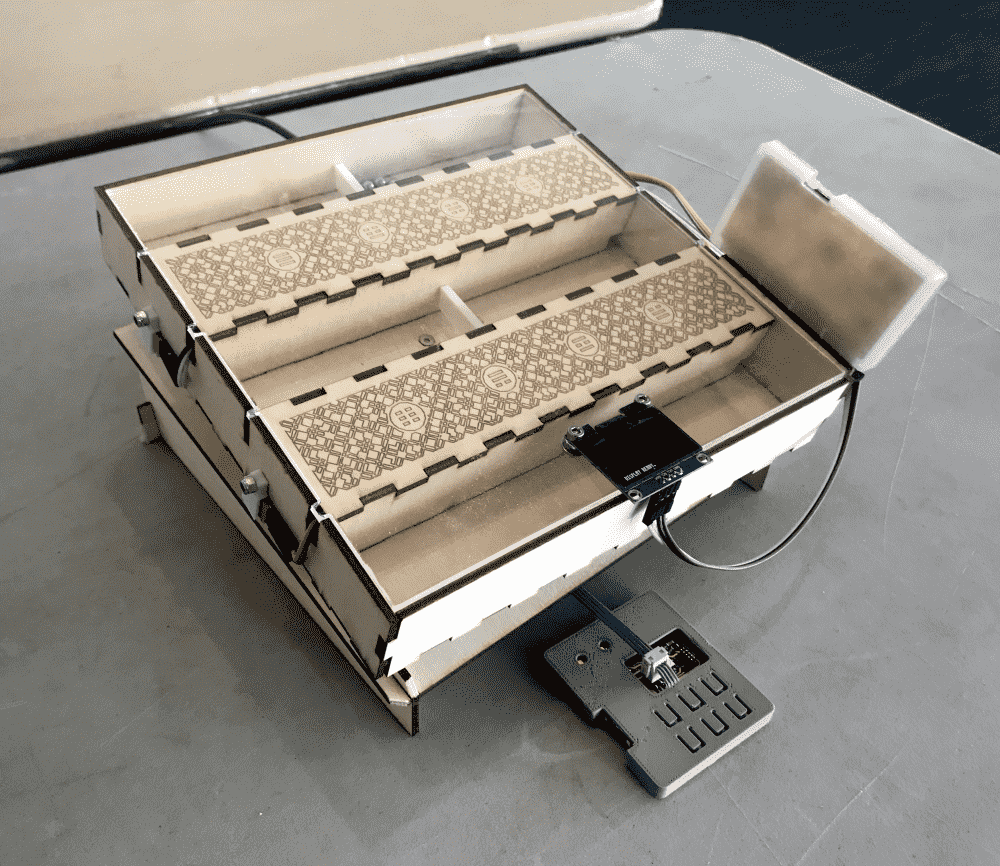My final project is an I-Ching divination machine, that fully automates the divination procedure described in the classic text (in a relatively authentic manner). For disbelievers of Tao, it can also be used as a physical random number generator.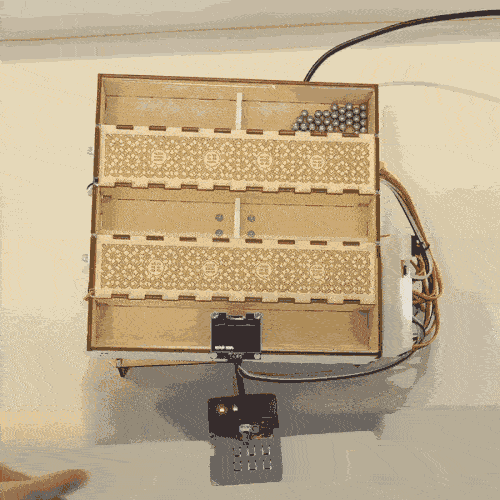I-Ching is a 3-thousand-year-old Chinese book used for a type of cleromancy, or prediction of the future using a random number generation. With divination being the “high tech” of its time, the method likely influenced the decision of many important people. Later the book became an important philosophical text due to commentaries added by later philosophers such as Confucius.

The method of divination (sans the symbolic gestures, with my rephrasing):

1. Take 49 sticks.
2. Arbitrarily divide them into two groups, with N and M each.
3. Find (N+3)%4 + 1, and discard that number of sticks away from N.
4. Find (M+2)%4 + 2, and discard that number of sticks away from M.
5. Combine the two groups of remaining sticks.
6. Repeat steps 2-5 three times.
7. The remaining sticks should be one of 24, 28, 32 and 36. Divide the number by 4, and record the quotient.
8. Repeat steps 1-7 six times.
9. Now we have six integer, each of them ranges from 6 to 9. The numbers correspond to Yin-Yang and young/old： 6 = old yin, 7 = young yang, 8 = young yin, 9 = old yang.
10. The next part is a bit hand wavy and require some understanding of Taoist philosophy, but you can understand it as such: Look up the numbers in a lookup table (i.e. I-Ching), which maps them to a fortune-telling message. The messages are usually very cryptic and ambiguous metaphors, and interpreting them which has been subject of research for thousands of years, is not in the scope of my machine. The machine only does the the random number generator part.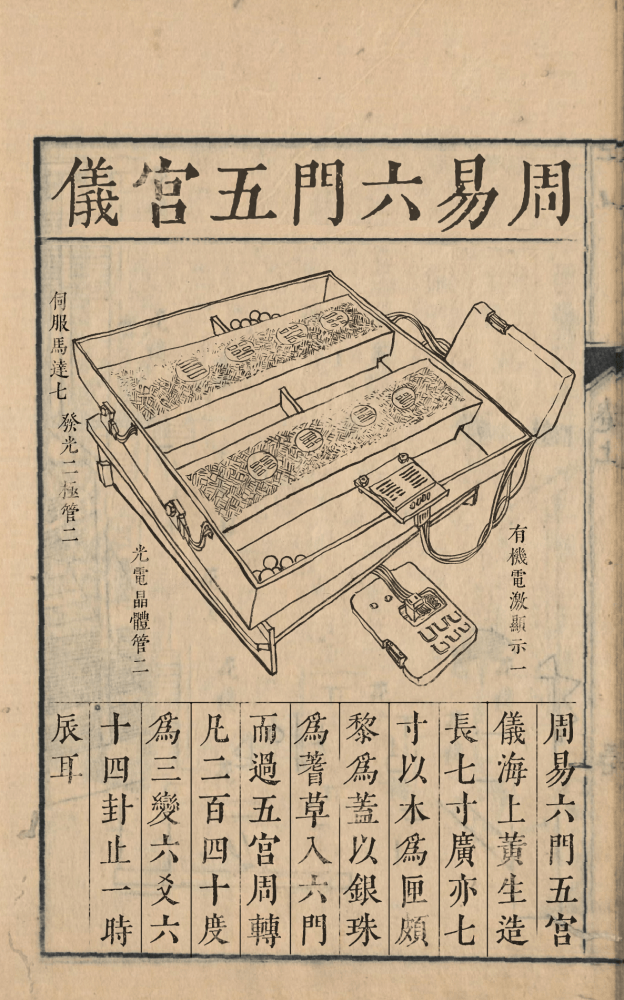As shown above, my design is an exact reconstruction based on an illustration of the machine found in an ancient Chinese book (just kidding).

In fact, my entire design is generated with a JavaScript program, found below. You can try the online demo here.

// unit: mm

const is_node = typeof process != 'undefined';
let createCanvas;
let findContours, approxPolyDP;
let fs;

if (is_node){
fs = require('fs');
;({createCanvas} = require('canvas'));
;({Image} = require('canvas'));
;({findContours,approxPolyDP} = require('./findcontours.js'));
var earcut = require('./earcut.js');
let img = new Image();
return img;
}
}else{
;({findContours, approxPolyDP} = FindContours);
createCanvas = function(w,h){
let cnv = document.createElement('canvas');
cnv.width = w;
cnv.height = h;
return cnv;
}
return document.querySelectorAll([src="${pth}"]); } var renderer; var scene; var camera; render_faces = function(faces){ if (!renderer){ scene = new THREE.Scene(); camera = new THREE.PerspectiveCamera( 75, 1, 0.1, 2000 ); camera.position.x = 0; camera.position.y = 0; camera.position.z = 100; camera.lookAt(0,0,0); renderer = new THREE.WebGLRenderer({}); renderer.setSize( 256,256 ); material = new THREE.MeshNormalMaterial(); } while(scene.children.length > 0){ scene.remove(scene.children); } // const controls = new THREE.OrbitControls( camera, renderer.domElement ); // controls.update(); let vertices = new Float32Array(faces.flat().map(xyz=>[xyz,xyz,xyz]).flat()); // console.log(faces); const geometry = new THREE.BufferGeometry(); geometry.setAttribute( 'position', new THREE.BufferAttribute( vertices, 3 ) ); geometry.setAttribute( 'normal', new THREE.BufferAttribute( new Float32Array(vertices.length).fill(0), 3 ) ); // geometry.computeFaceNormals(); geometry.computeBoundingBox(); let box = geometry.boundingBox; let siz = new THREE.Vector3(); box.getSize(siz); let mesh = new THREE.Mesh( geometry, material ); mesh.matrixAutoUpdate = true; scene.add(mesh); let cnvs = []; for (let i = 0; i < 12; i++){ geometry.rotateY(PI/6); geometry.computeVertexNormals(); geometry.attributes.normal.needsUpdate = true; geometry.computeBoundingBox(); let box = geometry.boundingBox; let cen = new THREE.Vector3(); box.getCenter(cen); camera.position.set(cen.x,cen.y,Math.max(siz.x,siz.y)*1.5+box.min.z+20); renderer.render( scene, camera ); let cnv = createCanvas(renderer.domElement.width,renderer.domElement.height); let ctx = cnv.getContext('2d'); ctx.drawImage(renderer.domElement,0,0); cnvs.push(cnv); } return cnvs; } download_stl = function(pth,faces){ let name = ${pth}-${new Date().getTime()}.stl; let data = to_stl_bin(faces); var a = document.createElement("a"); document.body.appendChild(a); a.style = "display: none"; var blob = new Blob([data], {type: "model/stl"}); var url = window.URL.createObjectURL(blob); a.href = url; a.download = name; a.click(); window.URL.revokeObjectURL(url); } download_svg = function(pth,text){ var element = document.createElement('a'); element.setAttribute('href', 'data:text/plain;charset=utf-8,' + encodeURIComponent(text)); element.setAttribute('download', pth); element.style.display = 'none'; document.body.appendChild(element); element.click(); document.body.removeChild(element); } } const PI = Math.PI; const cos = Math.cos; const sin = Math.sin; var WOOD_THICK = 3.15; // 25.4 * 1/8 var BEARING_GEAR_R = 22; var BEARING_INNER_R = 5.98; var BEARING_OUTER_R = 14.05; var BEARING_THICK = 8; var BEARING_LOWER_THICK = 6; var BEARING_CHAMF = 3; var BEARING_BOLT_THICK = 6; var BEARING_BOLT_R = 4.8; var BEARING_GEAR_THICK = 10; var BEARING_GEAR_HOLE_DX = 12; var BEARING_GEAR_HOLE_DY = 6; var BEARING_GEAR_TEETH = 18; // var BEARING_GEAR_R = 30; // var BEARING_INNER_R = 6.5; // var BEARING_OUTER_R = 13.5; // var BEARING_THICK = 8; // var BEARING_LOWER_THICK = 6; // var BEARING_CHAMF = 3; // var BEARING_BOLT_THICK = 6; // var BEARING_BOLT_R = 4.5; // var BEARING_GEAR_THICK = 10; // var BEARING_GEAR_HOLE_DX = 16; // var BEARING_GEAR_HOLE_DY = 8; // var BEARING_GEAR_TEETH = 18; var BEARING_BRACK_R = 18; var BEARING_BRACK_THICK = 13; var BEARING_BRACK_PL_THICK = 3; var BEARING_BRACK_PL_W = 36; var BEARING_BRACK_PL_H = 50; var BEARING_BRACK_PL_CHAMF = 3; var BEARING_BRACK_HOLE_DX = 13; var BEARING_BRACK_HOLE_DY = 21; // var BEARING_BRACK_R = 20; // var BEARING_BRACK_THICK = 13; // var BEARING_BRACK_PL_THICK = 3; // var BEARING_BRACK_PL_W = 40; // var BEARING_BRACK_PL_H = 60; // var BEARING_BRACK_PL_CHAMF = 3; // var BEARING_BRACK_HOLE_DX = 15; // var BEARING_BRACK_HOLE_DY = 25; var MG995_GEAR_RATIO = 1.5; var MG995_GEAR_R = BEARING_GEAR_R*MG995_GEAR_RATIO; var MG995_GEAR_TEETH = BEARING_GEAR_TEETH*MG995_GEAR_RATIO; var MG995_GEAR_THICK = BEARING_GEAR_THICK; var MG995_SINK_THICK = 4; var MG995_WIRE_THICK = 2; var MG995_HOLE_R = 2.05; var MG995_HOLE_DX = 3.55; var MG995_HOLE_DY = 4.75; var MG995_W = 40.4; var MG995_D = 20.15; var MG995_W_GAP = 0.6; var MG995_AXIS_RX = 30.15; var MG995_AXIS_LX = MG995_W-MG995_AXIS_RX; var NINEG_W = 22.7; var NINEG_D = 11.8; var NINEG_HOLE_DX = 2.5; var NINEG_HOLE_R = 1.05; var NINEG_HORN_THICK = 1.4; var NINEG_AXIS_LX = 6.2; var NINEG_AXIS_RX = NINEG_W-6.2; var NINEG_TOTAL_H = 31.6; var NINEG_LOWER_H = 15.8; var NINEG_CABLE_H = 8.2; var NINEG_CABLE_W = 3; var NINEG_ARM_W = 9; var NINEG_ARM_LEN = 50; var NINEG_ARM_STEM_LEN = 12; var NINEG_ARM_ROOT_LEN = 5; var NINEG_ARM_LEAF_LEN = NINEG_ARM_LEN-NINEG_ARM_ROOT_LEN-NINEG_ARM_STEM_LEN; var NINEG_ARM_THICK = 5; var NINEG_SINK_THICK = 3; var NINEG_LEAF_THICK = 1; var NINEG_BRACK_H = 9.5; var NINEG_BRACK_FRONT_THICK = 2.5; var NINEG_BRACK_SIDE_THICK = 1.5; var NINEG_BRACK_BACK_THICK = 2; var NINEG_BRACK_BOLT_THICK = 4; var NINEG_BRACK_TOP_THICK = 2.4; var NINEG_BRACK_W = 8; var NINEG_BRACK_HOLE_DX = 3.5; var NINEG_BRACK_HOLE_DY = 4.5; var NINEG_BRACK2_HOLE_DX = 3.31; var NINEG_BRACK2_HOLE_DY = 4.34; // var NINEG_BRACK2_HOLE_DX = 2.65; // var NINEG_BRACK2_HOLE_DY = 3; var NINEG_BRACK2_SINK_THICK = 5; var NINEG_BRACK2_BACK_THICK = 7; var NINEG_ASM_TOTAL_H = NINEG_TOTAL_H + NINEG_SINK_THICK - NINEG_HORN_THICK; var NINEG_ASM_TOTAL_W = NINEG_W+NINEG_BRACK_W*2; var M3_HOLE_D = 3.2; var MEZZ_H = Math.ceil(Math.max(40,NINEG_ASM_TOTAL_H,BEARING_GEAR_HOLE_DY*2+M3_HOLE_D*2)); var MEZZ_SIDE_SLOT_W = 8; var CHAMBER_H = 35; var CHAMBER_LW = 100; var CHAMBER_RW = 100; var BOX_W = CHAMBER_LW+CHAMBER_RW; var BOX_H = CHAMBER_H*3+MEZZ_H*2+WOOD_THICK*4; var BOX_D = 25; var BOX_X_FINGER_CNT = 17; var BOX_Y_FINGER_CNT = 17; var BOX_Z_FINGER_CNT = 3; var BASE_X_FINGER_CNT = 17; var BASE_Y_FINGER_CNT = 17; var BASE_W = 200; var BASE_H = 200; var BASE2_SLOT_DX = 6; var BASE2_SLOT_DY = 5; var BASE2_SLOT_LEN = 30; var BASE_RAIL_R = 80; var BASE_RAIL_W = 12; var BASE_DEG = 10; var BASE_ELEV_H = 20; var BASE_BACK_DX = 5; var BASE_BACK_H = 20; var LED_DY = 8.5; var LED_W = 8.78; var LED_R = 2.4; var LED_BRACK_W = 10; var LED_BRACK_H = 9.2; var LED_BRACK_INNER_H = 8.6; var LED_BRACK_THICK = 4; var LED_BRACK_SINK_THICK = 1.65; var LED_BRACK_PL_W = 6; var LED_BRACK_PL_H = 6; var LED_BRACK_PL_HOLE_DX = 3.5; var LED_BRACK_PL_HOLE_DY = 3; var LED_BRACK_PL_THICK = 3; var LED_BRACK_ROOF_W = 3; var LED_BRACK_ROOF_THICK = 1; var BALL_D = 7; var M3_HEXNUT_D = 5.51; var NINEG_ARM2_THICK = 4; var NINEG_ARM2_W = 13; var NINEG_ARM2_LEN = CHAMBER_H+WOOD_THICK+NINEG_AXIS_LX+NINEG_BRACK_W; var NINEG_ARM2_ROOT_LEN = 5; var NINEG_ARM2_STEM_LEN = 12; var NINEG_ARM2_LEAF_LEN = NINEG_ARM2_LEN-NINEG_ARM2_ROOT_LEN-NINEG_ARM2_STEM_LEN; var NINEG_ARM3_FLEX_THICK = 8; var NINEG_ARM3_STEM_THICK = 2.5; var NINEG_ARM3_STEM_LEN = 25; var NINEG_ARM3_FLEX_DY = 21; var TRIG_SLOPE_W = 9; var TRIG_SLOPE_THICK = BOX_D; var TRIG_SLOPE_SINK_THICK = 10; var TRIG_SLOPE2_W = 5; var TRIG_SLOPE2_THICK = BOX_D; var PCB_W = 75.1; var PCB_H = 39.1; // var PCB_THICK = 13; var PCB_BOARD_THICK = 1.65; var PCB_THICK = 4.8; var PCB_CASE_WALL_THICK = 3.5; var PCB_CASE_INNER_WALL_THICK = 1.5; var PCB_CASE_BOTTOM_THICK = 1.4; var PCB_CASE_TOP_THICK = 0.9; var PCB_CASE_CHAMF = 2.3; var PCB_CASE_CHAMF1 = 2; var PCB_CASE_CHAMF2 = 1; var PCB_CASE_W = PCB_W+PCB_CASE_WALL_THICK*2; var PCB_CASE_H = PCB_H+PCB_CASE_WALL_THICK*2; var PCB_CASE_TEETH_R = 0.5; var PCB_CASE_TEETH_THICK = 1.9; var PCB2_W = 68.48; // var PCB2_H = 47.3; var PCB2_H = 34.65; var PCB2_HOLE_DX = 4.4; var PCB2_HOLE_DY = 5.08; var PCB3_W = 54.1; var PCB3_H = 45.9; var PCB3_THICK = 4.75; var PCB3_CASE_WALL_THICK = 4.1; var PCB3_CASE_INNER_WALL_THICK = 1.7; var PCB3_CASE_BOTTOM_THICK = 1.8; var PCB3_CASE_TOP_THICK = 0.6; var PCB3_CASE_W = PCB3_W+PCB3_CASE_WALL_THICK*2; var PCB3_CASE_H = PCB3_H+PCB3_CASE_WALL_THICK*2; var PCB3_CASE_CHAMF = 3; var PCB3_CASE_CHAMF1 = 2; var PCB3_CASE_CHAMF2 = 1; // var PANEL_BRACK_DX = 5; var SENSOR_HOLE_DX = 15.7; var SENSOR_HOLE_DY = 11; var OLED_HOLE_DIST = 30; var OLED_HOLE_DY = 23; console.log(BOX_W,'x',BOX_H); let jsr = 0x5EED; function rand(){ jsr^=(jsr<<17); jsr^=(jsr>>13); jsr^=(jsr<<5); return (jsr>>>0)/4294967295; } function seg_isect(p0x, p0y, p1x, p1y, q0x, q0y, q1x, q1y, is_ray = false) { let d0x = p1x - p0x; let d0y = p1y - p0y; let d1x = q1x - q0x; let d1y = q1y - q0y; let vc = d0x * d1y - d0y * d1x; if (vc == 0) { return null; } let vcn = vc * vc; let q0x_p0x = q0x - p0x; let q0y_p0y = q0y - p0y; let vc_vcn = vc / vcn; let t = (q0x_p0x * d1y - q0y_p0y * d1x) * vc_vcn; let s = (q0x_p0x * d0y - q0y_p0y * d0x) * vc_vcn; if (0 <= t && (is_ray || t < 1) && 0 <= s && s < 1) { return t; } return null; } function dist(x0,y0,x1,y1){ return Math.hypot(x0-x1,y0-y1); } function trace(ctx,epsilon=1){ let cnv = ctx.canvas; let dat = ctx.getImageData(0,0,cnv.width,cnv.height).data; let im = []; for (let i = 0; i < dat.length; i+=4){ im.push(dat[i]>128?255:0); } let contours = findContours(im,cnv.width,cnv.height); for (let i = 0; i < contours.length; i++){ contours[i] = approxPolyDP(contours[i].points,epsilon); // contours[i] = contours[i].map(x=>[x,x]) } contours = contours.filter(x=>x.length>=3); return contours; } function draw_svg(polylines,W,H,SCALE=1){ W*=SCALE; H*=SCALE; polylines = scal_poly(polylines,SCALE); let o = <svg xmlns="http://www.w3.org/2000/svg" width="${W}" height="${H}"><path stroke="black" stroke-width="1" fill="none" d="M0 0${W} 0 ${W}${H} 0 ${H} z"/> for (let i = 0; i < polylines.length; i++){ o += <path stroke="black" stroke-width="0.5" fill="none" d="M for (let j = 0; j < polylines[i].length; j++){ let [x,y] = polylines[i][j]; o += ${x} ${y} ; } o += ' z"/>'; } o += </svg> return o; } function to_stl_bin(faces){ let nb = 84+faces.length*50; console.log(writing stl (binary)... estimated${Math.round((nb/1048576)*100)/100} MB);

let o = new Uint8Array(nb);
let a = new ArrayBuffer(4);
let b = new Uint32Array(a);
b = faces.length;
o.set(new Uint8Array(a),80);
for (let i = 0; i < faces.length; i++){
let d = [
faces[i],faces[i],faces[i],
faces[i],faces[i],faces[i],
faces[i],faces[i],faces[i],
]
let a = new ArrayBuffer(36);
let b = new Float32Array(a);
d.map((x,j)=>b[j]=x);
o.set(new Uint8Array(a),84+i*50+12);
}
return o;
}

function rot_pt_2d(x,y,th){
return [
x*cos(th)-y*sin(th),
x*sin(th)+y*cos(th),
]
}
function rot_path_2d(p,th){
return p.map(x=>rot_pt_2d(...x,th))
}

function involute(r){
let n = 100;
let p = [];
for (let i = 0; i < n; i++){
let t = i/n;
let a = t*PI/2;
let x = r*cos(a)+a*r*sin(a);
let y = r*sin(a)-a*r*cos(a);
p.push([x,y])
}
return p;
}

function circle(r,n=200){
let p = [];
for (let i = 0; i < n; i++){
let t = i/(n-1);
let a = t * PI*2;
let x = r*cos(a);
let y = r*sin(a);
p.push([x,y]);
}
return p;
}

function chamf_rect(w,h,r){
return [
[-w/2+r,-h/2],
[w/2-r,-h/2],
[w/2,-h/2+r],
[w/2,h/2-r],
[w/2-r,h/2],
[-w/2+r,h/2],
[-w/2,h/2-r],
[-w/2,-h/2+r],
]
}

function rect_xywh(x,y,w,h){
return [
[x,y],[x+w,y],[x+w,y+h],[x,y+h]
]
}

function fillet_rect(x,y,w,h,tl,tr,br,bl) {
let n = 10;
let poly = [];

if (tl){
for (let i = 0; i < n+1; i++) {
let a = (i/n)*PI/2;
let xx = x+tl-cos(a)*tl;
let yy = y+tl-sin(a)*tl;
poly.push([xx,yy]);
}
}else{
poly.push([x,y+tl]);
}
if (tr){
for (let i = 0; i < n+1; i++) {
let a = (i/n)*PI/2;
let xx = x+w-tr+sin(a)*tr;
let yy = y+tr-cos(a)*tr;
poly.push([xx,yy]);
}
}else{
poly.push([x+w-tr,y]);
}

if (br){
for (let i = 0; i < n+1; i++) {
let a = (i/n)*PI/2;
let xx = x+w-br+cos(a)*br;
let yy = y+h-br+sin(a)*br;
poly.push([xx,yy]);
}
}else{
poly.push([x+w,y+h-br])
}

if (bl){
for (let i = 0; i < n+1; i++) {
let a = (i/n)*PI/2;
let xx = x+bl-sin(a)*bl;
let yy = y+h-bl+cos(a)*bl;
poly.push([xx,yy]);
}
}else{
poly.push([x+bl,y+h])
}
return poly;
}

function semicircle(r,a0){
let n = 100;
let p = [];
for (let i = 0; i < n; i++){
let t = i/(n-1);
let a = a0+t * PI;
let x = r*cos(a);
let y = r*sin(a);
p.push([x,y]);
}
return p;
}
function isect_paths(p,q){
for (let i = 0; i < p.length-1; i++){
for (let j = 0; j < q.length-1; j++){
let a = p[i];
let b = p[i+1];
let c = q[j];
let d = q[j+1];
let t = seg_isect(...a,...b,...c,...d);
if (t!=null){
return [
a*(1-t)+b*t,
a*(1-t)+b*t,
]
}
}
}
}
function trim_path(p,q){
for (let i = 0; i < p.length-1; i++){
for (let j = 0; j < q.length-1; j++){
let a = p[i];
let b = p[i+1];
let c = q[j];
let d = q[j+1];
let t = seg_isect(...a,...b,...c,...d);
if (t!=null){
return p.slice(0,i+1).concat([[
a*(1-t)+b*t,
a*(1-t)+b*t,
]]);
}
}
}
return p;
}

function gear(r,n){
let pa = 0.11*PI;
let mod = r*2/n;
let ded = mod*1.157;

let r0 = cos(pa) * r;
let r1 = r + add;
let r2 = r - ded;

let inv = involute(r0);
let ref = circle(r);

let inn = circle(r2);
let out = circle(r1);

let ang = PI/n;

let p = isect_paths(inv,ref);

let a0 = Math.atan2(p,p);
a0*=2
let a1 = ang+a0;

let in1 = inv.map(x=>[x,-x]);

in1 = rot_path_2d(in1,a1);

let in0 = trim_path(inv,out);

let tip = trim_path(rot_path_2d(out,PI/2).reverse(),inv);
tip = trim_path(tip.reverse(),in1);

in1 = trim_path(in1,out);

let ac = ang-a0;
let rc = Math.tan(ac/2)*r0

let cc = semicircle(rc,PI/2)
cc = cc.map(x=>[x+r2,x]);

cc = rot_path_2d(cc,ang*1.5+a0/2);

inn = rot_path_2d(inn,PI/2)//.reverse();

in0 = trim_path(in0.reverse(),inn).reverse();
in1 = trim_path(in1.reverse(),inn);

let pl = [];

for (let i = 0; i < n; i++){
let ia = rot_path_2d(in0,ang*2*i);
let ib = rot_path_2d(in1,ang*2*i);
let ic = rot_path_2d(cc, ang*2*i);
let it = rot_path_2d(tip,ang*2*i);

pl.push(...ia,...it,...ib,...ic.reverse());
}
return approxPolyDP(rot_path_2d(pl,ang/2-a0/2-PI/n),0.05);
}

function dilate(ctx){
let ctx2 = createCanvas(ctx.canvas.width,ctx.canvas.height).getContext('2d');
ctx2.drawImage(ctx.canvas,0,0);
ctx2.globalCompositeOperation = "lighter";
ctx2.drawImage(ctx.canvas,-1,0);
ctx2.drawImage(ctx.canvas,0,-1);
ctx2.drawImage(ctx.canvas,0,1);
ctx2.drawImage(ctx.canvas,1,0);
ctx2.drawImage(ctx.canvas,-1,-1);
ctx2.drawImage(ctx.canvas,-1,1);
ctx2.drawImage(ctx.canvas,1,-1);
ctx2.drawImage(ctx.canvas,1,1);
return ctx2;
}

function erode(ctx){
let ctx2 = createCanvas(ctx.canvas.width,ctx.canvas.height).getContext('2d');
ctx2.drawImage(ctx.canvas,0,0);
ctx2.globalCompositeOperation = "multiply";
ctx2.drawImage(ctx.canvas,-1,0);
ctx2.drawImage(ctx.canvas,0,-1);
ctx2.drawImage(ctx.canvas,0,1);
ctx2.drawImage(ctx.canvas,1,0);
ctx2.drawImage(ctx.canvas,-1,-1);
ctx2.drawImage(ctx.canvas,-1,1);
ctx2.drawImage(ctx.canvas,1,-1);
ctx2.drawImage(ctx.canvas,1,1);
return ctx2;
}

function trsl_poly(poly,x,y){
return poly.map(xys=>xys.map(xy=>[xy+x,xy+y]));
}
function scal_poly(poly,x){
return poly.map(xys=>xys.map(xy=>[xy*x,xy*x]));
}

function trsl_mesh(mesh,x,y,z){
return mesh.map(xys=>xys.map(xy=>[xy+x,xy+y,xy+z]));
}

function extrude(p,d){
let ft = triangulate(p);
let f1 = ft.map(xys=>xys.map(xy=>[xy,xy,0]).reverse());
let f2 = ft.map(xys=>xys.map(xy=>[xy,xy,d]));

let ff = [...f1,...f2]
for (let k = 0; k < p.length; k++){
let vs = p[k];
for (let i = 0; i < vs.length; i++){
let j = (i+1)%vs.length;
let a = [...vs[i],0]
let b = [...vs[j],0]
let c = [...vs[i],d]
let e = [...vs[j],d]
if (!k){
ff.push([a,b,e],[a,e,c]);
}else{
ff.push([a,e,b],[a,c,e]);
}

}
}
return ff;
}

function make_tube(rs,zs,n){
let cs = [];
for (let i = 0; i < rs.length; i++){
let c = [];
for (let j = 0; j < n; j++){
let a = j/n*PI*2;
let x = cos(a)*rs[i];
let y = sin(a)*rs[i];
c.push([x,y,zs[i]]);
}
cs.push(c);
}
let ff = [];
for (let i = 0; i < cs.length-1; i++){
for (let j = 0; j < n; j++){
let a = cs[i][j];
let b = cs[i][(j+1)%n];
let c = cs[i+1][j]
let d = cs[i+1][(j+1)%n];
ff.push([a,b,d],[a,d,c]);
}
}
return ff;
}

function clone(p){
return JSON.parse(JSON.stringify(p));
}

function triangulate(p){
p = clone(p);
let poly = p;
let holes = p.slice(1);
let idx = [];
let q = poly;
while (holes.length){
idx.push(q.length);
q.push(...holes.pop());
}
let trigs = earcut(q.flat(),idx.length?idx:null);

let faces = [];
for (let i = 0; i < trigs.length; i+=3){
let a = trigs[i];
let b = trigs[i+1];
let c = trigs[i+2];
faces.push([q[a],q[b],q[c]]);
}
return faces;
}

function get_bbox(points){
let xmin = Infinity;
let ymin = Infinity;
let xmax = -Infinity;
let ymax = -Infinity
for (let i = 0;i < points.length; i++){
let [x,y] = points[i];
xmin = Math.min(xmin,x);
ymin = Math.min(ymin,y);
xmax = Math.max(xmax,x);
ymax = Math.max(ymax,y);
}
return {x:xmin,y:ymin,w:xmax-xmin,h:ymax-ymin};
}

function export_model(pth,faces){
console.log(pth);
let s = 1;//1/25.4;
faces = faces.map(xys=>xys.map(xy=>[xy*s,xy*s,xy*s]));
if (is_node){
let stl = to_stl_bin(faces);
fs.writeFileSync(pth,stl);
}else{
let div = document.getElementById(pth);
if (!div){
div = document.createElement("div");
div.id = pth;
div.style = "display:inline-block;margin:1px";
document.body.appendChild(div);
}
div.innerHTML = <div>${pth} <button>download</button></div>; div.getElementsByTagName("button").onclick = function(){ download_stl(pth,faces); } let cnv = document.createElement("canvas"); let ctx = cnv.getContext('2d'); let cnvs = window.render_faces(faces); cnv.width = cnvs.width; cnv.height = cnvs.height; let idx = 0; clearTimeout(window['loop_'+pth]); function loop(){ window['loop_'+pth] = setTimeout(loop,100); ctx.drawImage(cnvs[idx],0,0); idx = (idx+1)%cnvs.length; } loop(); div.appendChild(cnv); } } // function export_outline(pth,polylines){ // console.log(pth); // // polylines = scal_poly(polylines,1/25.4); // let bb = get_bbox(polylines.flat()); // polylines = trsl_poly(polylines,-bb.x+10,-bb.y+10); // fs.writeFileSync(pth,draw_svg(polylines,bb.w+20,bb.h+20)); // } function export_outline(pth,polylines){ console.log(pth); // polylines = scal_poly(polylines,1/25.4); let bb = get_bbox(polylines.flat()); polylines = trsl_poly(polylines,-bb.x+10,-bb.y+10); let svg = draw_svg(polylines,256,256); if (is_node){ fs.writeFileSync(pth,svg); }else{ let div = document.getElementById(pth); if (!div){ div = document.createElement("div"); div.id = pth; div.style = "display:inline-block;margin:1px"; document.body.appendChild(div); } div.innerHTML = <div>${pth} <button>download</button></div><div>${svg}</div>; div.getElementsByTagName("button").onclick = function(){ download_svg(pth,svg); } } } function load_mg995_horn(){ let img = readImage("horn_mg995.png"); let cnv = createCanvas(img.width,img.height); let ctx = cnv.getContext('2d'); ctx.drawImage(img,0,0); // let polylines = trace(ctx,1.5); let ctx2 = dilate(ctx); ctx2 = dilate(ctx2); ctx2 = dilate(ctx2); let polylines = [trace(ctx2,1.4)]; let ctx3 = erode(ctx); ctx3 = erode(ctx3); polylines.push(...trace(ctx3,1.4).slice(1)); // polylines = polylines.concat(trace(ctx,1.5)); // fs.writeFileSync('out.svg',draw_svg(polylines,1024,1024)); polylines = polylines.map(xys=>xys.map(xy=>[ (xy-512)/600*25.4, (xy-512)/600*25.4, ])); // console.log(polylines) return polylines; } function load_9g_horn(offset){ let img = readImage("horn_9g.png"); let cnv = createCanvas(img.width,img.height); let ctx = cnv.getContext('2d'); let dy = offset/25.4*600; ctx.drawImage(img,0,512+dy); // let polylines = trace(ctx,1.5); let ctx2 = dilate(ctx) let polylines = [trace(ctx2,1.4)]; let ctx3 = erode(ctx); ctx3 = erode(ctx3); ctx3 = erode(ctx3); polylines.push(...trace(ctx3,1.4).slice(1)); // polylines = polylines.concat(trace(ctx,1.5)); // fs.writeFileSync('out.svg',draw_svg(polylines,1024,1024)); polylines = polylines.map(xys=>xys.map(xy=>[ (512-xy)/600*25.4, (1024-xy)/600*25.4, ])); // console.log(polylines) return polylines; } function load_flexture_door(){ let img = readImage("flexture_door.png"); let cnv = createCanvas(img.width,img.height); let ctx = cnv.getContext('2d'); ctx.drawImage(img,0,0); // let polylines = trace(ctx,1.5); let polylines = [trace(ctx,1.4)]; // polylines = polylines.concat(trace(ctx,1.5)); // fs.writeFileSync('out.svg',draw_svg(polylines,1024,1024)); polylines = polylines.map(xys=>xys.map(xy=>[ (xy-51)/21, (xy-225)/21, ]).reverse()); // console.log(polylines) return polylines; } function hole(x,y,r){ return circle(r,32).map(xy=>[xy+x,xy+y]); } function m3_hole(x,y){ return hole(x,y,M3_HOLE_D/2); } function m3_hexnut_hole(x,y){ let r = M3_HEXNUT_D/2/Math.sqrt(3)*2; let o = []; for (let i = 0; i < 6; i++){ let a = i/6 * PI * 2; let u = Math.cos(a)*r; let v = Math.sin(a)*r; o.push([x+u,y+v]); } return o; } function mg995_gear(){ let g0 = gear(MG995_GEAR_R,MG995_GEAR_TEETH); let hn = load_mg995_horn(); hn = [hn,hn,hn,hn,hn]; // let g1 = gear(bearing_gear_r,bearing_gear_teeth); // fs.writeFileSync('out.svg',draw_svg([ // ...trsl_poly([g0,...hn],100,100),...trsl_poly([g1],200,100) // ],300,200,2)); let f0 = [g0].concat(hn.slice(1)); let f1 = [g0].concat([hn.slice().reverse()]); // let ff = triangulate(f1); // fs.writeFileSync('out.svg',draw_svg([ // ...trsl_poly(ff,100,100), // ],300,200,2)); let d0 = MG995_GEAR_THICK-MG995_SINK_THICK-MG995_WIRE_THICK; let part0 = extrude(f1, MG995_SINK_THICK); let part1 = trsl_mesh(extrude(f0, d0),0,0,MG995_SINK_THICK); let part2 = trsl_mesh(extrude(f1, MG995_WIRE_THICK),0,0,d0+MG995_SINK_THICK); // console.log(part0); export_model('part_mg995_gear.stl', part0.concat(part1).concat(part2)) } function bearing_gear(){ let g0 = gear(BEARING_GEAR_R,BEARING_GEAR_TEETH); let f0 = [g0].concat([ m3_hole(-BEARING_GEAR_HOLE_DX,-BEARING_GEAR_HOLE_DY), m3_hole( BEARING_GEAR_HOLE_DX,-BEARING_GEAR_HOLE_DY), m3_hole(-BEARING_GEAR_HOLE_DX, BEARING_GEAR_HOLE_DY), m3_hole( BEARING_GEAR_HOLE_DX, BEARING_GEAR_HOLE_DY), ]); let d0 = BEARING_GEAR_THICK-BEARING_BOLT_THICK; let part0 = extrude(f0, d0); // let f1 = [g0].concat([ // hole(-BEARING_GEAR_HOLE_DX,-BEARING_GEAR_HOLE_DY,BEARING_BOLT_R), // hole( BEARING_GEAR_HOLE_DX,-BEARING_GEAR_HOLE_DY,BEARING_BOLT_R), // hole(-BEARING_GEAR_HOLE_DX, BEARING_GEAR_HOLE_DY,BEARING_BOLT_R), // hole( BEARING_GEAR_HOLE_DX, BEARING_GEAR_HOLE_DY,BEARING_BOLT_R), // ]); let f1 = [g0].concat([ m3_hexnut_hole(-BEARING_GEAR_HOLE_DX,-BEARING_GEAR_HOLE_DY), m3_hexnut_hole( BEARING_GEAR_HOLE_DX,-BEARING_GEAR_HOLE_DY), m3_hexnut_hole(-BEARING_GEAR_HOLE_DX, BEARING_GEAR_HOLE_DY), m3_hexnut_hole( BEARING_GEAR_HOLE_DX, BEARING_GEAR_HOLE_DY), ]); let part1 = trsl_mesh(extrude(f1, BEARING_BOLT_THICK),0,0,d0); let part2 = make_tube( [BEARING_INNER_R,BEARING_INNER_R,BEARING_INNER_R-BEARING_CHAMF,0], [ BEARING_GEAR_THICK, BEARING_GEAR_THICK+BEARING_THICK, BEARING_GEAR_THICK+BEARING_THICK+BEARING_CHAMF, BEARING_GEAR_THICK+BEARING_THICK+BEARING_CHAMF ],64); export_model('part_bearing_gear.stl', part0.concat(part1).concat(part2)); } function bearing_bracket(){ let g0 = circle(BEARING_BRACK_R,64); let g1 = circle(BEARING_OUTER_R,64); let g2 = circle(BEARING_INNER_R,64); let f0 = [g0].concat([g1]) let part0 = extrude(f0, BEARING_LOWER_THICK); let d1 = BEARING_BRACK_THICK-BEARING_BRACK_PL_THICK-BEARING_LOWER_THICK; // let part1 = make_tube( // [0,BEARING_BRACK_R,BEARING_BRACK_R,0], // [BEARING_LOWER_THICK,BEARING_LOWER_THICK,BEARING_LOWER_THICK+d1,BEARING_LOWER_THICK+d1],64 // ); let f1 = [g0].concat([g2]) let part1 = trsl_mesh(extrude(f1, d1), 0,0,BEARING_LOWER_THICK); let g3 = chamf_rect(BEARING_BRACK_PL_W,BEARING_BRACK_PL_H,BEARING_BRACK_PL_CHAMF); let f2 = [g3].concat([ m3_hole(-BEARING_BRACK_HOLE_DX,-BEARING_BRACK_HOLE_DY), m3_hole( BEARING_BRACK_HOLE_DX,-BEARING_BRACK_HOLE_DY), m3_hole(-BEARING_BRACK_HOLE_DX, BEARING_BRACK_HOLE_DY), m3_hole( BEARING_BRACK_HOLE_DX, BEARING_BRACK_HOLE_DY), ]); let part2 = trsl_mesh(extrude(f2, BEARING_BRACK_PL_THICK),0,0,BEARING_LOWER_THICK+d1); export_model('part_bearing_bracket.stl', part0.concat(part1).concat(part2)); } function nineg_arm(){ let hn = load_9g_horn(5); // hn.reverse(); hn.splice(1,1); hn.splice(2,1); hn.splice(3,1); let fpi = 0; let hn0 = hn; for (let i = 0; i < hn0.length; i++){ if (hn0[i] < hn0[fpi]){ fpi = i; }else if (hn0[i] < hn0[fpi]+0.001 && hn0[i] < hn0[fpi]){ fpi = i; } } hn0 = hn0.slice(fpi).concat(hn0.slice(0,fpi)); let g0 = [ [NINEG_ARM_W/2,0], [NINEG_ARM_W/2,NINEG_ARM_STEM_LEN], [-NINEG_ARM_W/2,NINEG_ARM_STEM_LEN], [-NINEG_ARM_W/2,0] ]; let f0 = [[...hn0,...g0]]; let part0 = extrude(f0, NINEG_SINK_THICK); let f1 = [g0].concat(hn.slice(1)); let part1 = trsl_mesh(extrude(f1, NINEG_ARM_THICK-NINEG_SINK_THICK),0,0,NINEG_SINK_THICK); let part2 = trsl_mesh(extrude([[ [NINEG_ARM_W/2,0], [NINEG_ARM_W/2,NINEG_ARM_LEAF_LEN], [-NINEG_ARM_W/2,NINEG_ARM_LEAF_LEN], [-NINEG_ARM_W/2,0] ]],NINEG_LEAF_THICK),0,NINEG_ARM_STEM_LEN,0); let l0 = NINEG_ARM_STEM_LEN; let l1 = NINEG_ARM_LEN-NINEG_ARM_ROOT_LEN; let w = NINEG_ARM_W; let part3 = [ [[-w/2,l1,NINEG_LEAF_THICK],[0,l0,NINEG_ARM_THICK],[w/2,l1,NINEG_LEAF_THICK]], [[-w/2,l1,NINEG_LEAF_THICK],[-w/2,l0,NINEG_LEAF_THICK],[0,l0,NINEG_ARM_THICK]], [[w/2,l1,NINEG_LEAF_THICK],[0,l0,NINEG_ARM_THICK],[w/2,l0,NINEG_LEAF_THICK]], [[0,l0,NINEG_ARM_THICK],[-w/2,l0,NINEG_LEAF_THICK],[w/2,l0,NINEG_LEAF_THICK]], [[-w/2,l1,NINEG_LEAF_THICK],[w/2,l1,NINEG_LEAF_THICK],[w/2,l0,NINEG_LEAF_THICK]], [[-w/2,l1,NINEG_LEAF_THICK],[w/2,l0,NINEG_LEAF_THICK],[-w/2,l0,NINEG_LEAF_THICK]], ] export_model('part_9g_arm.stl', part0.concat(part1).concat(part2).concat(part3)); } function nineg_arm2(){ let hn = load_9g_horn(5); // hn.reverse(); hn.splice(1,1); hn.splice(2,1); hn.splice(3,1); let fpi = 0; let hn0 = hn; for (let i = 0; i < hn0.length; i++){ if (hn0[i] < hn0[fpi]){ fpi = i; }else if (hn0[i] < hn0[fpi]+0.001 && hn0[i] < hn0[fpi]){ fpi = i; } } hn0 = hn0.slice(fpi).concat(hn0.slice(0,fpi)); let g0 = [ [NINEG_ARM_W/2,0], [NINEG_ARM_W/2,NINEG_ARM2_STEM_LEN], [-NINEG_ARM_W/2,NINEG_ARM2_STEM_LEN], [-NINEG_ARM_W/2,0] ]; let f0 = [[...hn0,...g0]]; let part0 = extrude(f0, NINEG_SINK_THICK); let f1 = [g0].concat(hn.slice(1)); let part1 = trsl_mesh(extrude(f1, NINEG_ARM2_THICK-NINEG_SINK_THICK),0,0,NINEG_SINK_THICK); let l0 = NINEG_ARM2_STEM_LEN; let l1 = NINEG_ARM2_LEN-NINEG_ARM2_ROOT_LEN; let w = NINEG_ARM_W/2-NINEG_ARM2_W; let part2 = [ [[w,l0,0],[w,l1,NINEG_ARM2_THICK],[w,l1,0]], [[w,l0,0],[w,l0,NINEG_ARM2_THICK],[w,l1,NINEG_ARM2_THICK]], [[w,l0,0],[w,l1,0],[NINEG_ARM_W/2,l0,NINEG_ARM2_THICK/2]], [[w,l1,0],[NINEG_ARM_W/2,l1,NINEG_ARM2_THICK/2],[NINEG_ARM_W/2,l0,NINEG_ARM2_THICK/2]], [[w,l0,NINEG_ARM2_THICK],[NINEG_ARM_W/2,l0,NINEG_ARM2_THICK/2],[w,l1,NINEG_ARM2_THICK]], [[w,l1,NINEG_ARM2_THICK],[NINEG_ARM_W/2,l0,NINEG_ARM2_THICK/2],[NINEG_ARM_W/2,l1,NINEG_ARM2_THICK/2]], [[w,l1,0],[w,l1,NINEG_ARM2_THICK],[NINEG_ARM_W/2,l1,NINEG_ARM2_THICK/2]], [[w,l0,0],[NINEG_ARM_W/2,l0,NINEG_ARM2_THICK/2],[w,l0,NINEG_ARM2_THICK]], ] export_model('part_9g_arm2.stl', part0.concat(part1).concat(part2)); } function nineg_arm3(){ let hn = load_9g_horn(5); // hn.reverse(); hn.splice(1,1); hn.splice(2,1); hn.splice(3,1); let fpi = 0; let hn0 = hn; for (let i = 0; i < hn0.length; i++){ if (hn0[i] < hn0[fpi]){ fpi = i; }else if (hn0[i] < hn0[fpi]+0.001 && hn0[i] < hn0[fpi]){ fpi = i; } } hn0 = hn0.slice(fpi).concat(hn0.slice(0,fpi)); let g0 = [ [NINEG_ARM_W/2,0], [NINEG_ARM_W/2,NINEG_ARM3_STEM_LEN], [-NINEG_ARM_W/2,NINEG_ARM3_STEM_LEN], [-NINEG_ARM_W/2,0] ]; let f0 = [[...hn0,...g0]]; let part0 = extrude(f0, NINEG_SINK_THICK); let f1 = [g0].concat(hn.slice(1)); let part1 = trsl_mesh(extrude(f1, NINEG_ARM_THICK-NINEG_SINK_THICK),0,0,NINEG_SINK_THICK); let part2 = trsl_mesh(extrude([[ [NINEG_ARM_W/2,0], [NINEG_ARM_W/2,NINEG_ARM3_STEM_THICK], [-NINEG_ARM_W/2,NINEG_ARM3_STEM_THICK], [-NINEG_ARM_W/2,0] ]],MEZZ_H/2+NINEG_ARM3_STEM_THICK/2),0,NINEG_ARM3_STEM_LEN,0); let part3 = trsl_mesh(extrude([[ [0,0], [NINEG_ARM_W,0], [NINEG_ARM_W,NINEG_ARM_LEN-NINEG_ARM_ROOT_LEN-NINEG_ARM3_STEM_LEN-NINEG_ARM3_FLEX_THICK-NINEG_ARM3_STEM_THICK], [0,NINEG_ARM_LEN-NINEG_ARM_ROOT_LEN-NINEG_ARM3_STEM_LEN-NINEG_ARM3_FLEX_THICK-NINEG_ARM3_STEM_THICK] ]],NINEG_ARM3_STEM_THICK),-NINEG_ARM_W/2,NINEG_ARM3_STEM_LEN+NINEG_ARM3_STEM_THICK,MEZZ_H/2-NINEG_ARM3_STEM_THICK/2); let part32 = trsl_mesh(extrude([[ [0,0], [NINEG_ARM_W,0], [NINEG_ARM_W,NINEG_ARM_LEN-NINEG_ARM_ROOT_LEN-NINEG_ARM3_STEM_LEN-NINEG_ARM3_FLEX_THICK-NINEG_ARM3_STEM_THICK], [0,NINEG_ARM_LEN-NINEG_ARM_ROOT_LEN-NINEG_ARM3_STEM_LEN-NINEG_ARM3_FLEX_THICK-NINEG_ARM3_STEM_THICK] ]],NINEG_ARM3_STEM_THICK),-NINEG_ARM_W/2,NINEG_ARM3_STEM_LEN+NINEG_ARM3_STEM_THICK,0); let l0 = NINEG_ARM3_STEM_LEN; let l1 = NINEG_ARM_LEN-NINEG_ARM_ROOT_LEN; let w = NINEG_ARM_W; let part4 = trsl_mesh(extrude([[ [0,0], [NINEG_ARM_W,0], [NINEG_ARM_W,NINEG_ARM3_FLEX_THICK], [0,NINEG_ARM3_FLEX_THICK] ]],MEZZ_H-19.05),-NINEG_ARM_W/2,l1-NINEG_ARM3_FLEX_THICK,0); let fd = load_flexture_door(); let part5 = extrude(fd,NINEG_ARM3_FLEX_THICK).map(xys=>xys.map(xy=>[-xy-NINEG_ARM_W/2,xy+l1-NINEG_ARM3_FLEX_THICK,xy+NINEG_ARM3_FLEX_DY]).reverse()) export_model('part_9g_arm3.stl', part0.concat(part1).concat(part2).concat(part3).concat(part32).concat(part4).concat(part5)); } function nineg_arm3_noflex(){ let hn = load_9g_horn(5); // hn.reverse(); hn.splice(1,1); hn.splice(2,1); hn.splice(3,1); let fpi = 0; let hn0 = hn; for (let i = 0; i < hn0.length; i++){ if (hn0[i] < hn0[fpi]){ fpi = i; }else if (hn0[i] < hn0[fpi]+0.001 && hn0[i] < hn0[fpi]){ fpi = i; } } hn0 = hn0.slice(fpi).concat(hn0.slice(0,fpi)); let g0 = [ [NINEG_ARM_W/2,0], [NINEG_ARM_W/2,NINEG_ARM3_STEM_LEN], [-NINEG_ARM_W/2,NINEG_ARM3_STEM_LEN], [-NINEG_ARM_W/2,0] ]; let f0 = [[...hn0,...g0]]; let part0 = extrude(f0, NINEG_SINK_THICK); let f1 = [g0].concat(hn.slice(1)); let part1 = trsl_mesh(extrude(f1, NINEG_ARM_THICK-NINEG_SINK_THICK),0,0,NINEG_SINK_THICK); let part2 = trsl_mesh(extrude([[ [NINEG_ARM_W/2,0], [NINEG_ARM_W/2,NINEG_ARM3_STEM_THICK], [-NINEG_ARM_W/2,NINEG_ARM3_STEM_THICK], [-NINEG_ARM_W/2,0] ]],MEZZ_H/2+NINEG_ARM3_STEM_THICK/2),0,NINEG_ARM3_STEM_LEN,0); let part3 = trsl_mesh(extrude([[ [0,0], [NINEG_ARM_W,0], [NINEG_ARM_W,NINEG_ARM_LEN-NINEG_ARM_ROOT_LEN-NINEG_ARM3_STEM_LEN-NINEG_ARM3_FLEX_THICK-NINEG_ARM3_STEM_THICK], [0,NINEG_ARM_LEN-NINEG_ARM_ROOT_LEN-NINEG_ARM3_STEM_LEN-NINEG_ARM3_FLEX_THICK-NINEG_ARM3_STEM_THICK] ]],NINEG_ARM3_STEM_THICK),-NINEG_ARM_W/2,NINEG_ARM3_STEM_LEN+NINEG_ARM3_STEM_THICK,MEZZ_H/2-NINEG_ARM3_STEM_THICK/2); let part32 = trsl_mesh(extrude([[ [0,0], [NINEG_ARM_W,0], [NINEG_ARM_W,NINEG_ARM_LEN-NINEG_ARM_ROOT_LEN-NINEG_ARM3_STEM_LEN-NINEG_ARM3_FLEX_THICK-NINEG_ARM3_STEM_THICK], [0,NINEG_ARM_LEN-NINEG_ARM_ROOT_LEN-NINEG_ARM3_STEM_LEN-NINEG_ARM3_FLEX_THICK-NINEG_ARM3_STEM_THICK] ]],NINEG_ARM3_STEM_THICK),-NINEG_ARM_W/2,NINEG_ARM3_STEM_LEN+NINEG_ARM3_STEM_THICK,0); let l0 = NINEG_ARM3_STEM_LEN; let l1 = NINEG_ARM_LEN-NINEG_ARM_ROOT_LEN; let w = NINEG_ARM_W; let part4 = trsl_mesh(extrude([[ [0,0], [NINEG_ARM_W,0], [NINEG_ARM_W,NINEG_ARM3_FLEX_THICK-1.2], [0,NINEG_ARM3_FLEX_THICK-1.2] ]],MEZZ_H),-NINEG_ARM_W/2,l1-NINEG_ARM3_FLEX_THICK,0); export_model('part_9g_arm3_noflex.stl', part0.concat(part1).concat(part2).concat(part3).concat(part4)); } function nineg_bracket(){ // let part0 = trsl_mesh(extrude([[ // [-NINEG_W/2,NINEG_BRACK_TOP_THICK+NINEG_BRACK_BOLT_THICK], // [0,NINEG_BRACK_TOP_THICK+NINEG_BRACK_BOLT_THICK], // [0,NINEG_BRACK_H], // [-NINEG_W/2,NINEG_BRACK_H], // ]],NINEG_BRACK_FRONT_THICK),0,0,NINEG_D); let part0 = trsl_mesh(extrude([[ [-NINEG_W/2,0], [0,0], [0,NINEG_BRACK_H], [-NINEG_W/2,NINEG_BRACK_H], ]],NINEG_BRACK_FRONT_THICK),0,0,NINEG_D); let part1 = trsl_mesh(extrude([[ [0,NINEG_BRACK_TOP_THICK+NINEG_BRACK_BOLT_THICK], [NINEG_BRACK_SIDE_THICK,NINEG_BRACK_TOP_THICK+NINEG_BRACK_BOLT_THICK], [NINEG_BRACK_SIDE_THICK,NINEG_BRACK_H], [0,NINEG_BRACK_H], ]],NINEG_D+NINEG_BRACK_FRONT_THICK-NINEG_BRACK_BACK_THICK),0,0,NINEG_BRACK_BACK_THICK); let part2 = extrude([[ [0,NINEG_BRACK_TOP_THICK], [NINEG_BRACK_W,NINEG_BRACK_TOP_THICK], [NINEG_BRACK_W,NINEG_BRACK_H], [0,NINEG_BRACK_H], ], m3_hole(NINEG_BRACK_HOLE_DX+NINEG_BRACK_SIDE_THICK,NINEG_BRACK_HOLE_DY+NINEG_BRACK_TOP_THICK) ],NINEG_BRACK_BACK_THICK); let part3 = extrude([[ [0,0], [NINEG_BRACK_W,0], [NINEG_BRACK_W,NINEG_D+NINEG_BRACK_FRONT_THICK], [0,NINEG_D+NINEG_BRACK_FRONT_THICK], ], //hole(NINEG_HOLE_DX,NINEG_D/2,NINEG_HOLE_R) ],NINEG_BRACK_TOP_THICK).map(xys=>xys.map(xy=>[xy,xy,xy]).reverse()); let part4 = extrude([ hole(NINEG_HOLE_DX,NINEG_D/2,NINEG_HOLE_R) ],-NINEG_BRACK_TOP_THICK).map(xys=>xys.map(xy=>[xy,xy,xy])); let partl = trsl_mesh(part0.concat(part1).concat(part2).concat(part3).concat(part4),NINEG_W/2,0,0); let partr = partl.map(xys=>xys.map(xy=>[-xy,xy,xy]).reverse()); export_model('part_9g_bracket.stl', partl.concat(partr)); } function nineg_bracket2(){ let part0 = trsl_mesh(extrude([[ [-NINEG_W/2,0], [0,0], [0,NINEG_BRACK_H], [-NINEG_W/2,NINEG_BRACK_H], ]],NINEG_BRACK_FRONT_THICK),0,0,NINEG_D); let part1 = trsl_mesh(extrude([[ [0,NINEG_BRACK_TOP_THICK+NINEG_BRACK_BOLT_THICK], [NINEG_BRACK_SIDE_THICK,NINEG_BRACK_TOP_THICK+NINEG_BRACK_BOLT_THICK], [NINEG_BRACK_SIDE_THICK,NINEG_BRACK_H], [0,NINEG_BRACK_H], ]],NINEG_D+NINEG_BRACK_FRONT_THICK-NINEG_BRACK2_BACK_THICK),0,0,NINEG_BRACK2_BACK_THICK); let part2 = extrude([[ [0,NINEG_BRACK_TOP_THICK], [NINEG_BRACK_W,NINEG_BRACK_TOP_THICK], [NINEG_BRACK_W,NINEG_BRACK_H], [0,NINEG_BRACK_H], ], m3_hole(NINEG_BRACK2_HOLE_DX+NINEG_BRACK_SIDE_THICK,NINEG_BRACK2_HOLE_DY+NINEG_BRACK_TOP_THICK) ],NINEG_BRACK2_BACK_THICK-NINEG_BRACK2_SINK_THICK); let part5 = trsl_mesh(extrude([[ [0,NINEG_BRACK_TOP_THICK], [NINEG_BRACK_W,NINEG_BRACK_TOP_THICK], [NINEG_BRACK_W,NINEG_BRACK_H], [0,NINEG_BRACK_H], ], m3_hexnut_hole(NINEG_BRACK2_HOLE_DX+NINEG_BRACK_SIDE_THICK,NINEG_BRACK2_HOLE_DY+NINEG_BRACK_TOP_THICK) ],NINEG_BRACK2_SINK_THICK),0,0,NINEG_BRACK2_BACK_THICK-NINEG_BRACK2_SINK_THICK); let part3 = extrude([[ [0,0], [NINEG_BRACK_W,0], [NINEG_BRACK_W,NINEG_D+NINEG_BRACK_FRONT_THICK], [0,NINEG_D+NINEG_BRACK_FRONT_THICK], ], //hole(NINEG_HOLE_DX,NINEG_D/2,NINEG_HOLE_R) ],NINEG_BRACK_TOP_THICK).map(xys=>xys.map(xy=>[xy,xy,xy]).reverse()); let part4 = extrude([ hole(NINEG_HOLE_DX,NINEG_D/2,NINEG_HOLE_R) ],-NINEG_BRACK_TOP_THICK).map(xys=>xys.map(xy=>[xy,xy,xy])); let partl = trsl_mesh(part0.concat(part1).concat(part2).concat(part3).concat(part4).concat(part5),NINEG_W/2,0,0); let partr = partl.map(xys=>xys.map(xy=>[-xy,xy,xy]).reverse()); export_model('part_9g_bracket2.stl', partl.concat(partr)); } function led_bracket(){ let part0 = extrude([[ [0,0], [LED_BRACK_W,0], [LED_BRACK_W,LED_BRACK_H], [0,LED_BRACK_H], ]],LED_BRACK_THICK-LED_BRACK_SINK_THICK); let part1 = trsl_mesh(extrude([[ [0,0], [LED_BRACK_W,0], [LED_BRACK_W,LED_BRACK_H], [LED_W,LED_BRACK_H], [LED_W,LED_BRACK_H-LED_BRACK_INNER_H], [0,LED_BRACK_H-LED_BRACK_INNER_H] ]],LED_BRACK_SINK_THICK),0,0,LED_BRACK_THICK-LED_BRACK_SINK_THICK); let part2 = extrude([[ [0,0], [LED_BRACK_PL_W,0], [LED_BRACK_PL_W,LED_BRACK_PL_H], [0,LED_BRACK_PL_H], ],m3_hole(LED_BRACK_PL_HOLE_DX,LED_BRACK_PL_HOLE_DY), ],LED_BRACK_PL_THICK).map(xys=>xys.map(xy=>[xy,xy+LED_BRACK_H-LED_BRACK_PL_H,-xy])); let part3 = trsl_mesh(extrude([[ [LED_BRACK_W-LED_BRACK_ROOF_W,0], [LED_BRACK_W,0], [LED_BRACK_W,LED_BRACK_H], [LED_BRACK_W-LED_BRACK_ROOF_W,LED_BRACK_H], ]],LED_BRACK_ROOF_THICK),0,0,LED_BRACK_THICK); let partl = part0.concat(part1).concat(part2).concat(part3); let partr = partl.map(xys=>xys.map(xy=>[-xy,xy,xy]).reverse()); export_model('part_led_bracket.stl', partr); } function led_bracket2(){ let h = LED_BRACK_H + 1; let ih = LED_BRACK_INNER_H + 1; let st = LED_BRACK_SINK_THICK+1; let wt = LED_BRACK_W- LED_W; let part0 = extrude([[ [0,0], [LED_BRACK_W+wt,0], [LED_BRACK_W+wt,h], [0,h], ]],LED_BRACK_THICK-st); let part1 = trsl_mesh(extrude([[ [0,0], [LED_BRACK_W+wt,0], [LED_BRACK_W+wt,h], [LED_BRACK_W,h], [LED_BRACK_W,h-ih], [wt,h-ih], [wt,h], [0,h] ]],st),0,0,LED_BRACK_THICK-st); let part2 = extrude([[ [0,0], [LED_BRACK_PL_W,0], [LED_BRACK_PL_W,LED_BRACK_PL_H], [0,LED_BRACK_PL_H], ],m3_hole(LED_BRACK_PL_HOLE_DX,LED_BRACK_PL_HOLE_DY), ],LED_BRACK_PL_THICK).map(xys=>xys.map(xy=>[xy+LED_BRACK_W+wt,xy+LED_BRACK_H-LED_BRACK_PL_H,xy+LED_BRACK_THICK-LED_BRACK_PL_THICK])); // let part3 = trsl_mesh(extrude([[ // [LED_BRACK_W-LED_BRACK_ROOF_W,0], // [LED_BRACK_W,0], // [LED_BRACK_W,LED_BRACK_H], // [LED_BRACK_W-LED_BRACK_ROOF_W,LED_BRACK_H], // ]],LED_BRACK_ROOF_THICK),0,0,LED_BRACK_THICK); let partl = part0.concat(part1).concat(part2); // let partr = partl.map(xys=>xys.map(xy=>[-xy,xy,xy]).reverse()); export_model('part_led_bracket2.stl', partl); } function trig_slope(){ let part0 = extrude([[ [0,0],[TRIG_SLOPE_W,TRIG_SLOPE_W], [0,TRIG_SLOPE_W*2] ],m3_hole(M3_HEXNUT_D/2+0.5,TRIG_SLOPE_W), ],TRIG_SLOPE_THICK-TRIG_SLOPE_SINK_THICK); let part1 = trsl_mesh(extrude([[ [0,0],[TRIG_SLOPE_W,TRIG_SLOPE_W], [0,TRIG_SLOPE_W*2] ],...trsl_poly([rot_path_2d(m3_hexnut_hole(0,0),PI/2)],M3_HEXNUT_D/2+0.5,TRIG_SLOPE_W), ],TRIG_SLOPE_SINK_THICK),0,0,TRIG_SLOPE_THICK-TRIG_SLOPE_SINK_THICK); export_model('part_trig_slope.stl', part0.concat(part1)); } function trig_slope2(){ let part0 = extrude([[ [0,0],[TRIG_SLOPE2_W,TRIG_SLOPE2_W], [0,TRIG_SLOPE2_W*2] ]],TRIG_SLOPE2_THICK); export_model('part_trig_slope2.stl', part0); } function finger_edge(len,cnt,first_high){ let o = []; for (let i = 0; i < cnt+1; i++){ let x = (i/cnt)*len; if (i % 2 == 0){ if (i == 0){ if (first_high){ o.push([x,-WOOD_THICK]); }else{ o.push([x,0]); } }else{ if (first_high){ o.push([x,0],[x,-WOOD_THICK]); }else{ o.push([x,-WOOD_THICK],[x,0]); } } }else{ if (i == cnt){ if (first_high){ o.push([x,-WOOD_THICK]); }else{ o.push([x,0]); } }else{ if (first_high){ o.push([x,-WOOD_THICK],[x,0]); }else{ o.push([x,0],[x,-WOOD_THICK]); } } } } return o; } function finger_hole(len,cnt){ let o = []; for (let i = 1; i < cnt; i+=2){ let x0 = (i/cnt)*len; let x1 = ((i+1)/cnt)*len; o.push([ [x0,0],[x1,0],[x1,WOOD_THICK],[x0,WOOD_THICK] ]); } return o; } function nineg_cable_hole(x,y){ return [ [x-NINEG_CABLE_W/2,y-NINEG_CABLE_H/2], [x+NINEG_CABLE_W/2,y-NINEG_CABLE_H/2], [x+NINEG_CABLE_W/2,y+NINEG_CABLE_H/2], [x-NINEG_CABLE_W/2,y+NINEG_CABLE_H/2] ]; } function nineg_cable_hole_3x(x,y){ return [ [x,y-NINEG_CABLE_H/2], [x+NINEG_CABLE_W*3,y-NINEG_CABLE_H/2], [x+NINEG_CABLE_W*3,y+NINEG_CABLE_H/2], [x,y+NINEG_CABLE_H/2] ]; } function box_back(){ let o = []; o.push(...finger_edge(BOX_W,BOX_X_FINGER_CNT)); o.push(...trsl_poly([rot_path_2d(finger_edge(BOX_H,BOX_Y_FINGER_CNT),PI/2)],BOX_W,0)); o.push(...trsl_poly([rot_path_2d(finger_edge(BOX_W,BOX_X_FINGER_CNT),PI)],BOX_W,BOX_H)); o.push(...trsl_poly([rot_path_2d(finger_edge(BOX_H,BOX_Y_FINGER_CNT),PI/2+PI)],0,BOX_H)); let oo = [o]; oo.push(...trsl_poly(finger_hole(BOX_W,BOX_X_FINGER_CNT),0,CHAMBER_H)); oo.push(...trsl_poly(finger_hole(BOX_W,BOX_X_FINGER_CNT),0,CHAMBER_H+WOOD_THICK+MEZZ_H)); oo.push(...trsl_poly(finger_hole(BOX_W,BOX_X_FINGER_CNT),0,CHAMBER_H+WOOD_THICK+MEZZ_H+WOOD_THICK+CHAMBER_H)); oo.push(...trsl_poly(finger_hole(BOX_W,BOX_X_FINGER_CNT),0,CHAMBER_H+WOOD_THICK+MEZZ_H+WOOD_THICK+CHAMBER_H+WOOD_THICK+MEZZ_H)); let cable_x = 8; oo.push(m3_hole(NINEG_ARM_LEN-NINEG_AXIS_LX-NINEG_BRACK_SIDE_THICK-NINEG_BRACK_HOLE_DX, CHAMBER_H+WOOD_THICK+NINEG_ASM_TOTAL_H-NINEG_LOWER_H+NINEG_BRACK_TOP_THICK+NINEG_BRACK_HOLE_DY)); oo.push(m3_hole(NINEG_ARM_LEN-NINEG_AXIS_LX+NINEG_W+NINEG_BRACK_SIDE_THICK+NINEG_BRACK_HOLE_DX, CHAMBER_H+WOOD_THICK+NINEG_ASM_TOTAL_H-NINEG_LOWER_H+NINEG_BRACK_TOP_THICK+NINEG_BRACK_HOLE_DY)); // oo.push(nineg_cable_hole_3x(NINEG_ARM_LEN-NINEG_AXIS_LX+NINEG_W+NINEG_BRACK_W+cable_x, CHAMBER_H+WOOD_THICK+NINEG_ASM_TOTAL_H-NINEG_LOWER_H+NINEG_BRACK_TOP_THICK+NINEG_BRACK_HOLE_DY)) oo.push(m3_hole(NINEG_ARM_LEN-NINEG_AXIS_LX-NINEG_BRACK_SIDE_THICK-NINEG_BRACK_HOLE_DX, CHAMBER_H+WOOD_THICK+MEZZ_H+WOOD_THICK+CHAMBER_H+WOOD_THICK+NINEG_ASM_TOTAL_H-NINEG_LOWER_H+NINEG_BRACK_TOP_THICK+NINEG_BRACK_HOLE_DY)); oo.push(m3_hole(NINEG_ARM_LEN-NINEG_AXIS_LX+NINEG_W+NINEG_BRACK_SIDE_THICK+NINEG_BRACK_HOLE_DX, CHAMBER_H+WOOD_THICK+MEZZ_H+WOOD_THICK+CHAMBER_H+WOOD_THICK+NINEG_ASM_TOTAL_H-NINEG_LOWER_H+NINEG_BRACK_TOP_THICK+NINEG_BRACK_HOLE_DY)); oo.push(m3_hole(SENSOR_HOLE_DX, CHAMBER_H+WOOD_THICK+SENSOR_HOLE_DY)); oo.push(m3_hole(SENSOR_HOLE_DX, CHAMBER_H+WOOD_THICK+MEZZ_H+WOOD_THICK+CHAMBER_H+WOOD_THICK+SENSOR_HOLE_DY)); // oo.push(nineg_cable_hole_3x(NINEG_ARM_LEN-NINEG_AXIS_LX+NINEG_W+NINEG_BRACK_W+cable_x, CHAMBER_H+WOOD_THICK+MEZZ_H+WOOD_THICK+CHAMBER_H+WOOD_THICK+NINEG_ASM_TOTAL_H-NINEG_LOWER_H+NINEG_BRACK_TOP_THICK+NINEG_BRACK_HOLE_DY)); oo.push(m3_hole(BOX_W-(NINEG_ARM_LEN-NINEG_AXIS_RX-NINEG_BRACK_SIDE_THICK-NINEG_BRACK_HOLE_DX), BOX_H-(CHAMBER_H+WOOD_THICK+NINEG_ASM_TOTAL_H-NINEG_LOWER_H+NINEG_BRACK_TOP_THICK+NINEG_BRACK_HOLE_DY))); oo.push(m3_hole(BOX_W-(NINEG_ARM_LEN-NINEG_AXIS_RX+NINEG_W+NINEG_BRACK_SIDE_THICK+NINEG_BRACK_HOLE_DX), BOX_H-(CHAMBER_H+WOOD_THICK+NINEG_ASM_TOTAL_H-NINEG_LOWER_H+NINEG_BRACK_TOP_THICK+NINEG_BRACK_HOLE_DY))); oo.push(m3_hole(BOX_W-(NINEG_ARM_LEN-NINEG_AXIS_RX-NINEG_BRACK_SIDE_THICK-NINEG_BRACK_HOLE_DX), BOX_H-(CHAMBER_H+WOOD_THICK+MEZZ_H+WOOD_THICK+CHAMBER_H+WOOD_THICK+NINEG_ASM_TOTAL_H-NINEG_LOWER_H+NINEG_BRACK_TOP_THICK+NINEG_BRACK_HOLE_DY))); oo.push(m3_hole(BOX_W-(NINEG_ARM_LEN-NINEG_AXIS_RX+NINEG_W+NINEG_BRACK_SIDE_THICK+NINEG_BRACK_HOLE_DX), BOX_H-(CHAMBER_H+WOOD_THICK+MEZZ_H+WOOD_THICK+CHAMBER_H+WOOD_THICK+NINEG_ASM_TOTAL_H-NINEG_LOWER_H+NINEG_BRACK_TOP_THICK+NINEG_BRACK_HOLE_DY))); oo.push(m3_hole(CHAMBER_LW-NINEG_ARM2_THICK/2+NINEG_ASM_TOTAL_H-NINEG_LOWER_H+NINEG_BRACK_TOP_THICK+NINEG_BRACK_HOLE_DY, CHAMBER_H+WOOD_THICK+NINEG_BRACK_W-NINEG_BRACK_SIDE_THICK-NINEG_BRACK_HOLE_DX)); oo.push(m3_hole(CHAMBER_LW-NINEG_ARM2_THICK/2+NINEG_ASM_TOTAL_H-NINEG_LOWER_H+NINEG_BRACK_TOP_THICK+NINEG_BRACK_HOLE_DY, CHAMBER_H+WOOD_THICK+NINEG_BRACK_W+NINEG_W+NINEG_BRACK_SIDE_THICK+NINEG_BRACK_HOLE_DX)); oo.push(m3_hole(CHAMBER_LW-NINEG_ARM2_THICK/2+NINEG_ASM_TOTAL_H-NINEG_LOWER_H+NINEG_BRACK_TOP_THICK+NINEG_BRACK_HOLE_DY, CHAMBER_H+WOOD_THICK+MEZZ_H+WOOD_THICK+CHAMBER_H+WOOD_THICK+NINEG_BRACK_W-NINEG_BRACK_SIDE_THICK-NINEG_BRACK_HOLE_DX)); oo.push(m3_hole(CHAMBER_LW-NINEG_ARM2_THICK/2+NINEG_ASM_TOTAL_H-NINEG_LOWER_H+NINEG_BRACK_TOP_THICK+NINEG_BRACK_HOLE_DY, CHAMBER_H+WOOD_THICK+MEZZ_H+WOOD_THICK+CHAMBER_H+WOOD_THICK+NINEG_BRACK_W+NINEG_W+NINEG_BRACK_SIDE_THICK+NINEG_BRACK_HOLE_DX)); oo.push(m3_hole(BOX_W/2-BEARING_GEAR_HOLE_DX,BOX_H/2-BEARING_GEAR_HOLE_DY)); oo.push(m3_hole(BOX_W/2+BEARING_GEAR_HOLE_DX,BOX_H/2-BEARING_GEAR_HOLE_DY)); oo.push(m3_hole(BOX_W/2-BEARING_GEAR_HOLE_DX,BOX_H/2+BEARING_GEAR_HOLE_DY)); oo.push(m3_hole(BOX_W/2+BEARING_GEAR_HOLE_DX,BOX_H/2+BEARING_GEAR_HOLE_DY)); export_outline("part_box_back.svg",oo); let part0 = extrude(oo, WOOD_THICK); export_model("part_box_back.stl",part0); } function mezz_top(){ let o = []; o.push(...finger_edge(BOX_W,BOX_X_FINGER_CNT)); o.shift(); o.pop(); // o.splice(o.length/2,0, // [BOX_W/2-NINEG_ARM2_THICK/2,0],[BOX_W/2-NINEG_ARM2_THICK/2,0], // ); // o.push(...trsl_poly([rot_path_2d(finger_edge(BOX_D,BOX_Z_FINGER_CNT),PI/2)],BOX_W,0)); // o.push(...trsl_poly([rot_path_2d(finger_edge(BOX_D,BOX_Z_FINGER_CNT),PI/2+PI)],0,BOX_D)); // o.pop(); o.push([BOX_W-BALL_D,0],[BOX_W-BALL_D,BALL_D],[BOX_W,BALL_D]) o.push([BOX_W,BOX_D-MEZZ_SIDE_SLOT_W],[BOX_W+WOOD_THICK,BOX_D-MEZZ_SIDE_SLOT_W],[BOX_W+WOOD_THICK,BOX_D]); o.push(...trsl_poly([rot_path_2d(finger_edge(BOX_W,BOX_X_FINGER_CNT),PI)],BOX_W,BOX_D)); // o.push([0,BOX_D]); o.push([-WOOD_THICK,BOX_D],[-WOOD_THICK,BOX_D-MEZZ_SIDE_SLOT_W],[0,BOX_D-MEZZ_SIDE_SLOT_W]); o.push([0,BALL_D],[BALL_D,BALL_D],[BALL_D,0]); let oo = [o]; oo.push([ [BOX_W/2+NINEG_ARM2_THICK/2,BOX_D-1.5], [BOX_W/2+NINEG_ARM2_THICK/2,1.5], [BOX_W/2-NINEG_ARM2_THICK/2,1.5], [BOX_W/2-NINEG_ARM2_THICK/2,BOX_D-1.5], ]); export_outline("part_mezz_top.svg",oo); let part0 = extrude(oo, WOOD_THICK); export_model("part_mezz_top.stl",part0); } function mezz_bottom(){ let o = []; o.push(...finger_edge(BOX_W,BOX_X_FINGER_CNT)); o.shift(); o.pop(); o.push([BOX_W-BALL_D,0],[BOX_W-BALL_D,BALL_D],[BOX_W,BALL_D]); o.push([BOX_W,BOX_D-MEZZ_SIDE_SLOT_W],[BOX_W+WOOD_THICK,BOX_D-MEZZ_SIDE_SLOT_W],[BOX_W+WOOD_THICK,BOX_D]); o.push(...trsl_poly([rot_path_2d(finger_edge(BOX_W,BOX_X_FINGER_CNT),PI)],BOX_W,BOX_D)); // o.push([0,BOX_D]); o.push([-WOOD_THICK,BOX_D],[-WOOD_THICK,BOX_D-MEZZ_SIDE_SLOT_W],[0,BOX_D-MEZZ_SIDE_SLOT_W]); o.push([0,BALL_D],[BALL_D,BALL_D],[BALL_D,0]); let oo = [o]; export_outline("part_mezz_bottom.svg",oo); let part0 = extrude(oo, WOOD_THICK); export_model("part_mezz_bottom.stl",part0); } function mezz_front(){ let o = []; o.push(...finger_edge(BOX_W,BOX_X_FINGER_CNT,true)); o.push(...trsl_poly([rot_path_2d(finger_edge(BOX_W,BOX_X_FINGER_CNT,true),PI)],BOX_W,MEZZ_H)); let oo = [o]; // fs.writeFileSync('pat.png',pat.toBuffer('image/png')); let pat0 = pattern3((BOX_W-8)*5,(MEZZ_H-8)*5,[[1,1,1],[0,0,1],[0,1,0],[1,0,0]]); let contours0 = trace(pat0.getContext('2d'),1).map(xys=>xys.map(xy=>[xy/5+4,xy/5+4])); contours0 = contours0.map(xys=>poly_area(xys)>0?xys:xys.reverse()) let pat1 = pattern3((BOX_W-8)*5,(MEZZ_H-8)*5,[[0,0,0],[1,1,0],[1,0,1],[0,1,1]]); let contours1 = trace(pat1.getContext('2d'),1).map(xys=>xys.map(xy=>[xy/5+4,xy/5+4])); contours1 = contours1.map(xys=>poly_area(xys)>0?xys:xys.reverse()) // oo.push(...contours); export_outline("part_mezz_front_a.svg",oo.concat(contours0)); export_outline("part_mezz_front_b.svg",oo.concat(contours1)); // let part0 = extrude(oo, WOOD_THICK); // export_model("part_mezz_front.stl",part0); } function box_top(){ let d = BOX_D + WOOD_THICK+WOOD_THICK; let o = []; o.push(...finger_edge(BOX_W,BOX_X_FINGER_CNT,true)); o.push(...trsl_poly([rot_path_2d(finger_edge(d,BOX_Z_FINGER_CNT),PI/2)],BOX_W,0)); o.push(...trsl_poly([rot_path_2d(finger_edge(d,BOX_Z_FINGER_CNT),PI/2+PI)],0,d)); let oo = [o]; export_outline("part_box_top.svg",oo); let part0 = extrude(oo, WOOD_THICK); export_model("part_box_top.stl",part0); } function box_left(){ let d = BOX_D + WOOD_THICK + WOOD_THICK; let o = [[-WOOD_THICK,-WOOD_THICK]]; o.push(...finger_edge(BOX_H,BOX_Y_FINGER_CNT,true)); o.push([BOX_H+WOOD_THICK,-WOOD_THICK]) o.push(...trsl_poly([rot_path_2d(finger_edge(d,BOX_Z_FINGER_CNT,true),PI/2)],BOX_H,0)); let ys = [ CHAMBER_H, // CHAMBER_H+WOOD_THICK+LED_DY, CHAMBER_H+WOOD_THICK+MEZZ_H-WOOD_THICK, CHAMBER_H+WOOD_THICK+MEZZ_H+WOOD_THICK+CHAMBER_H, // CHAMBER_H+WOOD_THICK+MEZZ_H+WOOD_THICK+CHAMBER_H+WOOD_THICK+LED_DY, CHAMBER_H+WOOD_THICK+MEZZ_H+WOOD_THICK+CHAMBER_H+WOOD_THICK+MEZZ_H-WOOD_THICK, ] let whs = [ [MEZZ_SIDE_SLOT_W+WOOD_THICK*2,WOOD_THICK], // [LED_W,LED_H], [MEZZ_SIDE_SLOT_W+WOOD_THICK*2,WOOD_THICK*2], [MEZZ_SIDE_SLOT_W+WOOD_THICK*2,WOOD_THICK], // [LED_W,LED_H], [MEZZ_SIDE_SLOT_W+WOOD_THICK*2,WOOD_THICK*2], ] let ss = []; for (let i = 0; i < ys.length; i++){ let [w,h] = whs[i]; ss.push( [ys[i],d], [ys[i],d-w], [ys[i]+h,d-w], [ys[i]+h,d], ); } o.push(...ss.reverse()); o.push(...trsl_poly([rot_path_2d(finger_edge(d,BOX_Z_FINGER_CNT,true),PI/2+PI)],0,d)); let oo = [o]; let ly = LED_R+0.5; oo.push(hole(CHAMBER_H+WOOD_THICK+LED_DY,ly,LED_R)); oo.push(hole(CHAMBER_H+WOOD_THICK+MEZZ_H+WOOD_THICK+CHAMBER_H+WOOD_THICK+LED_DY,ly,LED_R)); let sy = (LED_BRACK_W+(LED_BRACK_W-LED_W))/2+ly+LED_BRACK_PL_HOLE_DX oo.push(m3_hole(CHAMBER_H+WOOD_THICK+SENSOR_HOLE_DY,sy)); oo.push(m3_hole(CHAMBER_H+WOOD_THICK+MEZZ_H+WOOD_THICK+CHAMBER_H+WOOD_THICK+SENSOR_HOLE_DY,sy)); // oo.push(m3_hole(PANEL_BRACK_DX,PANEL_BRACK_DX)); export_outline("part_box_left.svg",oo); let part0 = extrude(oo, WOOD_THICK); export_model("part_box_left.stl",part0); } function box_right(){ let d = BOX_D + WOOD_THICK + WOOD_THICK; let o = [[-WOOD_THICK,-WOOD_THICK]]; o.push(...finger_edge(BOX_H,BOX_Y_FINGER_CNT,true)); o.push([BOX_H+WOOD_THICK,-WOOD_THICK]) o.push(...trsl_poly([rot_path_2d(finger_edge(d,BOX_Z_FINGER_CNT,true),PI/2)],BOX_H,0)); let ys = [ CHAMBER_H, // CHAMBER_H+WOOD_THICK+LED_DY, CHAMBER_H+WOOD_THICK+MEZZ_H, CHAMBER_H+WOOD_THICK+MEZZ_H+WOOD_THICK+CHAMBER_H, // CHAMBER_H+WOOD_THICK+MEZZ_H+WOOD_THICK+CHAMBER_H+WOOD_THICK+LED_DY, // CHAMBER_H+WOOD_THICK+MEZZ_H+WOOD_THICK+CHAMBER_H+WOOD_THICK+MEZZ_H, BOX_H-PCB2_W, ] let whs = [ [MEZZ_SIDE_SLOT_W+WOOD_THICK*2,WOOD_THICK], // [LED_W,LED_H], [MEZZ_SIDE_SLOT_W+WOOD_THICK*2,WOOD_THICK], [MEZZ_SIDE_SLOT_W+WOOD_THICK*2,WOOD_THICK], // [LED_W,LED_H], // [MEZZ_SIDE_SLOT_W,WOOD_THICK], [-PCB2_H+d,PCB2_W] ] let ss = []; for (let i = 0; i < ys.length; i++){ let [w,h] = whs[i]; ss.push( [ys[i],d], [ys[i],d-w], [ys[i]+h,d-w], [ys[i]+h,d], ); } o.push(...ss.reverse()); o.push(...trsl_poly([rot_path_2d(finger_edge(d,BOX_Z_FINGER_CNT,true),PI/2+PI)],0,d)); let oo = [o]; oo.push(m3_hole(BOX_H-PCB2_HOLE_DY,PCB2_HOLE_DX)); oo.push(m3_hole(BOX_H-PCB2_W+PCB2_HOLE_DY,PCB2_HOLE_DX)); oo.push(rect_xywh(CHAMBER_H+WOOD_THICK+10,BOX_D-5,MEZZ_H-20,5)); oo.push(rect_xywh(CHAMBER_H+WOOD_THICK+MEZZ_H+WOOD_THICK+CHAMBER_H+WOOD_THICK+3,BOX_D-8,8,8)); let y = CHAMBER_H+WOOD_THICK+MEZZ_H+WOOD_THICK+CHAMBER_H+WOOD_THICK+MEZZ_H; let wh = [MEZZ_SIDE_SLOT_W,WOOD_THICK]; oo.push([ [y,BOX_D], [y,BOX_D-wh], [y+wh,BOX_D-wh], [y+wh,BOX_D], ]); export_outline("part_box_right.svg",oo); let part0 = extrude(oo, WOOD_THICK); export_model("part_box_right.stl",part0); } function base_front(){ let o = []; o.push(...finger_edge(BASE_W,BASE_X_FINGER_CNT)); o.push(...trsl_poly([rot_path_2d(finger_edge(BASE_H,BASE_Y_FINGER_CNT),PI/2)],BASE_W,0)); o.push(...trsl_poly([rot_path_2d(finger_edge(BASE_W,BASE_X_FINGER_CNT),PI)],BASE_W,BASE_H)); o.push(...trsl_poly([rot_path_2d(finger_edge(BASE_H,BASE_Y_FINGER_CNT),PI/2+PI)],0,BASE_H)); let oo = [o]; // oo.push([ // [BOX_W/2-20,BOX_H/2-20],[BOX_W,BOX_H/2-20], // [BOX_W,BOX_H/2+20],[BOX_W/2-20,BOX_H/2+20] // ]); oo.push(m3_hole(BASE_W/2-BEARING_BRACK_HOLE_DX,BASE_H/2-BEARING_BRACK_HOLE_DY)); oo.push(m3_hole(BASE_W/2+BEARING_BRACK_HOLE_DX,BASE_H/2-BEARING_BRACK_HOLE_DY)); oo.push(m3_hole(BASE_W/2-BEARING_BRACK_HOLE_DX,BASE_H/2+BEARING_BRACK_HOLE_DY)); oo.push(m3_hole(BASE_W/2+BEARING_BRACK_HOLE_DX,BASE_H/2+BEARING_BRACK_HOLE_DY)); oo.push(m3_hole(10,10)); oo.push(m3_hole(BASE_W-10,10)); oo.push(m3_hole(BASE_W-10,BASE_H-10)); oo.push(m3_hole(10,BASE_H-10)); oo.push(...trsl_poly([[ [-MG995_W_GAP,0],[MG995_W+MG995_W_GAP,0],[MG995_W+MG995_W_GAP,MG995_D],[-MG995_W_GAP,MG995_D] ], hole(-MG995_HOLE_DX,MG995_HOLE_DY,MG995_HOLE_R), hole(-MG995_HOLE_DX,MG995_D-MG995_HOLE_DY,MG995_HOLE_R), hole(MG995_W+MG995_HOLE_DX,MG995_HOLE_DY,MG995_HOLE_R), hole(MG995_W+MG995_HOLE_DX,MG995_D-MG995_HOLE_DY,MG995_HOLE_R), ],BASE_W/2+BEARING_GEAR_R+MG995_GEAR_R-MG995_AXIS_LX,BASE_H/2-MG995_D/2)); export_outline("part_base_front.svg",oo); let part0 = extrude(oo, WOOD_THICK); export_model("part_base_front.stl",part0); } // function base_front_test_mount(){ // let o = [ // [BOX_W/2-30,BOX_H/2-20],[BOX_W,BOX_H/2-20], // [BOX_W,BOX_H/2+20],[BOX_W/2-30,BOX_H/2+20] // ]; // let oo = [o]; // oo.push(m3_hole(BOX_W/2-BEARING_BRACK_HOLE_DY,BOX_H/2-BEARING_BRACK_HOLE_DX)); // oo.push(m3_hole(BOX_W/2+BEARING_BRACK_HOLE_DY,BOX_H/2-BEARING_BRACK_HOLE_DX)); // oo.push(m3_hole(BOX_W/2-BEARING_BRACK_HOLE_DY,BOX_H/2+BEARING_BRACK_HOLE_DX)); // oo.push(m3_hole(BOX_W/2+BEARING_BRACK_HOLE_DY,BOX_H/2+BEARING_BRACK_HOLE_DX)); // oo.push(...trsl_poly([[ // [-MG995_W_GAP,0],[MG995_W+MG995_W_GAP,0],[MG995_W+MG995_W_GAP,MG995_D],[-MG995_W_GAP,MG995_D] // ], // hole(-MG995_HOLE_DX,MG995_HOLE_DY,MG995_HOLE_R), // hole(-MG995_HOLE_DX,MG995_D-MG995_HOLE_DY,MG995_HOLE_R), // hole(MG995_W+MG995_HOLE_DX,MG995_HOLE_DY,MG995_HOLE_R), // hole(MG995_W+MG995_HOLE_DX,MG995_D-MG995_HOLE_DY,MG995_HOLE_R), // ],BOX_W/2+BEARING_GEAR_R+MG995_GEAR_R-MG995_AXIS_LX,BOX_H/2-MG995_D/2)); // export_outline("part_base_front_test_mount.svg",oo); // let part0 = extrude(oo, WOOD_THICK); // export_model("part_base_front_test_mount.stl",part0); // } function base_front2(){ let o = [[0,0],[BASE_W,0],[BASE_W,BASE_H],[0,BASE_H], [0,BASE_H-BASE2_SLOT_DX], [BASE2_SLOT_LEN,BASE_H-BASE2_SLOT_DX], [BASE2_SLOT_LEN,BASE_H-BASE2_SLOT_DX-WOOD_THICK], [0,BASE_H-BASE2_SLOT_DX-WOOD_THICK], [0,BASE2_SLOT_DX+WOOD_THICK], [BASE2_SLOT_LEN,BASE2_SLOT_DX+WOOD_THICK], [BASE2_SLOT_LEN,BASE2_SLOT_DX], [0,BASE2_SLOT_DX] ]; // o.push(...finger_edge(BASE_W,BASE_X_FINGER_CNT)); // o.push(...trsl_poly([rot_path_2d(finger_edge(BASE_H,BASE_Y_FINGER_CNT),PI/2)],BASE_W,0)); // o.push(...trsl_poly([rot_path_2d(finger_edge(BASE_W,BASE_X_FINGER_CNT),PI)],BASE_W,BASE_H)); // o.push(...trsl_poly([rot_path_2d(finger_edge(BASE_H,BASE_Y_FINGER_CNT),PI/2+PI)],0,BASE_H)); let oo = [o]; // oo.push([ // [BOX_W/2-20,BOX_H/2-20],[BOX_W,BOX_H/2-20], // [BOX_W,BOX_H/2+20],[BOX_W/2-20,BOX_H/2+20] // ]); oo.push(m3_hole(BASE_W/2-BEARING_BRACK_HOLE_DX,BASE_H/2-BEARING_BRACK_HOLE_DY)); oo.push(m3_hole(BASE_W/2+BEARING_BRACK_HOLE_DX,BASE_H/2-BEARING_BRACK_HOLE_DY)); oo.push(m3_hole(BASE_W/2-BEARING_BRACK_HOLE_DX,BASE_H/2+BEARING_BRACK_HOLE_DY)); oo.push(m3_hole(BASE_W/2+BEARING_BRACK_HOLE_DX,BASE_H/2+BEARING_BRACK_HOLE_DY)); // oo.push(m3_hole(10,10)); // oo.push(m3_hole(BASE_W-10,10)); // oo.push(m3_hole(BASE_W-10,BASE_H-10)); // oo.push(m3_hole(10,BASE_H-10)); let rail = []; let n = 100; for (let i = 0; i < n; i++){ let a = PI/3 + (i/(n-1))*(PI); rail.push([ BASE_W/2+cos(a)*(BASE_RAIL_R+BASE_RAIL_W/2), BASE_H/2+sin(a)*(BASE_RAIL_R+BASE_RAIL_W/2), ]) } for (let j = 0; j < n; j++){ let i = n - j-1; let a = PI/3 + (i/(n-1))*(PI); rail.push([ BASE_W/2+cos(a)*(BASE_RAIL_R-BASE_RAIL_W/2), BASE_H/2+sin(a)*(BASE_RAIL_R-BASE_RAIL_W/2), ]) } oo.push(rail); oo.push(...trsl_poly([[ [-MG995_W_GAP,0],[MG995_W+MG995_W_GAP,0],[MG995_W+MG995_W_GAP,MG995_D],[-MG995_W_GAP,MG995_D] ], hole(-MG995_HOLE_DX,MG995_HOLE_DY,MG995_HOLE_R), hole(-MG995_HOLE_DX,MG995_D-MG995_HOLE_DY,MG995_HOLE_R), hole(MG995_W+MG995_HOLE_DX,MG995_HOLE_DY,MG995_HOLE_R), hole(MG995_W+MG995_HOLE_DX,MG995_D-MG995_HOLE_DY,MG995_HOLE_R), ],BASE_W/2+BEARING_GEAR_R+MG995_GEAR_R-MG995_AXIS_LX,BASE_H/2-MG995_D/2)); export_outline("part_base_front2.svg",oo); let part0 = extrude(oo, WOOD_THICK); export_model("part_base_front2.stl",part0); } function base_left2(){ let a = BASE_DEG*PI/180; let w = cos(a)*BASE_W; let h = sin(a)*BASE_W; let w0 = cos(a)*BASE2_SLOT_LEN; let h0 = sin(a)*BASE2_SLOT_LEN; let h1 = WOOD_THICK/cos(a); let o = [[0,0],[w-w0,h-h0],[w-w0,h-h0-h1],[w-w0-w0,h-h0-h0-h1],[w-w0-w0,h-h0-h0-h1-BASE2_SLOT_DY],[w,h-h1-BASE2_SLOT_DY],[w,h+BASE_ELEV_H], [BASE_BACK_DX+WOOD_THICK,h+BASE_ELEV_H], [BASE_BACK_DX+WOOD_THICK,h+BASE_ELEV_H-BASE_BACK_H/2], [BASE_BACK_DX,h+BASE_ELEV_H-BASE_BACK_H/2], [BASE_BACK_DX,h+BASE_ELEV_H], [0,h+BASE_ELEV_H]]; let oo = [o]; export_outline("part_base_left2.svg",oo); let part0 = extrude(oo, WOOD_THICK); export_model("part_base_left2.stl",part0); } function glass(){ let ys = [ CHAMBER_H, CHAMBER_H+WOOD_THICK+MEZZ_H, CHAMBER_H+WOOD_THICK+MEZZ_H+WOOD_THICK+CHAMBER_H, CHAMBER_H+WOOD_THICK+MEZZ_H+WOOD_THICK+CHAMBER_H+WOOD_THICK+MEZZ_H, ] let o = [ [0,0],[BOX_W,0], [BOX_W,ys],[BOX_W+WOOD_THICK,ys],[BOX_W+WOOD_THICK,ys+WOOD_THICK],[BOX_W,ys+WOOD_THICK], [BOX_W,ys],[BOX_W+WOOD_THICK,ys],[BOX_W+WOOD_THICK,ys+WOOD_THICK],[BOX_W,ys+WOOD_THICK], [BOX_W,ys],[BOX_W+WOOD_THICK,ys],[BOX_W+WOOD_THICK,ys+WOOD_THICK],[BOX_W,ys+WOOD_THICK], [BOX_W,BOX_H], [0,BOX_H], [0,ys+WOOD_THICK],[-WOOD_THICK,ys+WOOD_THICK],[-WOOD_THICK,ys-WOOD_THICK],[0,ys-WOOD_THICK], [0,ys+WOOD_THICK],[-WOOD_THICK,ys+WOOD_THICK],[-WOOD_THICK,ys],[0,ys], [0,ys+WOOD_THICK],[-WOOD_THICK,ys+WOOD_THICK],[-WOOD_THICK,ys-WOOD_THICK],[0,ys-WOOD_THICK], [0,ys+WOOD_THICK],[-WOOD_THICK,ys+WOOD_THICK],[-WOOD_THICK,ys],[0,ys], ] let oo = [o]; oo.push(m3_hole(BOX_W/2-OLED_HOLE_DIST/2,BOX_H-OLED_HOLE_DY)); oo.push(m3_hole(BOX_W/2+OLED_HOLE_DIST/2,BOX_H-OLED_HOLE_DY)); export_outline("part_glass.svg",oo); let part0 = extrude(oo, WOOD_THICK); export_model("part_glass.stl",part0); } function base_back2(){ let o = [ [0,0], [BASE2_SLOT_DX,0], [BASE2_SLOT_DX,BASE_BACK_H/2], [BASE2_SLOT_DX+WOOD_THICK,BASE_BACK_H/2], [BASE2_SLOT_DX+WOOD_THICK,0], [BASE_H-BASE2_SLOT_DX-WOOD_THICK,0], [BASE_H-BASE2_SLOT_DX-WOOD_THICK,BASE_BACK_H/2], [BASE_H-BASE2_SLOT_DX,BASE_BACK_H/2], [BASE_H-BASE2_SLOT_DX,0], [BASE_H,0], [BASE_H,BASE_BACK_H], [0,BASE_BACK_H] ]; let oo = [o]; export_outline("part_base_back2.svg",oo); let part0 = extrude(oo, WOOD_THICK); export_model("part_base_back2.stl",part0); } function subtract_shapes(shapes,cuts){ let bb = get_bbox(shapes.flat()); bb.x -= 2; bb.y -= 2; bb.w += 4; bb.h += 4; shapes = shapes.map(xys=>xys.map(xy=>[xy-bb.x,xy-bb.y])); cuts = cuts.map(xys=>xys.map(xy=>[xy-bb.x,xy-bb.y])); let cnv = createCanvas(bb.w*20,bb.h*20); let ctx = cnv.getContext('2d'); ctx.fillRect(0,0,cnv.width,cnv.height); ctx.scale(20,20); ctx.fillStyle = "white"; for (let i = 0; i < shapes.length; i++){ ctx.beginPath(); for (let j = 0; j < shapes[i].length; j++){ ctx[j?'lineTo':'moveTo'](...shapes[i][j]); } ctx.fill(); ctx.fillStyle = "black"; } ctx.fillStyle = "black"; for (let i = 0; i < cuts.length; i++){ ctx.beginPath(); for (let j = 0; j < cuts[i].length; j++){ ctx[j?'lineTo':'moveTo'](...cuts[i][j]); } ctx.fill(); } // fs.writeFileSync("test.png",cnv.toBuffer('image/png')); let contours = trace(ctx,1); return contours.map(xys=>xys.map(xy=>[xy/20+bb.x,xy/20+bb.y])); } function pattern1(w,h){ let SCALE = 1; let cn0 = createCanvas(w*SCALE,h*SCALE); let ct0 = cn0.getContext('2d'); ct0.scale(SCALE,SCALE); ct0.fillStyle = 'black'; ct0.fillRect(0,0,w,h); ct0.translate(-20,-5); ct0.strokeStyle = 'white'; ct0.lineWidth = 10; for (let i = 0; i < 10; i++){ for (let j = 0; j < 10; j++){ ct0.save(); if (i % 2) ct0.translate(-60,0); ct0.beginPath(); ct0.arc(j*120-40,i*60-40,80,0,PI*2); ct0.fill(); ct0.stroke(); ct0.beginPath(); ct0.arc(j*120-40,i*60-40,60,0,PI*2); ct0.fill(); ct0.stroke(); ct0.beginPath(); ct0.arc(j*120-40,i*60-40,40,0,PI*2); ct0.fill(); ct0.stroke(); ct0.beginPath(); ct0.arc(j*120-40,i*60-40,20,0,PI*2); ct0.fill(); ct0.stroke(); ct0.restore(); } } return cn0; } function pattern2(w,h){ let cn0 = createCanvas(w,h); let ct0 = cn0.getContext('2d'); ct0.fillStyle = 'black'; ct0.fillRect(0,0,w,h); ct0.scale(1.5,1.5); ct0.strokeStyle = 'white'; let N = 1000; let N_CAND = 100; let circs = []; let dmax = 30; for (let i = 0; i < N; i++){ let dmin = -Infinity; let nx, ny; for (let j = 0; j < N_CAND; j ++){ let x = rand()*w; let y = rand()*h; let d = Infinity; for (let k = 0; k < circs.length; k++){ d = Math.min(d,Math.hypot(circs[k] - x,circs[k] - y)- circs[k]); } if (d > dmin){ dmin = d; nx = x; ny = y; } } if (dmin < 8){ continue; } circs.push([nx,ny,dmin<0?dmax:Math.min(dmin,dmax)]); } let nbrs = []; for (let i = 0; i < circs.length; i++){ let ds = circs.map((x,j)=>[j,dist(circs[i],circs[i],x,x)-circs[i]-x]); ds.sort((a,b)=>a-b).shift(); nbrs.push(ds.slice(0,3)); } // console.log(nbrs); ct0.fillStyle = 'white'; ct0.lineCap = 'round'; // ct0.filter='blur(3px)'; for (let i = 0; i < circs.length; i++){ // ct0.lineWidth = 1; // ct0.beginPath(); // ct0.arc(...circs[i],0,PI*2); // ct0.stroke(); let q = circs[nbrs[i]]; ct0.lineWidth = 7; let a0 = Math.atan2(circs[i]-q,circs[i]-q)+PI; ct0.beginPath(); // ct0.moveTo(circs[i]+cos(a0)*r0,circs[i]+sin(a0)*r0); for (let j = 0; j < 100; j++){ let t = j/100; let m = Math.max(1,(circs[i]/12)); let a = a0+t*PI*2*m; let r = (circs[i]+6)*(1-t); let x = circs[i] + cos(a)*r; let y = circs[i] + sin(a)*r; ct0[j?'lineTo':'moveTo'](x,y); } ct0.stroke(); } // let imgdata = ct0.getImageData(0,0,cn0.width,cn0.height) // for (let i = 0; i < imgdata.data.length; i++){ // imgdata.data[i] = imgdata.data[i] < 128 ? 0 : 255; // } // ct0.putImageData(imgdata,0,0); // console.log('?'); let dat = ct0.getImageData(0,0,cn0.width,cn0.height).data; let im = []; for (let i = 0; i < dat.length; i+=4){ im.push(dat[i]>128?255:0); } let contours = findContours(im,cn0.width,cn0.height); // console.log('?'); for (let i = 0; i < contours.length; i++){ contours[i] = approxPolyDP(contours[i].points,1.5); } contours = contours.filter(x=>x.length>=3); // console.log('?'); let cn1 = createCanvas(w,h); let ct1 = cn1.getContext('2d'); // ct1.filter='blur(4px)'; // ct1.scale(SCALE,SCALE) ct1.fillStyle = 'white'; ct1.fillRect(0,0,w,h); ct1.fillStyle = 'black'; ct1.strokeStyle="black"; ct1.lineWidth = 2; ct1.beginPath(); for (let i = 0; i < contours.length; i++){ for (let j = 0; j < contours[i].length; j++){ ct1[j?'lineTo':'moveTo']((contours[i][j]-3)*1.1,(contours[i][j]-3)*1.1); } } ct1.stroke(); ct1.fill(); // let imgdata = ct1.getImageData(0,0,cn1.width,cn1.height) // for (let i = 0; i < imgdata.data.length; i++){ // imgdata.data[i] = imgdata.data[i] < 128 ? 0 : 255; // } // ct1.putImageData(imgdata,0,0); // console.log('?'); return cn1; } function pattern4(w,h){ let cnv = createCanvas(w,h); let ctx = cnv.getContext('2d'); ctx.fillRect(0,0,w,h); ctx.strokeStyle = "white"; ctx.lineWidth = 3; ctx.beginPath(); let dd = 6; for (let i = -h; i < w; i+=40){ ctx.moveTo(i-dd,0); ctx.lineTo(i+h-dd,h); ctx.moveTo(i+dd,0); ctx.lineTo(i+h+dd,h); } for (let i = 0; i < w+h; i+=40){ ctx.moveTo(i-dd,0); ctx.lineTo(i-h-dd,h); ctx.moveTo(i+dd,0); ctx.lineTo(i-h+dd,h); } ctx.stroke(); ctx.save(); ctx.translate(4,-4); ctx.fillStyle = "white"; ctx.beginPath(); for (let i = 0; i < h+40; i+=40){ for (let j = 0; j < w; j+=40){ ctx.moveTo(j,i-16); ctx.lineTo(j+16,i); ctx.lineTo(j,i+16); ctx.lineTo(j-16,i); } } ctx.fill(); ctx.restore(); return cnv; } function pattern3(w,h,bbs){ let cnv = createCanvas(w,h); let ctx = cnv.getContext('2d'); function fillcircle(x,y,r,a0=0,a1=PI*2){ ctx.beginPath(); ctx.arc(x,y,r,a0,a1); ctx.fill(); } function drawgua(x,y,w,bb){ ctx.save(); ctx.fillStyle="white"; ctx.fillRect(x-w/2,y-w/2,w,w/5); ctx.fillRect(x-w/2,y-w/2+w/5*2,w,w/5); ctx.fillRect(x-w/2,y+w/2-w/5,w,w/5); ctx.fillStyle="black"; if (!bb)ctx.fillRect(x-w/8,y-w/2,w/4,w/5); if (!bb)ctx.fillRect(x-w/8,y-w/2+w/5*2,w/4,w/5); if (!bb)ctx.fillRect(x-w/8,y+w/2-w/5,w/4,w/5); ctx.restore(); } ctx.fillRect(0,0,w,h); ctx.strokeStyle = "white"; ctx.lineWidth = 3; ctx.beginPath(); let dd = 6; for (let i = -h; i < w; i+=40){ ctx.moveTo(i-dd,0); ctx.lineTo(i+h-dd,h); ctx.moveTo(i+dd,0); ctx.lineTo(i+h+dd,h); } for (let i = 0; i < w+h; i+=40){ ctx.moveTo(i-dd,0); ctx.lineTo(i-h-dd,h); ctx.moveTo(i+dd,0); ctx.lineTo(i-h+dd,h); } ctx.stroke(); ctx.fillStyle = "white"; ctx.beginPath(); for (let i = 0; i < h; i+=40/Math.sqrt(2)){ for (let j = 0; j < w; j+=40/Math.sqrt(2)){ ctx.moveTo(j,i-10); ctx.lineTo(j+10,i); ctx.lineTo(j,i+10); ctx.lineTo(j-10,i); } } ctx.fill(); fillcircle(w/5,h/2, 35); fillcircle(w/5*2,h/2,35); fillcircle(w/5*3,h/2,35); fillcircle(w/5*4,h/2,35); ctx.fillStyle = "black"; fillcircle(w/5,h/2,29); fillcircle(w/5*2,h/2,29); fillcircle(w/5*3,h/2,29); fillcircle(w/5*4,h/2,29); drawgua(w/5, h/2,30,bbs); drawgua(w/5*2,h/2,30,bbs); drawgua(w/5*3,h/2,30,bbs); drawgua(w/5*4,h/2,30,bbs); return cnv; } function poly_area(poly){ var n = poly.length; var a = 0.0; for(var p=n-1,q=0; q<n; p=q++) { a += poly[p] * poly[q] - poly[q] * poly[p]; } return a * 0.5; } function isect_circ_line(cx,cy,r,x0,y0,x1,y1){ //https://stackoverflow.com/a/1084899 let dx = x1-x0; let dy = y1-y0; let fx = x0-cx; let fy = y0-cy; let a = dx*dx+dy*dy; let b = 2*(fx*dx+fy*dy); let c = (fx*fx+fy*fy)-r*r; let discriminant = b*b-4*a*c; if (discriminant<0){ return null; } discriminant = Math.sqrt(discriminant); let t0 = (-b - discriminant)/(2*a); if (0 <= t0 && t0 <= 1){ return t0; } let t = (-b + discriminant)/(2*a); if (t > 1 || t < 0){ return null; } return t; } function resample(polyline,step){ if (polyline.length <= 2){ return polyline.slice(); } polyline = polyline.slice(); let out = [polyline.slice()]; let next = null; let i = 0; while(i < polyline.length-1){ let a = polyline[i]; let b = polyline[i+1]; let dx = b-a; let dy = b-a; let d = Math.sqrt(dx*dx+dy*dy); if (d == 0){ i++; continue; } let n = ~~(d/step); let rest = (n*step)/d; let rpx = a * (1-rest) + b * rest; let rpy = a * (1-rest) + b * rest; for (let j = 1; j <= n; j++){ let t = j/n; let x = a*(1-t) + rpx*t; let y = a*(1-t) + rpy*t; let xy = [x,y]; for (let k = 2; k < a.length; k++){ xy.push(a[k]*(1-t) + (a[k] * (1-rest) + b[k] * rest)*t); } out.push(xy); } next = null; for (let j = i+2; j < polyline.length; j++){ let b = polyline[j-1]; let c = polyline[j]; if (b == c && b == c){ continue; } let t = isect_circ_line(rpx,rpy,step,b,b,c,c); if (t == null){ continue; } let q = [ b*(1-t)+c*t, b*(1-t)+c*t, ]; for (let k = 2; k < b.length; k++){ q.push(b[k]*(1-t)+c[k]*t); } out.push(q); polyline[j-1] = q; next = j-1; break; } if (next == null){ break; } i = next; } if (out.length > 1){ let lx = out[out.length-1]; let ly = out[out.length-1]; let mx = polyline[polyline.length-1]; let my = polyline[polyline.length-1]; let d = Math.sqrt((mx-lx)**2+(my-ly)**2); if (d < step*0.5){ out.pop(); } } out.push(polyline[polyline.length-1].slice()); return out; } function pcb_case(){ let n0 = 19; let n1 = 32; let g0 = fillet_rect(-PCB_CASE_WALL_THICK,-PCB_CASE_WALL_THICK,PCB_CASE_W,PCB_CASE_H,PCB_CASE_CHAMF,PCB_CASE_CHAMF,PCB_CASE_CHAMF,PCB_CASE_CHAMF); // g0.splice(11,0,[n0,-PCB_CASE_WALL_THICK],[n0,-0.5],[n1,-0.5],[n1,-PCB_CASE_WALL_THICK]) let cuts = [ [[n0,-PCB_CASE_WALL_THICK],[n0,0],[n1,0],[n1,-PCB_CASE_WALL_THICK]], ] let cuts2 = [ [[n0,-PCB_CASE_WALL_THICK],[n0,0],[n1,0],[n1,-PCB_CASE_WALL_THICK]], [[0,PCB_H],[0,PCB_H+PCB_CASE_WALL_THICK],[PCB_W-0,PCB_H+PCB_CASE_WALL_THICK],[PCB_W-0,PCB_H]] ] let f0 = subtract_shapes([g0],cuts); f0.reverse(); let d0 = PCB_CASE_BOTTOM_THICK+PCB_BOARD_THICK-PCB_CASE_TEETH_THICK; let part0 = extrude(f0, d0); let wt0 = (PCB_CASE_WALL_THICK-PCB_CASE_INNER_WALL_THICK)/2; let wt1 = (PCB_CASE_WALL_THICK-PCB_CASE_INNER_WALL_THICK)/2+PCB_CASE_INNER_WALL_THICK; let f01 = [ fillet_rect(-PCB_CASE_WALL_THICK,-PCB_CASE_WALL_THICK,PCB_CASE_W,PCB_CASE_H,PCB_CASE_CHAMF,PCB_CASE_CHAMF,PCB_CASE_CHAMF,PCB_CASE_CHAMF), fillet_rect(-wt1,-wt1,PCB_W+wt1*2,PCB_H+wt1*2,PCB_CASE_CHAMF1,PCB_CASE_CHAMF1,PCB_CASE_CHAMF,PCB_CASE_CHAMF1) ] f01 = subtract_shapes(f01,cuts); f01.reverse(); // f01.reverse(); // part0.push(...trsl_mesh(extrude([f01],PCB_CASE_TEETH_THICK).concat(extrude([f01],PCB_CASE_TEETH_THICK)),0,0,d0)); part0.push(...trsl_mesh(extrude(f01,PCB_CASE_TEETH_THICK),0,0,d0)); let f02 = [ fillet_rect(-wt0,-wt0,PCB_W+wt0*2,PCB_H+wt0*2,PCB_CASE_CHAMF2,PCB_CASE_CHAMF2,PCB_CASE_CHAMF2,PCB_CASE_CHAMF2), fillet_rect(0,0,PCB_W,PCB_H,0.5,0.5,0.5,0.5) ] f02 = subtract_shapes(f02,cuts); f02.reverse(); // f02.reverse(); // part0.push(...trsl_mesh(extrude([f02],PCB_CASE_TEETH_THICK).concat(extrude([f02],PCB_CASE_TEETH_THICK)),0,0,d0)); part0.push(...trsl_mesh(extrude(f02,PCB_CASE_TEETH_THICK),0,0,d0)); // part0.push(...trsl_mesh(extrude(f02,PCB_CASE_TEETH_THICK),0,0,d0)); part0.push(...trsl_mesh(extrude([fillet_rect(0,0,PCB_W,PCB_H,0.5,0.5,0.5,0.5)],PCB_BOARD_THICK-d0),0,0,d0)); let f1 = [ g0, ...trsl_poly([chamf_rect(PCB_W,PCB_H,0.2)],PCB_W/2,PCB_H/2), ]; // let part1 = trsl_mesh(extrude(f1, PCB_BOARD_THICK), 0,0,PCB_CASE_BOTTOM_THICK); // let part2 = []; // function add_teeth(x,y){ // part2.push(...trsl_mesh( // extrude([hole(x,y,PCB_CASE_TEETH_R)],PCB_CASE_TEETH_THICK), // 0,0,PCB_CASE_BOTTOM_THICK+PCB_BOARD_THICK)) // } // add_teeth(-PCB_CASE_WALL_THICK/2+PCB_CASE_CHAMF/8,-PCB_CASE_WALL_THICK/2+PCB_CASE_CHAMF/8); // add_teeth(PCB_W+PCB_CASE_WALL_THICK/2-PCB_CASE_CHAMF/8,-PCB_CASE_WALL_THICK/2+PCB_CASE_CHAMF/8); // add_teeth(PCB_W+PCB_CASE_WALL_THICK/2-PCB_CASE_CHAMF/8,PCB_H+PCB_CASE_WALL_THICK/2-PCB_CASE_CHAMF/8); // add_teeth(-PCB_CASE_WALL_THICK/2+PCB_CASE_CHAMF/8,PCB_H+PCB_CASE_WALL_THICK/2-PCB_CASE_CHAMF/8); // add_teeth(PCB_W/2,-PCB_CASE_WALL_THICK/2); // add_teeth(PCB_W+PCB_CASE_WALL_THICK/2,PCB_H/2); let h = PCB_THICK - PCB_BOARD_THICK; let part3 = trsl_mesh(extrude([ fillet_rect(0,0,PCB_CASE_WALL_THICK,PCB_CASE_WALL_THICK,PCB_CASE_CHAMF,0,0,0), // hole(PCB_CASE_WALL_THICK/2+PCB_CASE_CHAMF/8,PCB_CASE_WALL_THICK/2+PCB_CASE_CHAMF/8,PCB_CASE_TEETH_R), ],h),-PCB_CASE_WALL_THICK,-PCB_CASE_WALL_THICK,PCB_CASE_BOTTOM_THICK+PCB_BOARD_THICK); let f03 = [ fillet_rect(-wt1,-wt1,PCB_W+wt1*2,PCB_H+wt1*2,PCB_CASE_CHAMF1,PCB_CASE_CHAMF1,PCB_CASE_CHAMF,PCB_CASE_CHAMF1), fillet_rect(-wt0,-wt0,PCB_W+wt0*2,PCB_H+wt0*2,PCB_CASE_CHAMF2,PCB_CASE_CHAMF2,PCB_CASE_CHAMF2,PCB_CASE_CHAMF2), ]; // export_outline("test0.svg",f03) f03 = subtract_shapes(f03,cuts2); // export_outline("test1.svg",f03) f03.reverse(); f03.reverse(); let part2 = trsl_mesh(extrude([f03],PCB_CASE_TEETH_THICK).concat(extrude([f03],PCB_CASE_TEETH_THICK)),0,0,d0); // export_model("test2.stl",part2); // part3.push(...trsl_mesh(extrude(f03,PCB_CASE_TEETH_THICK),0,0,d0)); let part4 = trsl_mesh(extrude( [[ [0,-PCB_CASE_WALL_THICK],[n0,-PCB_CASE_WALL_THICK], [n0,0],[0,0] ]], h),0,0,PCB_CASE_BOTTOM_THICK+PCB_BOARD_THICK); let part5 = trsl_mesh(extrude( [[ [n1,-PCB_CASE_WALL_THICK],[PCB_W,-PCB_CASE_WALL_THICK], [PCB_W,0],[n1,0] ], // hole(PCB_W/2,-PCB_CASE_WALL_THICK/2,PCB_CASE_TEETH_R), ], h),0,0,PCB_CASE_BOTTOM_THICK+PCB_BOARD_THICK); let part6 = part3.map(xys=>xys.map(xy=>[PCB_W-xy,xy,xy]).reverse()); let part7 = trsl_mesh(extrude( [[ [PCB_W,0],[PCB_W+PCB_CASE_WALL_THICK,0], [PCB_W+PCB_CASE_WALL_THICK,PCB_H],[PCB_W,PCB_H] ], // hole(PCB_W/2,-PCB_CASE_WALL_THICK/2,PCB_CASE_TEETH_R), ], h),0,0,PCB_CASE_BOTTOM_THICK+PCB_BOARD_THICK); let part8 = part6.map(xys=>xys.map(xy=>[xy,PCB_H-xy,xy]).reverse()); let part9 = part3.map(xys=>xys.map(xy=>[xy,PCB_H-xy,xy]).reverse()); let parta = []; let ns = [ 0,3,13.5,18,24,PCB_H ]; for (let i = 0; i < ns.length-1; i+=2){ parta.push(...trsl_mesh(extrude( [[ [0,ns[i]],[0,ns[i+1]], [-PCB_CASE_WALL_THICK,ns[i+1]],[-PCB_CASE_WALL_THICK,ns[i]] ]], h),0,0,PCB_CASE_BOTTOM_THICK+PCB_BOARD_THICK)); } let g2 = fillet_rect(-PCB_CASE_WALL_THICK,-PCB_CASE_WALL_THICK,PCB_CASE_W,PCB_CASE_H,PCB_CASE_CHAMF,PCB_CASE_CHAMF,PCB_CASE_CHAMF,PCB_CASE_CHAMF); let n2 = 15.4; let n3 = 1; let n4 = 12.5; let n5 = 55; let n6 = 72; g2.splice(44,0,[-PCB_CASE_WALL_THICK,ns],[n2,ns],[n2,ns],[-PCB_CASE_WALL_THICK,ns]); g2.splice(11,0,[n0,-PCB_CASE_WALL_THICK],[n0,-0.5],[n1,-0.5],[n1,-PCB_CASE_WALL_THICK]) let pat = pattern2(200,200); // fs.writeFileSync('pat.png',pat.toBuffer('image/png')); let contours = trace(pat.getContext('2d'),1).map(xys=>xys.map(xy=>[xy/10+20,xy/10+10])); contours = contours.map(xys=>poly_area(xys)>0?xys:xys.reverse()) let f2 = [g2, fillet_rect(n5,n3,n6-n5,n4-n3,1,1,1,1), ...contours, ]; let partb = trsl_mesh(extrude(f2, PCB_CASE_TOP_THICK),0,0,PCB_CASE_BOTTOM_THICK+PCB_THICK); export_model("part_pcb_case_bottom.stl",part0); export_model("part_pcb_case_top.stl",part2.concat(part3).concat(part4).concat(part5).concat(part6).concat(part7).concat(part8).concat(part9).concat(parta).concat(partb)); } function pcb3_case(){ let n0 = 25; let n1 = 41; let g0 = fillet_rect(-PCB3_CASE_WALL_THICK,-PCB3_CASE_WALL_THICK,PCB3_CASE_W,PCB3_CASE_H,PCB3_CASE_CHAMF,PCB3_CASE_CHAMF,PCB3_CASE_CHAMF,PCB3_CASE_CHAMF); let cuts = [ [[n0,-PCB3_CASE_WALL_THICK],[n0,0],[n1,0],[n1,-PCB3_CASE_WALL_THICK]], ] let f0 = subtract_shapes([g0],cuts); f0.reverse(); let d0 = PCB3_CASE_BOTTOM_THICK+PCB_BOARD_THICK-PCB_CASE_TEETH_THICK; let part0 = extrude(f0, d0); let wt0 = (PCB3_CASE_WALL_THICK-PCB3_CASE_INNER_WALL_THICK)/2; let wt1 = (PCB3_CASE_WALL_THICK-PCB3_CASE_INNER_WALL_THICK)/2+PCB3_CASE_INNER_WALL_THICK; let f01 = [ fillet_rect(-PCB3_CASE_WALL_THICK,-PCB3_CASE_WALL_THICK,PCB3_CASE_W,PCB3_CASE_H,PCB3_CASE_CHAMF,PCB3_CASE_CHAMF,PCB3_CASE_CHAMF,PCB3_CASE_CHAMF), fillet_rect(-wt1,-wt1,PCB3_W+wt1*2,PCB3_H+wt1*2,PCB3_CASE_CHAMF1,PCB3_CASE_CHAMF1,PCB3_CASE_CHAMF,PCB3_CASE_CHAMF1) ] f01 = subtract_shapes(f01,cuts); f01.reverse(); // f01.reverse(); // // part0.push(...trsl_mesh(extrude([f01],PCB_CASE_TEETH_THICK).concat(extrude([f01],PCB_CASE_TEETH_THICK)),0,0,d0)); part0.push(...trsl_mesh(extrude(f01,PCB_CASE_TEETH_THICK),0,0,d0)); let f02 = [ fillet_rect(-wt0,-wt0,PCB3_W+wt0*2,PCB3_H+wt0*2,PCB3_CASE_CHAMF2,PCB3_CASE_CHAMF2,PCB3_CASE_CHAMF2,PCB3_CASE_CHAMF2), fillet_rect(0,0,PCB3_W,PCB3_H,0.5,0.5,0.5,0.5) ] f02 = subtract_shapes(f02,cuts); f02.reverse(); // // f02.reverse(); // // part0.push(...trsl_mesh(extrude([f02],PCB_CASE_TEETH_THICK).concat(extrude([f02],PCB_CASE_TEETH_THICK)),0,0,d0)); part0.push(...trsl_mesh(extrude(f02,PCB_CASE_TEETH_THICK),0,0,d0)); // // part0.push(...trsl_mesh(extrude(f02,PCB_CASE_TEETH_THICK),0,0,d0)); part0.push(...trsl_mesh(extrude([fillet_rect(0,0,PCB3_W,PCB3_H,0.5,0.5,0.5,0.5)],PCB_BOARD_THICK-d0),0,0,d0)); let f04 = [ fillet_rect(-PCB3_CASE_WALL_THICK,-PCB3_CASE_WALL_THICK,PCB3_CASE_W,PCB3_CASE_H,PCB3_CASE_CHAMF,PCB3_CASE_CHAMF,PCB3_CASE_CHAMF,PCB3_CASE_CHAMF), fillet_rect(0,0,PCB3_W,PCB3_H,0.5,0.5,0.5,0.5) ] f04 = subtract_shapes(f04,cuts); f04.reverse(); let h = PCB3_THICK - PCB_BOARD_THICK; let part3 = trsl_mesh(extrude(f04,h),0,0,PCB3_CASE_BOTTOM_THICK+PCB_BOARD_THICK); let f03 = [ fillet_rect(-wt1,-wt1,PCB3_W+wt1*2,PCB3_H+wt1*2,PCB3_CASE_CHAMF1,PCB3_CASE_CHAMF1,PCB3_CASE_CHAMF,PCB3_CASE_CHAMF1), fillet_rect(-wt0,-wt0,PCB3_W+wt0*2,PCB3_H+wt0*2,PCB3_CASE_CHAMF2,PCB3_CASE_CHAMF2,PCB3_CASE_CHAMF2,PCB3_CASE_CHAMF2), ]; f03 = subtract_shapes(f03,cuts); f03.reverse(); let part2 = trsl_mesh(extrude([f03],PCB_CASE_TEETH_THICK),0,0,d0); let g2 = fillet_rect(-PCB3_CASE_WALL_THICK,-PCB3_CASE_WALL_THICK,PCB3_CASE_W,PCB3_CASE_H,PCB3_CASE_CHAMF,PCB3_CASE_CHAMF,PCB3_CASE_CHAMF,PCB3_CASE_CHAMF); let f2 = [g2, ]; f2 = subtract_shapes(f2,cuts); f2.reverse(); let ss = 0.8; for (let i = 0; i < 3; i++){ for (let j = 0; j < 2; j++){ let w = 6; let h = 6; let x = PCB3_W-11+j*11-w-0.1; let y = 13.5+i*11; f2.push([[x-ss*2,y],[x+w,y],[x+w,y+h],[x-ss*2,y+h],[x-ss*2,y+h-ss],[x+w-ss,y+h-ss],[x+w-ss,y+ss],[x-ss*2,y+ss]]); } } f2.push(fillet_rect(15,18,16,27,1,1,1,1)); f2.push(hole(6,7,1.8)); f2.push(hole(6,17.5,1.8)); let partb = trsl_mesh(extrude(f2, PCB3_CASE_TOP_THICK),0,0,PCB3_CASE_BOTTOM_THICK+PCB3_THICK); export_model("part_pcb3_case_bottom.stl",part0); export_model("part_pcb3_case_top.stl",part2.concat(part3).concat(partb)); } function main(){ mg995_gear(); bearing_gear(); bearing_bracket(); nineg_arm(); nineg_arm2(); // nineg_arm3(); nineg_arm3_noflex(); nineg_bracket(); nineg_bracket2(); led_bracket(); led_bracket2(); trig_slope(); trig_slope2(); box_back(); mezz_top(); mezz_bottom(); mezz_front(); box_top(); box_left(); box_right(); base_front(); base_front2(); base_left2(); base_back2(); glass(); pcb_case(); pcb3_case(); } if (is_node){ main(); }  Below is a video of the machine completing its very first divination, which took around 2 hours. On this page, you can find more information about the video and the result. ## Overview I built a machine that has: • A box that can electrically rotate 240° on a gear system; • Six animated doors that open and shut at exacting speeds to block and unblock passage of objects; • An accurate object counting system using phototransistors and bright LED's (Two pairs); • An OLED display for showing results; • A bunch of metal balls being moved around. All the files needed for making this project can be downloaded at the bottom of this page. ### Materials Item Est. Cost Note 5 sheets of 12"x12"x1/8" plywood$30 Not counting ones wasted on errors and prototypes
3D printing filament Negligible From the shop
Handful of M3 bolts, countersink bolts, nuts $8 From the shop 6x 9g servos$15 The crappiest kind
MG995 servo $5 From the shop 1 sheet of 12"x12"x1/8" clear acrylic$8 Not counting wasted
1x 4"x4" single-sided board for PCB milling $1 From the shop SAMD21E, SAMD11D and other electronic components$10 From the shop
1x OLED display $4 From the shop 50x 1/4" steel bearing balls$2
Assorted jumper wires Negligible
1x 6001-ZZ Bearing $2 From the shop Metal wire Negligible For tying servo horns TOTAL$85 Would you pay that amount for a useless machine?

## Sketches

Initially I had little idea how to realize my final project, as I had little experience with mechanical and electrical mechanisms. I vaguely knew that I would need to construct a box, and I've played with servos and phototransistors in the previous weeks, which I needed to somehow fasten onto the box -- with duct tape maybe?

Thanks to the “midterm” check-in, I got to chat with Zach our TA. This discussion had a very enlightening effect on me. I now know that there's something called “bearing”, and servos can be fastened with 3d printed “brackets” and “m3” screws. “Gear” systems can be used to make a 180° servo turn my box 360°. Using metal wires I can bind the “horn” of the servos onto my doors and gears. We also agreed that sticks, though more authentic, can be hard to control, and starting with balls is a better idea. Zach sketched all the components I would need to design and 3D print. Suddenly my vague cloudy impression of a final project became some concrete steps to take.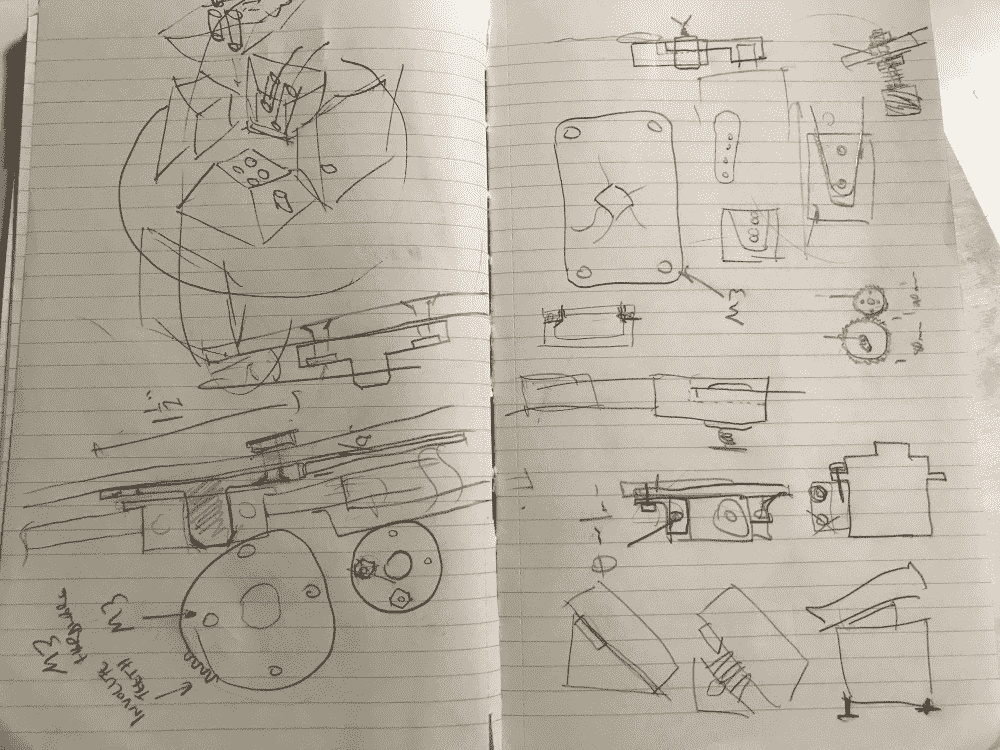Both invigorated by new directions and terrified by my complete lack of progress thus far, I decided to start working immediately. But I was debating between learning a real CAD software like Fusion360, or continuing to use my entirely code-based approach I've been doing throughout the semester.

I realized that some of the fully 3-dimensional geometries aren't that convenient to generate, and I wouldn't be able to receive help from the TA's and classmates. But eventually I decided to stick with my coding: A gun might be more effective, but a swordsman holds on to his sword even in death.

## Gears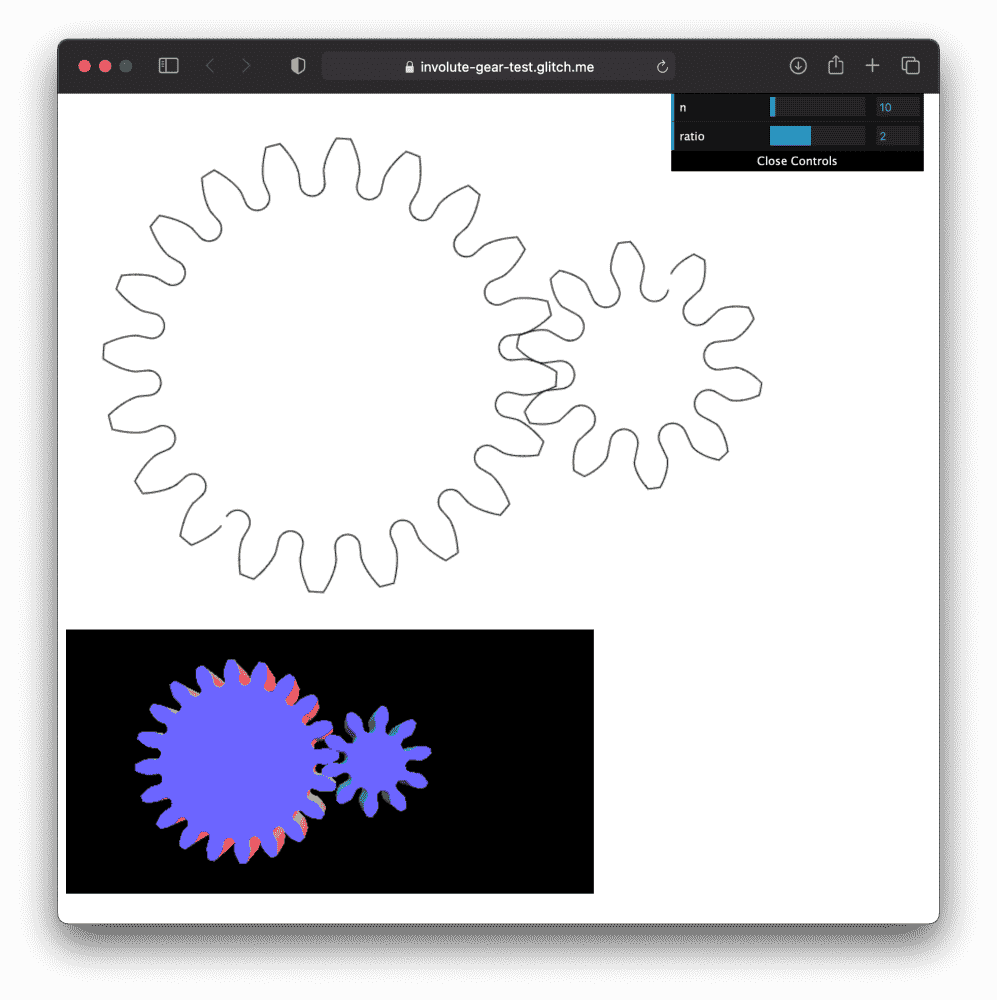I started with generating the gear system. From the machine-building lecture and some google searches I learned that “involute” gears are the “best” gears. So I decided that that's what I'm going to make. I learned from Wikipedia that the curves of involute gears are determined by the parametric function:

X(t) = r (cos t + (t-a) sin t)
Y(t) = r (sin t + (t-a) cos t)


So simple! But, it seemed that there was much more involved to generate the final shape of the gears. The biggest question was how to determine the angle between the tip and the root of a teeth (i.e. skinny teeth with fat gaps, or fat teeth with skinny gaps). There seemed to be a definite answer; otherwise the teeth of two gears apparently wouldn't fit.

I tired to search the internet, but it seemed that everyone was talking about how nice involute gears are, and the perfect properties of involute gears, and nobody cared that HOW DO I ACTUALLY GENERATE ONE???

Time to put my brain to work. I figured that the intersections between the “pitch circle” and the teeth should form equidistant segments to make two gears “mate” each other. How to intersect the involute curve with a circle? I searched the internet again to see if someone had come up with a closed-form solution, but it seemed that numerical methods was the way to go.

After putting together all the math, I managed to generate two identical involute gears that perfectly mate each other (single contact point blah blah). However, when I decreased the size of one of the gears (as required for my project), the gears started “overlapping”. What?? I checked all the maths and read all the descriptions of involute gears the Internet had about them again and again, but couldn't figure out an error in my algorithm.

Eventually I found out by digging into the guts of a gear library someone else implemented. It turned out that the “base circle” is different from the origin of the involute curves. Basically, to avoid the “overlap” problem I had, the involutes don't grow directly from the circle, but are instead offset from it by some amount. What?? I spent much time staring at all the math, but the answer was just an offset. No source I read mentioned about any “offsets”.

But anyways, I had my gears, at the price of a couple hours of head-scratching. I found it interesting that, as an artist, I've drawn many gears in my illustrations, but this was the first time in my life, that I drew a “real” gear system that was actually functional.

You can try my interactive gear demo online here.

## Parts

Next, I need to design a “hole” on one of the gears in the shape of the horn of the servo, so that the gear can be fastened onto the servo. I learned from Zach our TA that:

• Design for fastening onto the horn, and not onto the spindle.
• If I scan the horn in a scanner, no measurement or guesswork will be necessary.

I own a 80 color scanner/printer that can scan at a surprisingly high 600 DPI, so the latter sounded like befitting approach. After scanning the horns, I processed them in an image processing software. To align the shapes perfectly in the center, I generated a “shooting target” image with some simple script, which I superimposed onto the scan. Finally I thresholded the image, so my blob-tracing algorithm can extract the outlines.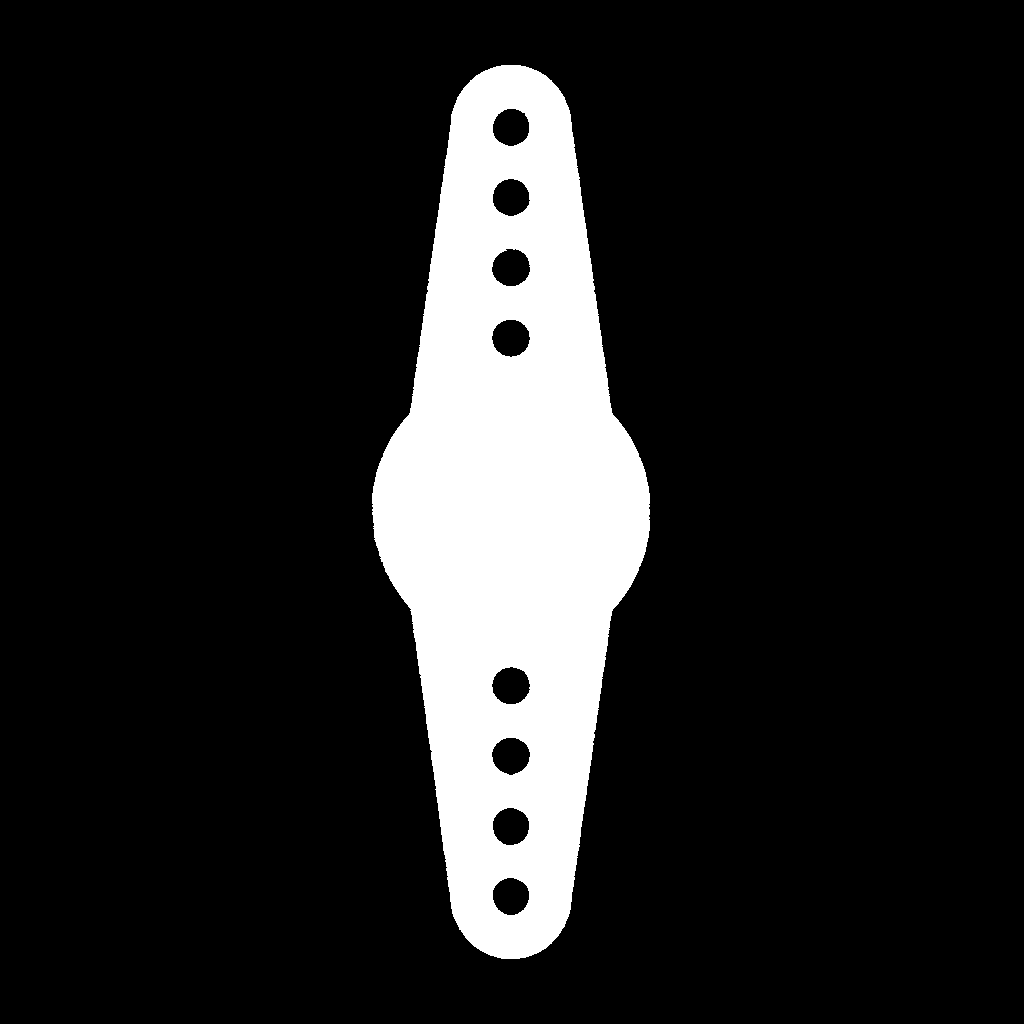Generating the 3D components was a bit of a challenge. Though in previous weeks, I generated a lot of 3D designs, they were usually derivable from 2D/2.5D processes, or served decorative functions so prettiness was the only major concern. This time, would I be able to generate fully 3D components that are mechanically functional? The answer was yes, but it was “CPU-intensive” for my brain. I wouldn't really recommend to anyone else, if they're not used to thinking about vertices, normals and triangles in three dimensions all the time. Below are the initial set of components I generated. From top left: bearing gear, servo gear, bearing bracket, servo arm, servo bracket.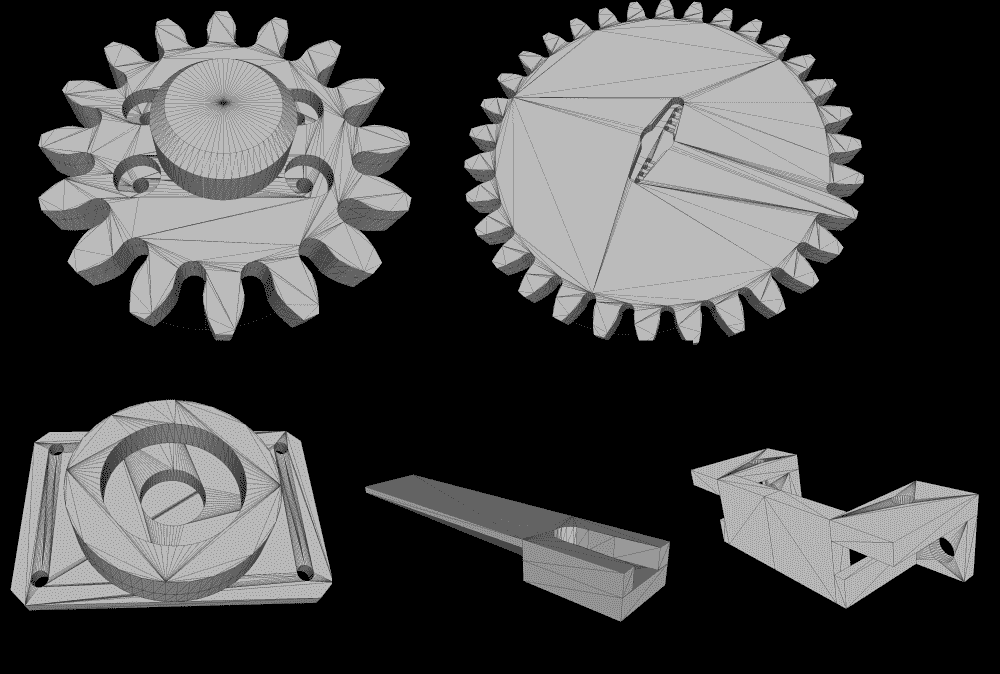You'll see below how I had to iterate upon these designs. ## Fabrication & Iteration I've never operated a 3D printer before, since in the 3D printing week I was sending my job to the Stratsys J55 via Tom, the only person authorized to operate that particular printer. Now I finally get to learn using the Prusa MK3 printer. It turned out to be extremely straightforward. I just slice my STL in Prusa's software, save it in an SD card, and plug it into the printer. I don't even need to calibrate or zero or anything. So boring.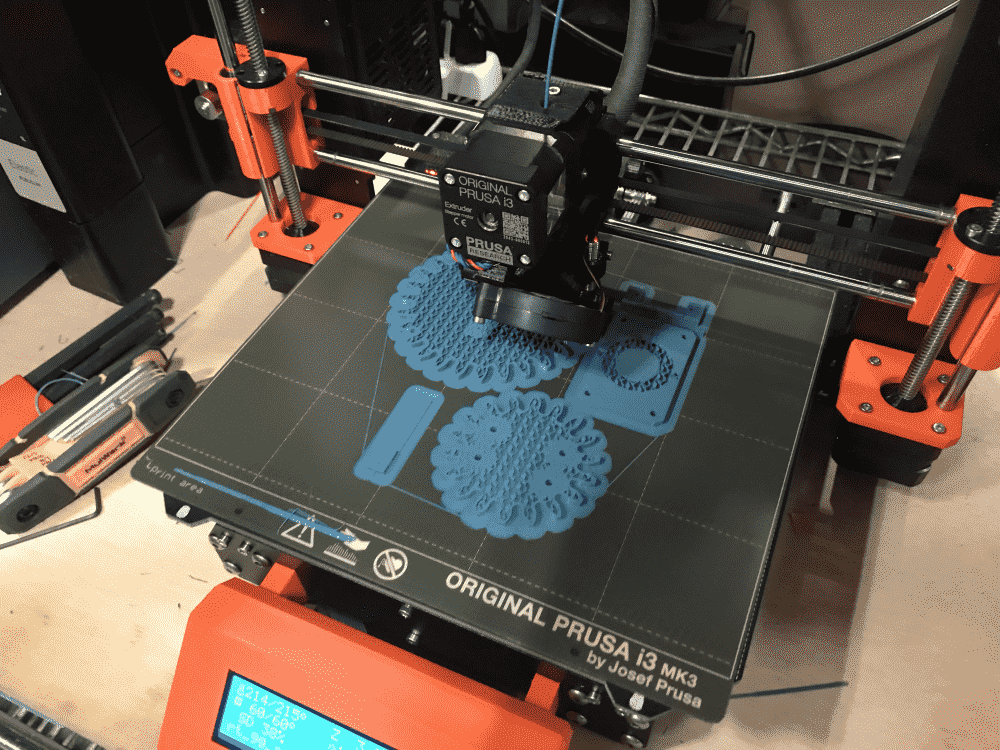However there were two problems. I initially didn't pay attention to the estimated time given by Prusa software, and the default setting was too fine, that my first print was going to take 7 hours to complete. The second problem was that while designing the parts at home, I didn't have access to a caliper, so everything was measured on a plastic ruler I had from my childhood. When the 7 hour job finally completed, none of the parts actually fit the servos and bearings I had! I remeasured the servos and bearings with a real caliper, and retried printing. I used the 0.2mm (SPEED) and set the infill to 15%. I also decreased the physical size of both of my gears, since they didn't actually have to be that large. The prints finally finished in reasonable time, but the parts still didn't fit my bearing so well. I estimated that I could insert them by hammering with a mallet, but I didn't want to, since I was only starting to prototype and didn't want anything to be permanent yet. I wanted a press-fit so perfect that, it could not come loose on its own, but could be separated by a human with relative ease. I forgot if it was the fourth time or the fifth time, that I was finally satisfied with the prints.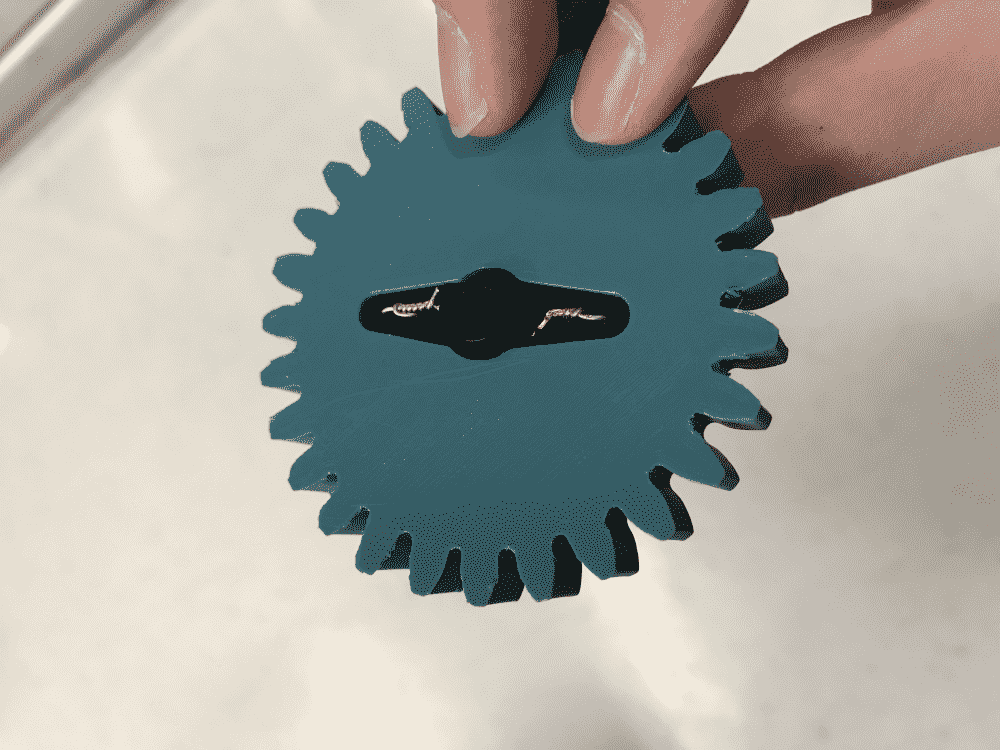I was quite proud of my servo bracket design, which was also improved from the initial one. It is a tad hard to insert the servo into it, but once it is in (by using the elasticity of the plastic), it gets locked in for good. Instead of using screws on holes of the “shoulders” of the servo, I added tiny protrusions on the bracket that press-fits into the holes. It was very sturdy. (Later I further improved them by adding encapsulation for hex nuts, see below). It might sound silly since brackets are such trivial parts, but imagine just a few days ago I was thinking of fastening the servos using duct tape!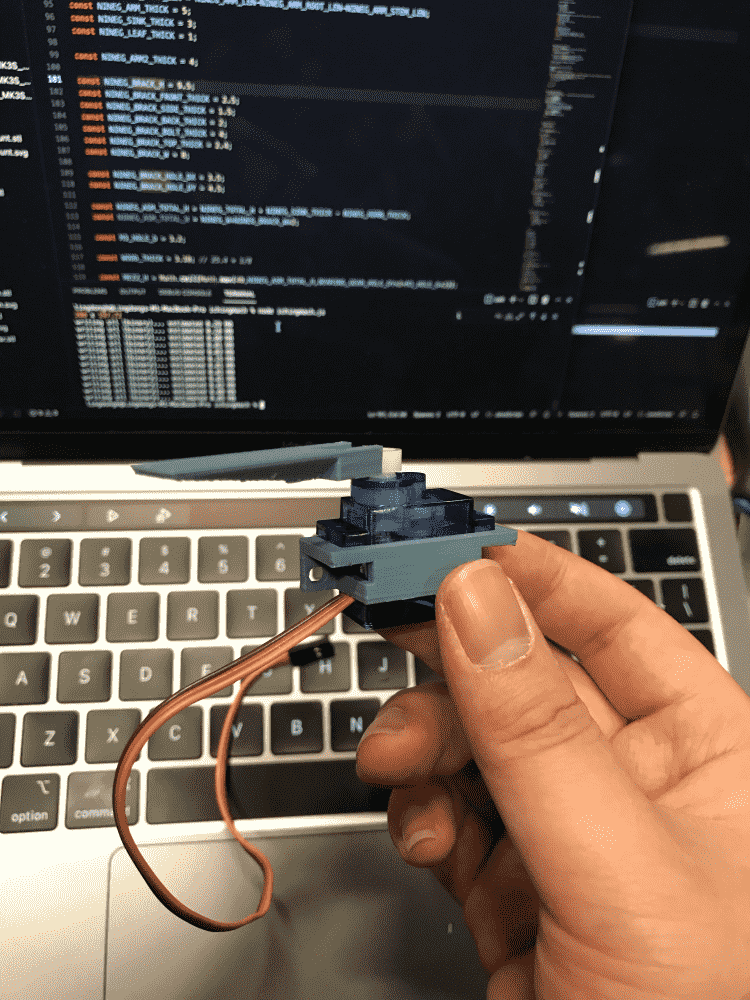I learned that with 3D printing, it is important to pick the best orientation of the object in relation to the bottom plane. Features parallel to the XY plane will have extremely high resolution, and be suitable for any press-fit situation. On the Z-axis -- not so much. Things will look roughly like the shape you intended, but forget about precision. Next, I laser cut two small pieces of wood, for the minimal structure needed to test the gear system driven by the servo. My first gear system was working! The next important step, also one of the most challenging bits of the project, was to test the door mechanism. The doors need to open and close at such perfect speed that, it allow only one ball to fall through at a time. By default, my cheap 9g servos (2 each) rotate quite slowly for small angles. However, I discovered a nasty hack with software PWM.

Basically it goes like this: if you tell it to rotate 10°, it will think “oh that's easy, I'm gonna take my time”, and rotate slowly. If you tell it to rotate 180°, it will think “oh no! that's so far away, gotta hurry!” and rotate very fast. So, to make it rotate 10° very fast (as is required by my project), I trick it into thinking that it needs to rotate (say) 180° by sending the PWM signal for that, but only give it enough time to rotate to 10° by cutting the PWM signal immediately.

To fine tune the speed of the door, I modify the “imaginary” angle I trick it with, the amount of time I send the PWM, and a tiny delay between door open and door close.

However, as you can see in the video above, there is another serious issue. Sometimes, two balls both want to pass through the hole at the same time, and being incredibly rude creatures, they end up clogging and neither can pass.

Alfonso our TA suggested adding a tiny triangle solid above the hole, that serves to guide the balls. It seemed to help a bit, but the balls still clog up from time to time. Alfonso suggested a smaller triangle; TA's Quentin and Leo stopped by and suggested a bigger triangle. They also pointed me to the Marble Machine. That was a huge encouragement. If someone could make this crazy machine that moves thousands of balls, my crappy little machine that moves 50 balls could definitely be made to work, somehow.

In the end, I decided to fix this problem with a lazy way. I found that if I shake the box after every ball has passed through, it would unclog the hole (as shown in video above). Since the box is supposed to be rotating by design, I could just add this additional routine to the bottom servo in my program. It makes the process a lot slower since every ball falling has to be followed by a shake, but the hack seems to work reliably enough.

In the video below, you can see it in action:

So far, I've been simultaneously laser-cutting and 3D printing and programming and assembling, so not a single second is wasted. But I could do more! I started milling a new PCB board for my servos, phototransistors and LED's. My earlier board had enough headers for the servos and OLED, but I overlooked the fact that I also needed ones for phototransistors.

This time, I also added some mounting holes, in case they're useful.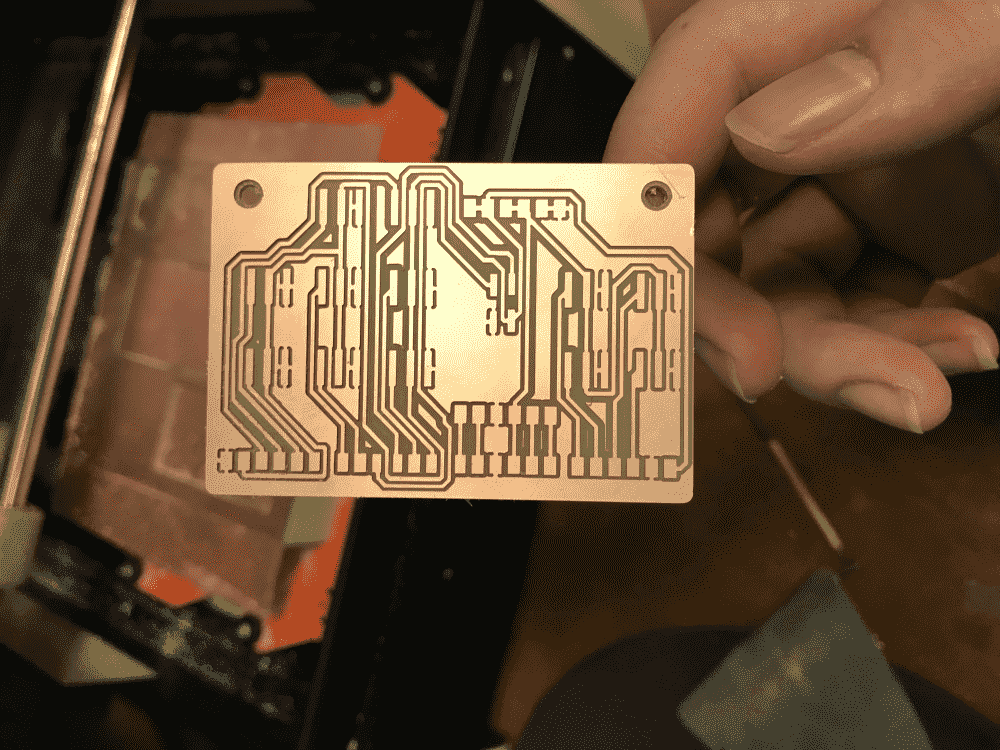The servo board was plugged into my SAMD21E breakout board.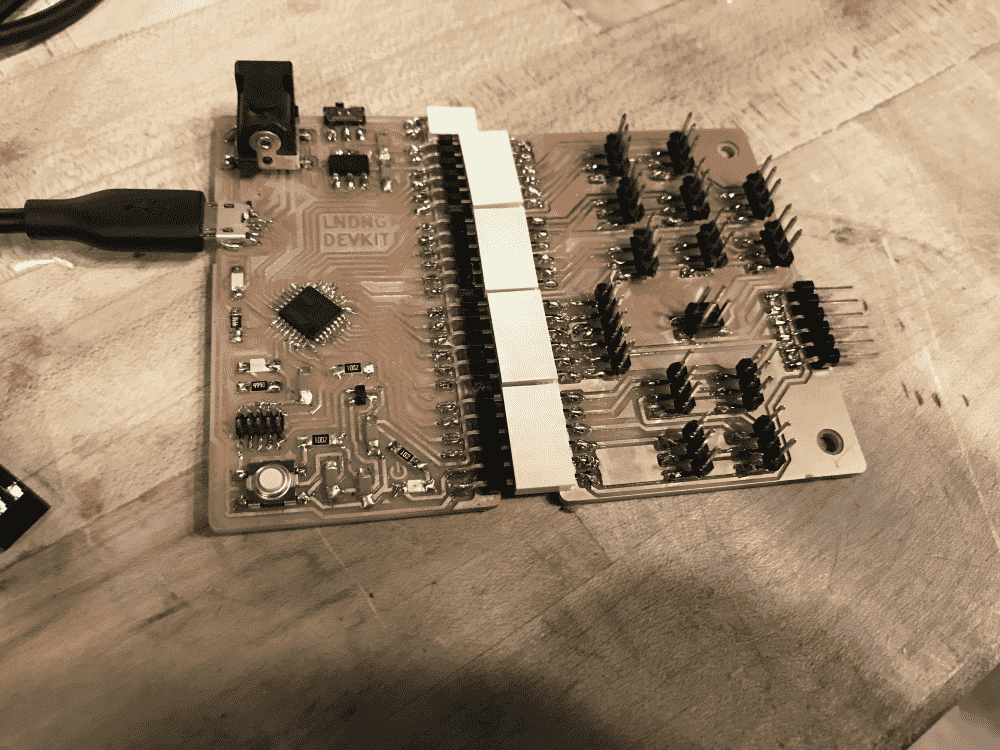It was Sunday night and the Boston subway is always partially down on weekends, so if I tried going home it would take some two hours in a mixture of subway, shuttle bus, and endless waiting. I decided to not go home at all, and worked all night at the lab and take the first train on Monday morning, a decision I would very much regret soon.

For in a tired state I allowed my crazily spinning servo to yank off the microUSB connector along with adjacent traces. At first I was wondering why my board was working sporadically; When I discovered the issue it was too late, and the region was damaged beyond repair.

Confident of my soldering skills I nevertheless tried to repair it. However, it only made the situation worse, as the heat from repeated trials peeled off the traces even more, and now, the board was truly damaged beyond repair.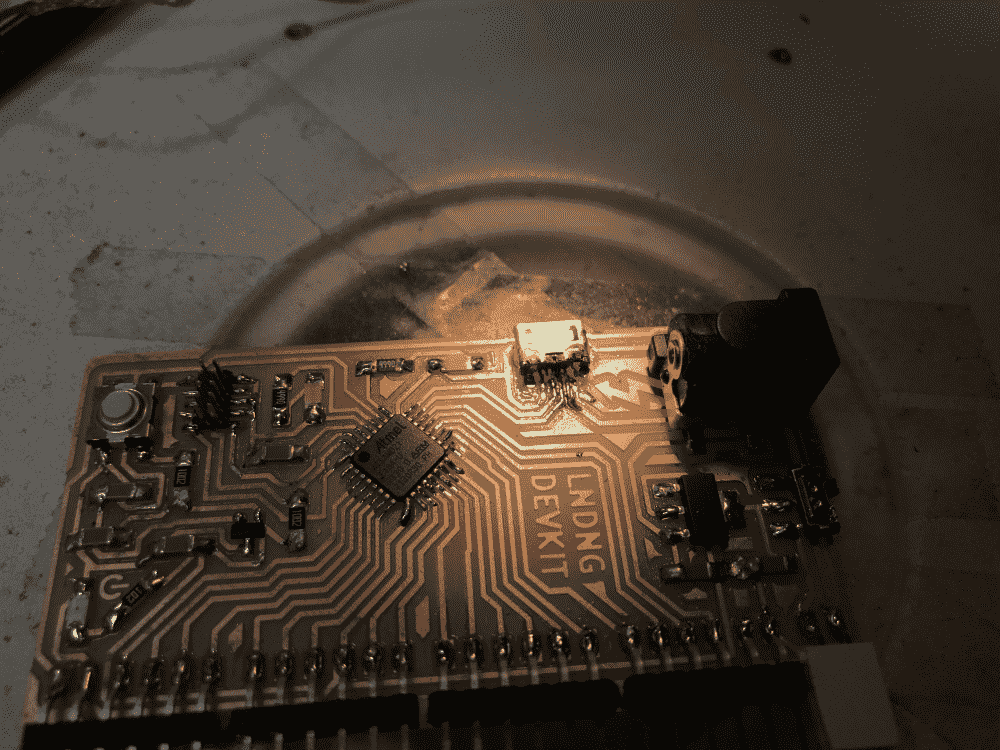Desperately, I tried to solder some jumpers. They were fragile, and I would still need to figure out a way to connect the other end to a microUSB or USB. I gave up.

I went home 7 o'clock in the morning, very defeated and traumatized. Now I don't even have a functional board. Moreover, only few mechanisms of the project were working, and how to figure out the rest was still a mystery. The board received much praise in its tragically short life: people were admiring its beautiful traces; Perhaps as the Chinese superstition goes, it used up all its “good luck” too quickly, so now it had to die.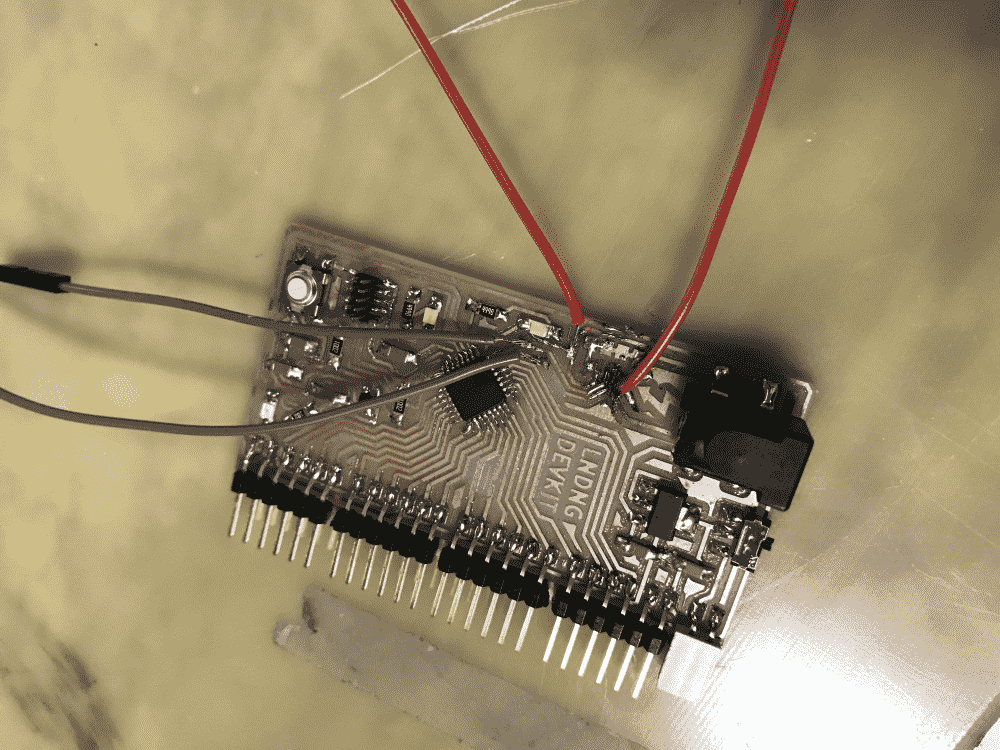After getting some sleep, I was finally partially resurrected on Monday night. I went to school to re-mill my breakout board. I've also slightly modified my design, such that no traces path beneath the microUSB connector, and no padding layer would be needed when soldering it.

However, we were quite out of single-sided boards. The TA's were like: “just use double-sided boards”. But no, I wanted to use single-sided boards for my single-sided boards, and double-sided boards for double-sided boards (if I ever have to design one).

So I scavenged some really bent and worn out single-sided boards riddled with (other people's) failed milling attempts. But since it was not the first time I had to do this due to board shortage, I knew a couple tricks to get it to (barely) work.

However, this time the enemy was the tape (for holding down the boards). The new tape we had were not as sticky as the old tape, and caused the board to nudge left and right while it was being milled. You can see on the right of the below image, how this created a “double impression” effect on the traces.

I found some last bits of the old tape left over, and peeled some tape from old boards, and finally milled a usable board (below left). Not pictured is another attempt that was electronically passable, but had some slight ugliness due to not being cut deep enough at a few places.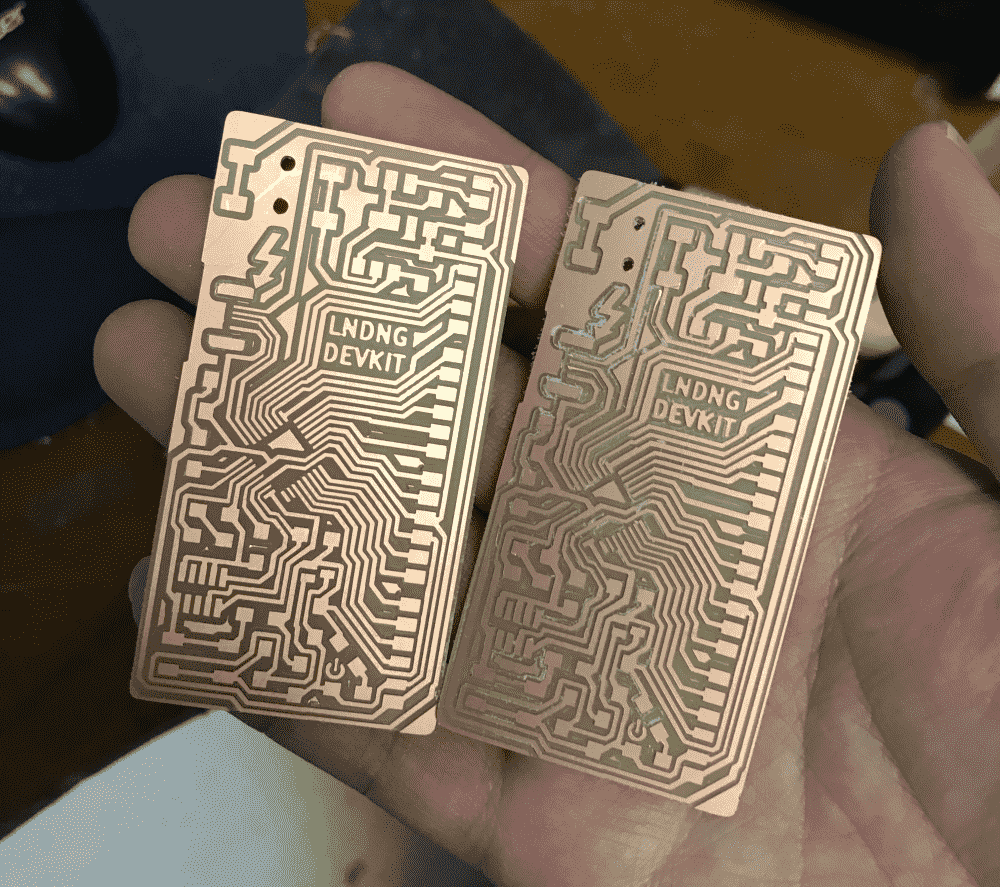With relative ease I stuffed the board. My SAMD21E breakout board returned! I could finally start working on my actual final project again.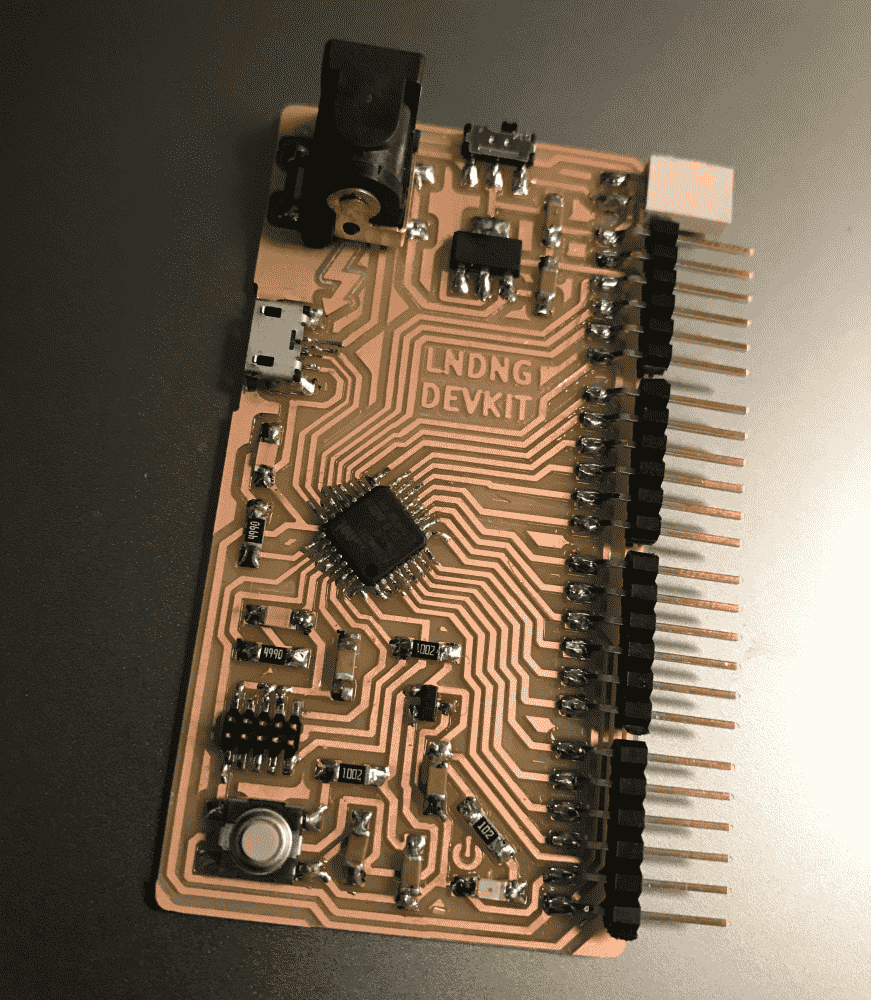But prof. Neil passed by and noticed my naked board. He suggested that I make some protective casing, for all sorts of bad things could happen to it otherwise. It sounded wise, given that a very bad thing just happened to its predecessor. So I spent some time to do that, and printed with semi-transparent filament. I call it the “crystal coffin”.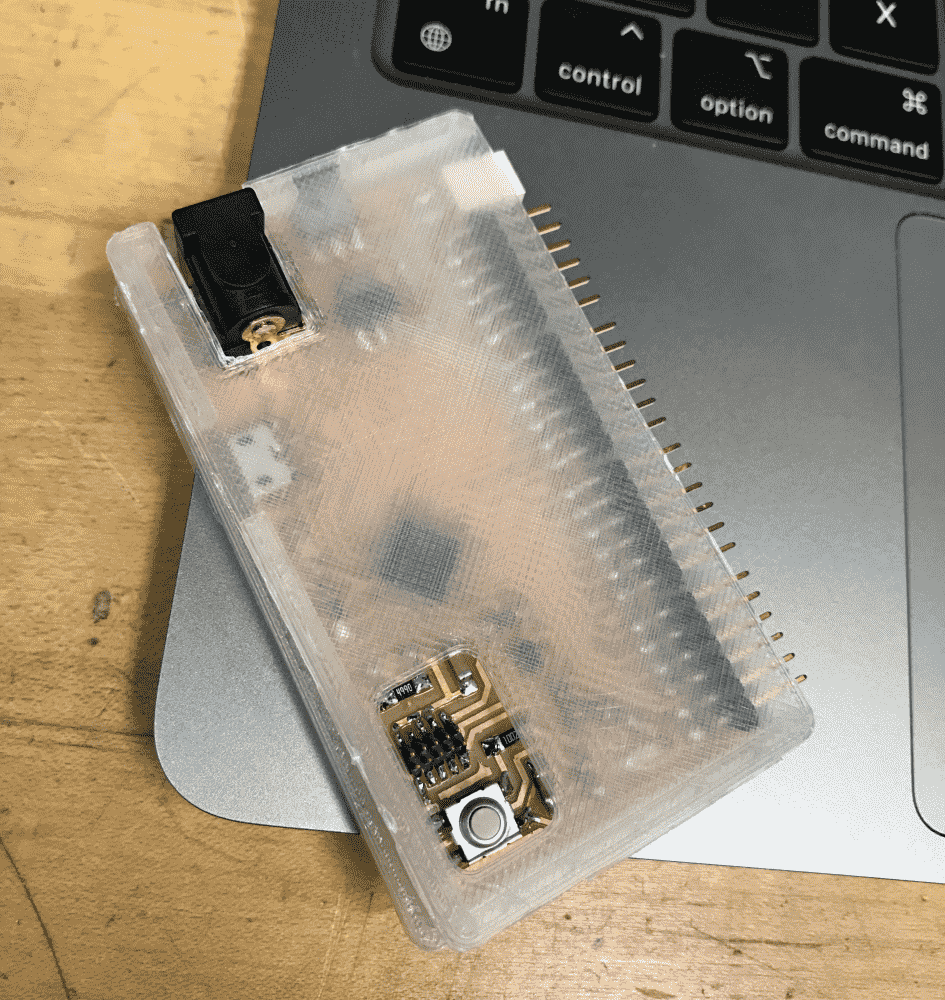The accident of yanking off the microUSB traces made it apparent that I needed to start designing a wiring system. Devoid of experience, I never imagined that wiring could be a problem previously; But now I had a mess of some 37 wires that could tangle, yank, break at any time. The most difficult part, was that the big servo responsible for rotating the entire box, was situated underneath the base, separated from all the other servos; and the power also had to be outside of the box.

Since my box needed to rotate more than 180° (around 240°), it seemed that regardless of whether the PCB is on the box or outside of the box, yanking would be unavoidable. The only method at the top of my head at the time, was to make a giant tube hovering over the box, through which all the wires in the box would go. It would be quite clumsy and ugly.

I consulted my classmate Reina, who pointed out that I could make a U-shaped rail on my base, so the wires can slide gracefully without being trapped onto anything when the box rotates. Thanks, problem solved!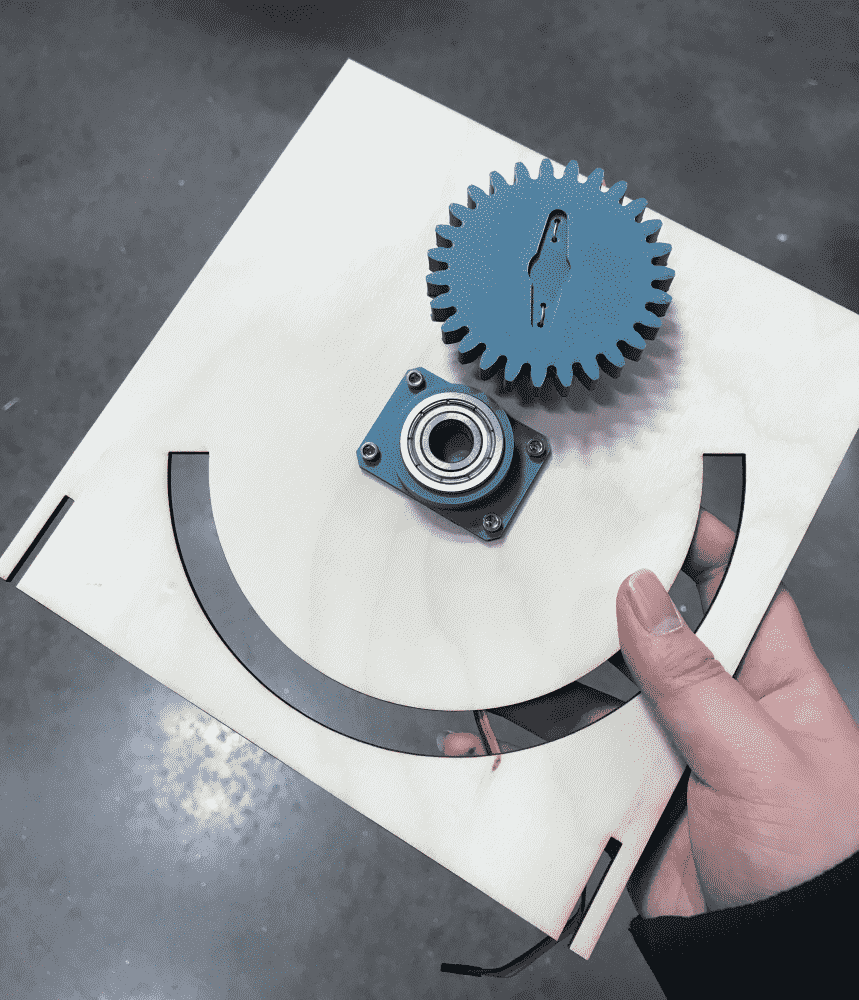The 180° rail made the base slightly wobbly, but I figured that the box isn't that heavy anyways -- in any case, I could make some additional supports to mitigate the issue.

Seeing my project quite on track now, I decided to make another board that contained six buttons. I estimated that I would need to zero, calibrate, debug, change settings for, and trigger different functionalities of my machine, and some sort of “TV remote” would be nice to have.

I used SAMD21E's imbecile cousin SAMD11D -- a bulky microcontroller with plenty of pins and very little brain -- perfect for just reading a bunch of buttons (and sending the events via serial).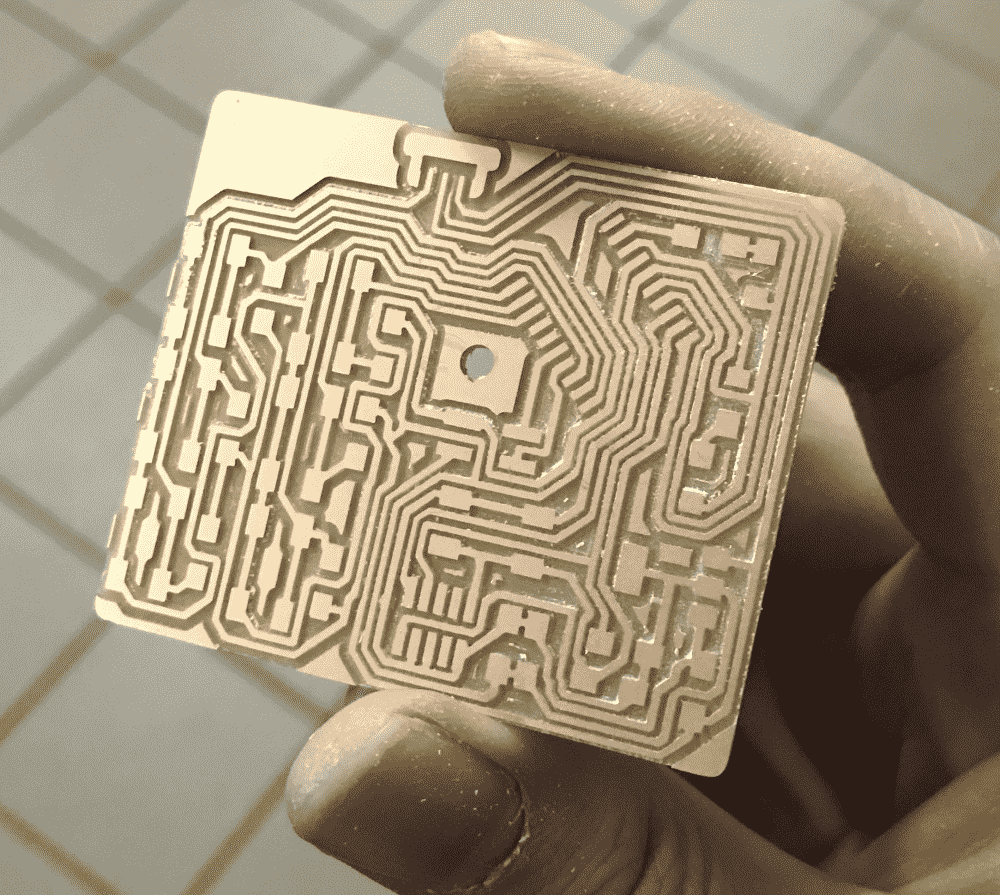While stuffing the board, I figured that it's always better to have more backups of my main breakout board in case anything goes wrong again, so I milled yet another one. Look at this extreme alignment! Next time you hear the professor or the TA's complain about how we're wasting the boards, you know that they're not talking about me.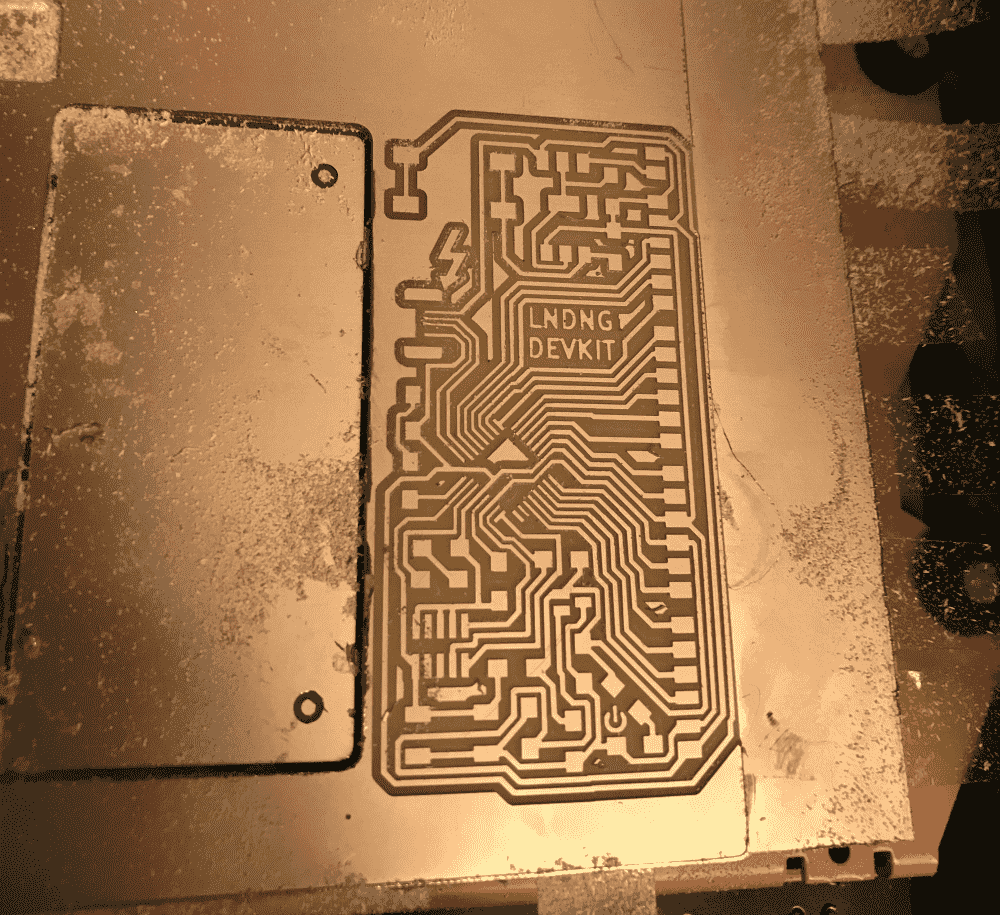I discovered that the male FTDI pins are easily bent when I try to unplug the breakout board from a mating board: There's too much friction, and when unplugging, it is easy to pull at a slight angle causing the last pin to be damaged.

I had to replace the pins at one point, but soon it started bending again.

This wasn't a problem previously since I've only used 6, 12, or 18 FTDI pins. The current number, 24, was starting to hit the limits.

Unable to think of a better idea, I considered putting the female headers on my more precious, harder-to-make breakout board, and putting the male headers on the rougher, easier-to-make servos board. However, that would render the mating boards I've made previously for my breakout board series useless (without jumpers). Then again, I already have a male version; and this is for the final project; and the more I wait the more painful the eventual change will become; so it's probably a good time to perform a gender-reassignment surgery on my design.

Meet the fraternal twin of my SAMD21E breakout board: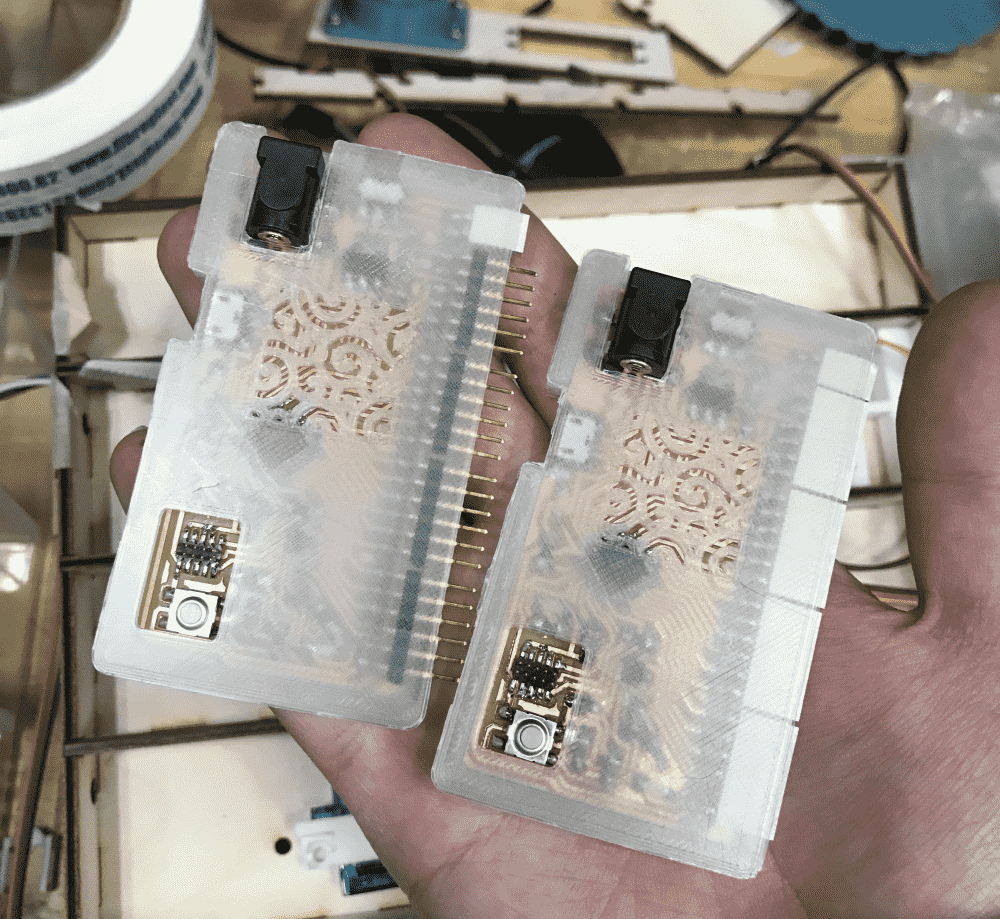Incest!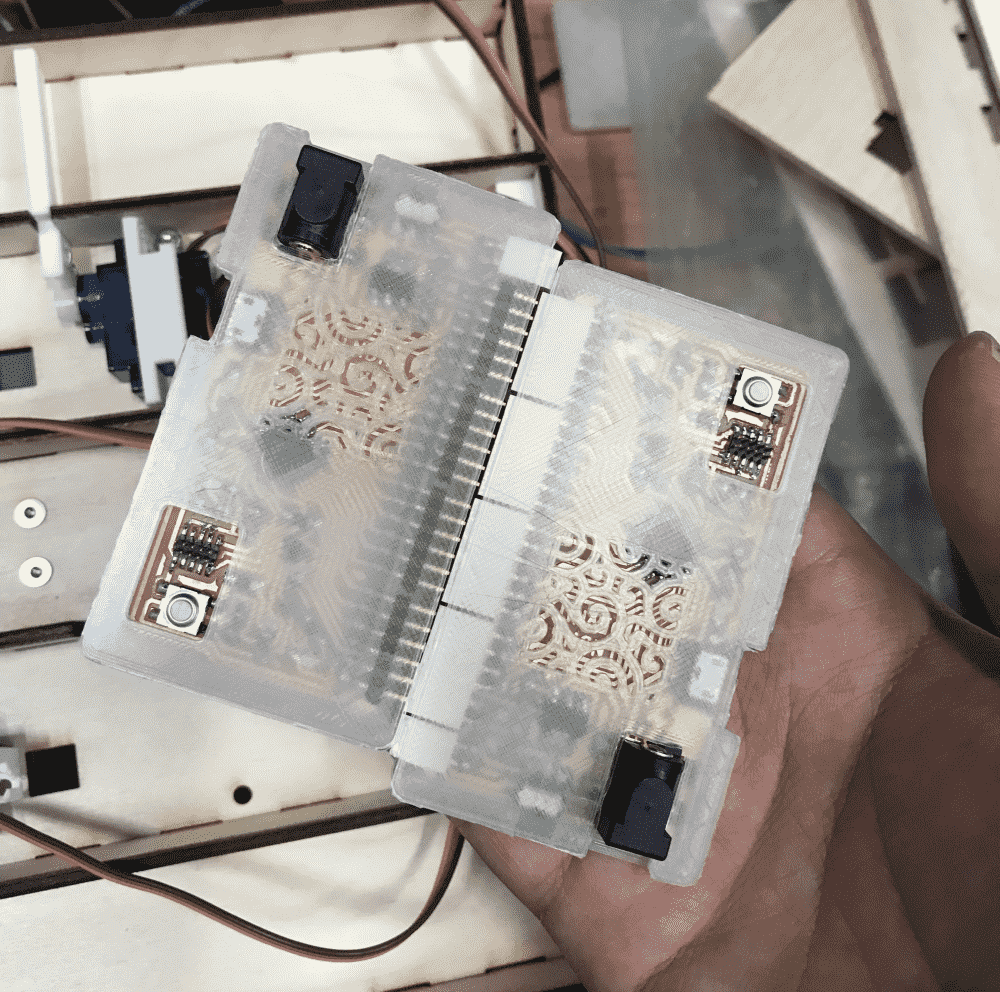Finally I put together all the parts to test if they work as a system. (The servo board was also remade into a more compact, masculine version to mate with the female breakout board).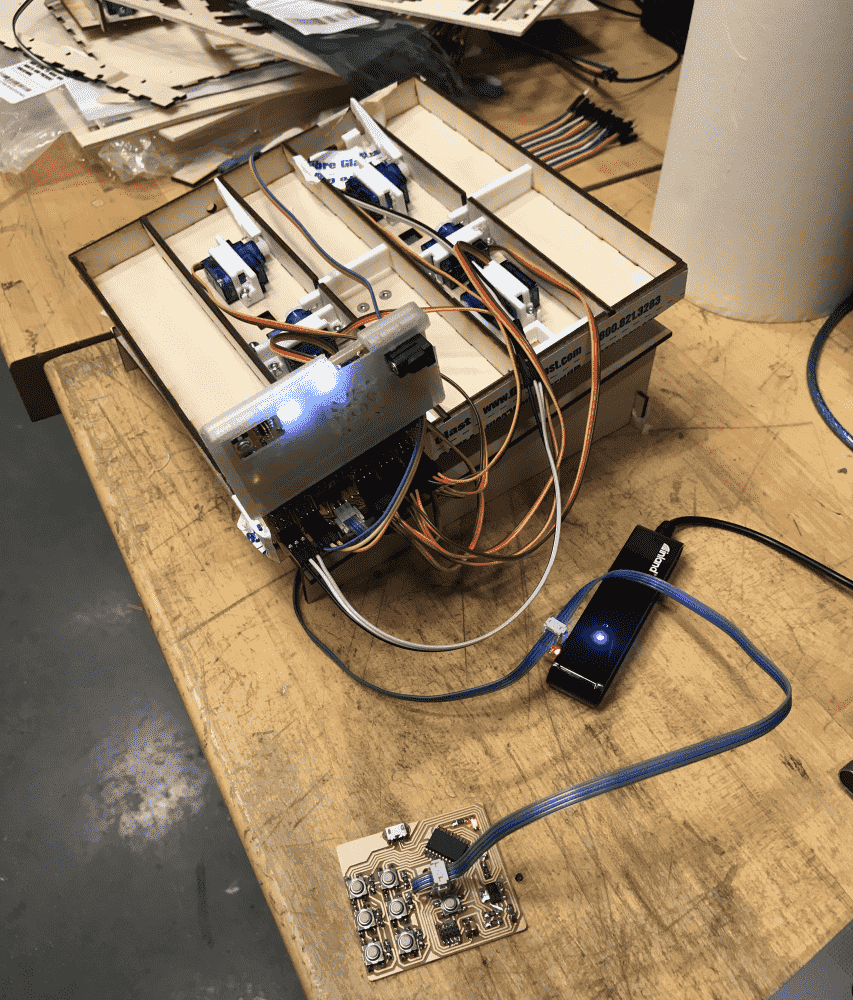In below video you can see the basic mechanism of the machine working. It doesn't include the phototransistor/LED tests, but at that point I figured out a reliable solution for them, which will be explained soon.

On Wednesday, prof. Neil showed us projects from previous years with nice packaging and system integration, so I became anxious about my crappy masking-taped box of wobbly electronics.

Now that my mechanisms are mostly working, it is time to create a pretty-looking box. Which was pretty straightforward. I tried to laser-cut some clear acrylics for the front panel, but with the wrong settings I managed to cut my hand instead (when trying to tear apart the halfway-cut acrylic).

The patterns on the pieces that cover up the servos and wires were procedurally generated. They were very simple. Initially I planned for an aligned pattern of rhombi and grids inspired by historical sword decorations when the divination technique was popular, but made a mistake that caused misalignment. I fixed it, but found the misaligned version to be more visually interesting, so I went with that.

The patterns were then laser-cut in raster mode. I tweaked the settings a couple of times to get the perfect darkness.

I made some mistakes with the Z-dimension of the box (since the front panels were later additions), but with the foresight of buying more plywood then I can use, no disastrous consequence was suffered.

I carefully designed holes for wires to pass through.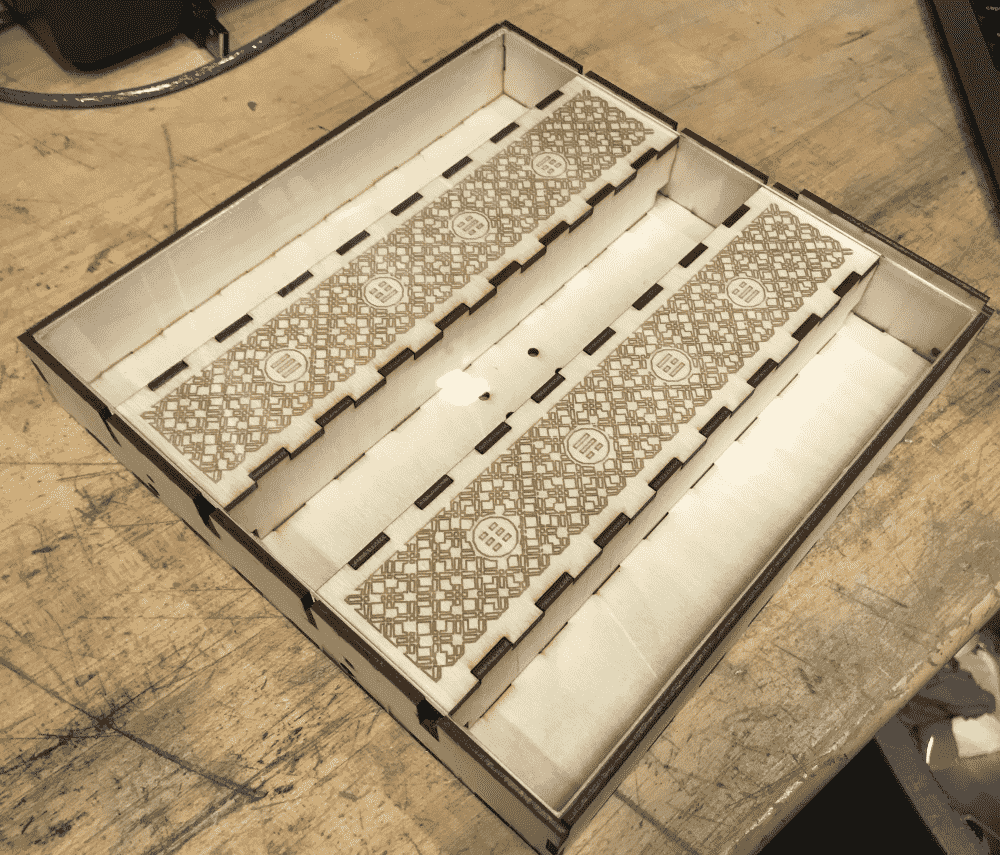Next I printed brackets for the phototransistor and the LED. They're mostly similar, the most noticeable difference being the direction of the thingy on which the screw goes.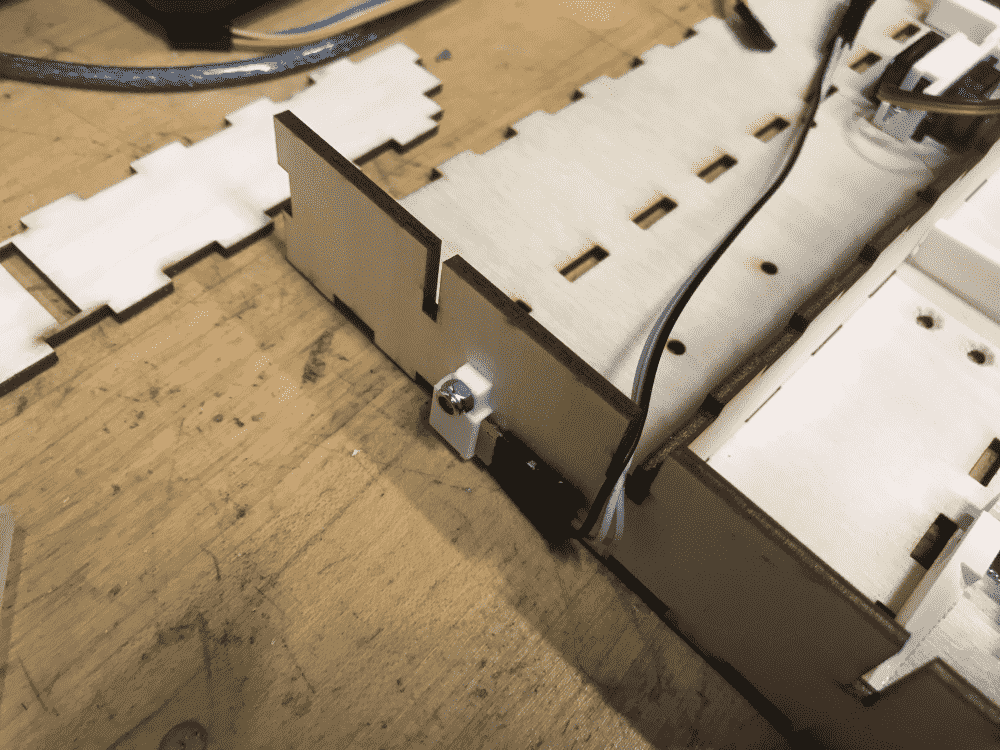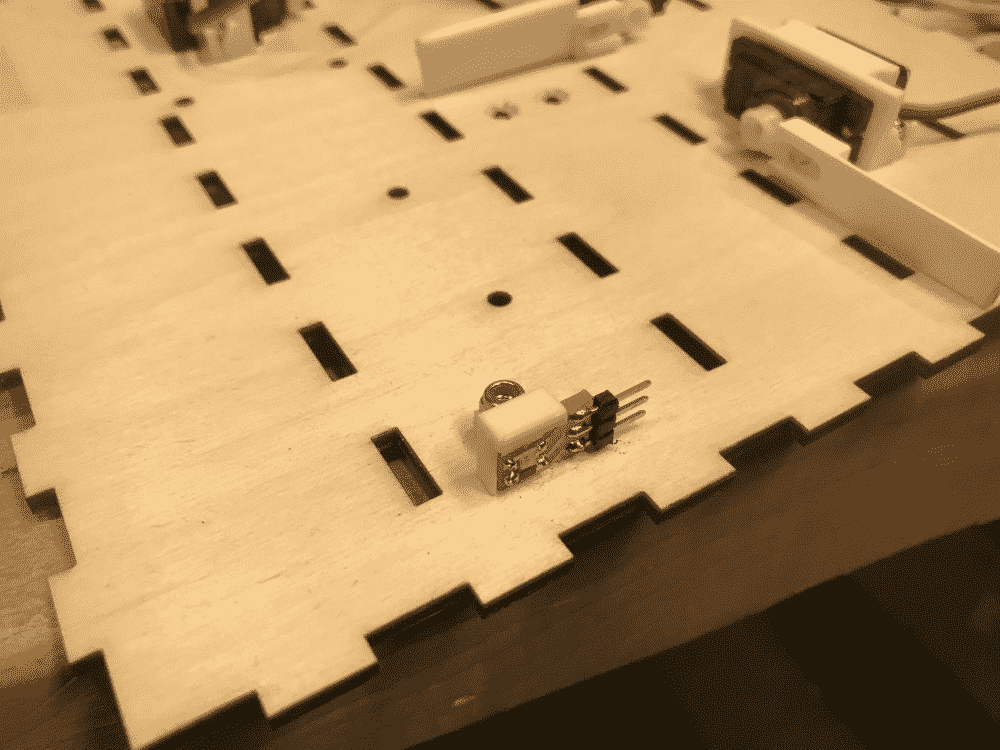I spaced them just enough for a ball to pass through. Perhaps even slightly too closely, that I had to sand the black thingy on the header pins a little bit for balls to pass through without ever getting stuck.

In the image below, you can also see my improved servo bracket, now with encapsulation for hex nuts. Encapsulation for hex nuts is such a great idea; No wonder both Alfonso and Zach independently recommended it to me. It basically turns a difficult two-person job of fastening a bolt into a simple one-person job; it also turns unfastening the bolt from an impossibility to a piece of cake.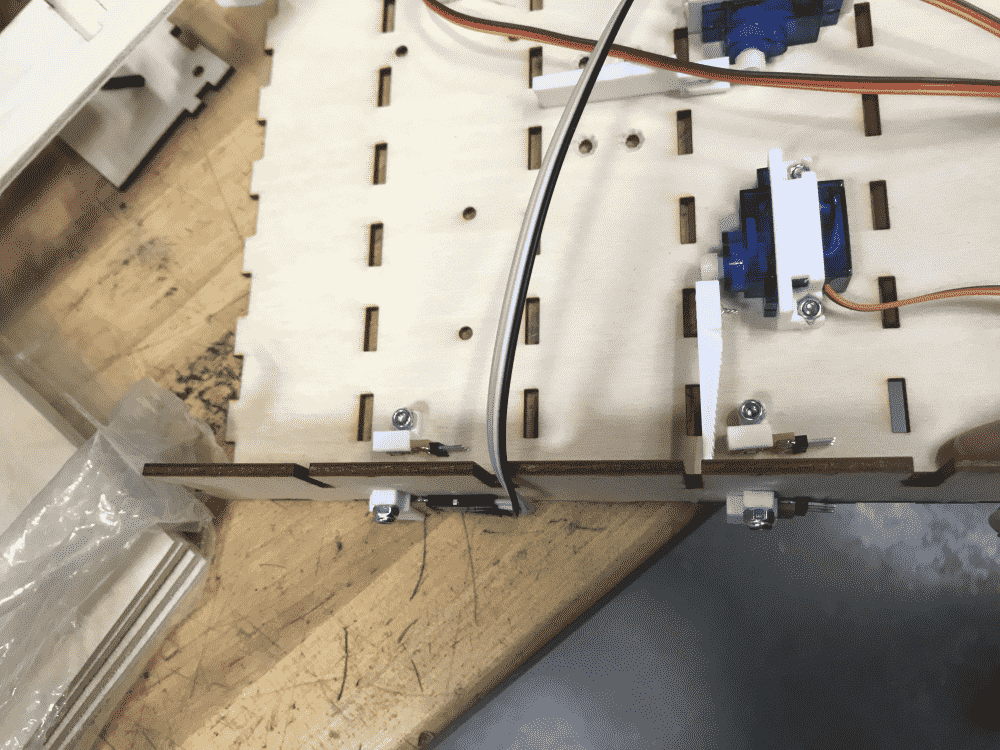Most of the electronics had been added to the new box. Closing the lids, things looked pretty nice.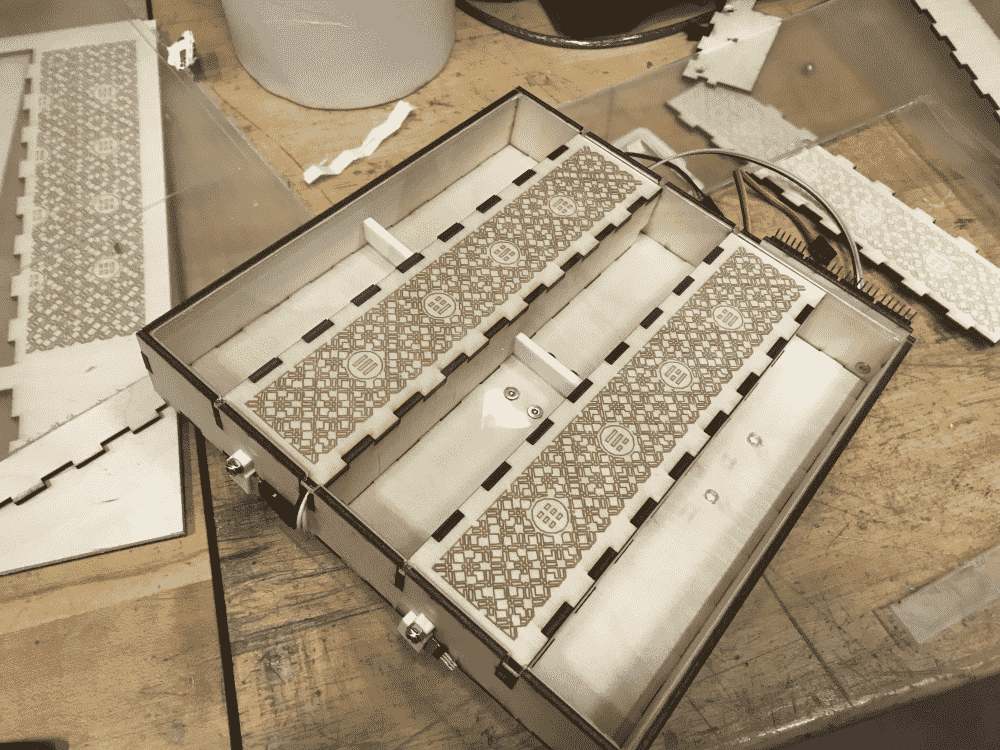Now that I've shown the final installation of the LED and phototransistor, I'd like the explain previous issues I had with them.

Initially, I had problems getting the phototransistor to detect the blocking of the LED by a falling ball. The earlier design was to have the phototransistor fastened to the outside of the box, looking at the LED through a hole on the wall, while the LED is situated on the other side closer to the servo door. The problem was that, the detections weren't that good. Maybe the ball was too fast, or due to some optical misfortunes the metal ball didn't reduce much of the perceived brightness. There was only around 0-10 difference in the reading, in a range of 0-1023: indistinguishable from noise.

Without this mechanism working, my project would be completely useless.

I simultaneously started trying two things: the first was to make an IR LED and phototransistor pair; the second was to swap the location of the LED and the phototransistor.

The first didn't work out: the IR phototransistor seemed to act just like a less sensitive visible-light phototransistor, while the IR LED has no effect on it. Unfortunately, being a pathetic mortal, I am incapable of seeing infrared, so apparently, there was no way to debug it. I asked Alfonso and Zach, neither of whom possessed this superpower, and suggested that I borrow an IR camera instead.

Before I managed do that, I found the second solution, the swapping of the position of the LED and phototransistor to work extremely well. It seemed that when the ball passes the peep-hole on the wall, it almost completely eclipses the LED, giving the phototransistor a drastic change in reading, over 900 in the range of 0-1023! That's about the difference between heaven and hell.

See slow-motion video below of my “auto-calibrating” sensor system. Before any action happens, the LED flashes once, and the phototransistor records the average brightness over a short duration. Then, just after the ball goes through the door, the LED flashes again, and the phototransistor records both the average and minimum brightness over a short duration. Finally, the three numbers are compared to reach the conclusion of whether or not a ball has passed.

The formula I used was

v1_avg * 0.2 + v1_max * 0.8 > v0_avg + threshold


Note that larger the value, darker it is, due to the way the phototransistor circuit is connected. The darkest value has a weight of 0.8, because the ball might be fast, and only blocks the light for a duration shorter than the recorded duration; while the average value has a weight of 0.2, to balance out any accidental noisy anomalies. The detection duration is 200 frames, and threshold is 80 (playing safe here, any number between 70-700 probably works). All numbers were empirically picked and seemed to work sufficiently well for my use case.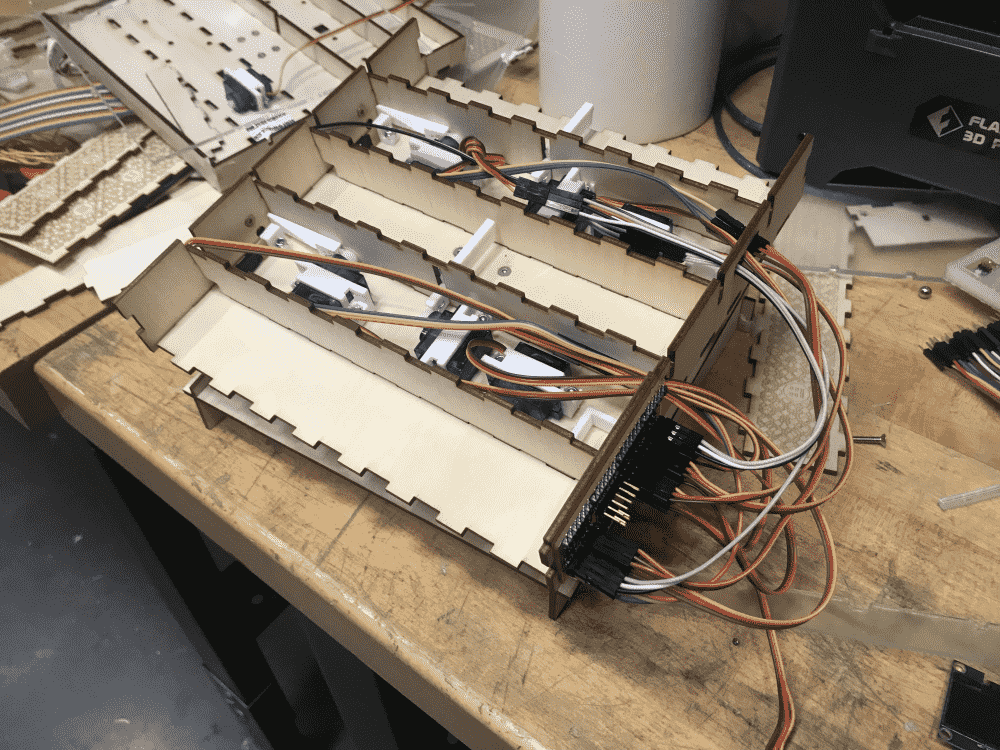I added the rest of the electronic components. What a complete mess of wires it is in the interior! But when I showed it off the others, they all sneered and said that it was mild compared to wiring of professional machines.Loading up the balls the machine was looking and working nicely. Notice that the whole assembly was held together by 4 pieces of masking tape on the box's corners. It turned out by taping these corners all the pieces were well constrained. By not permanently glueing the box too soon, I can debug the machine more easily.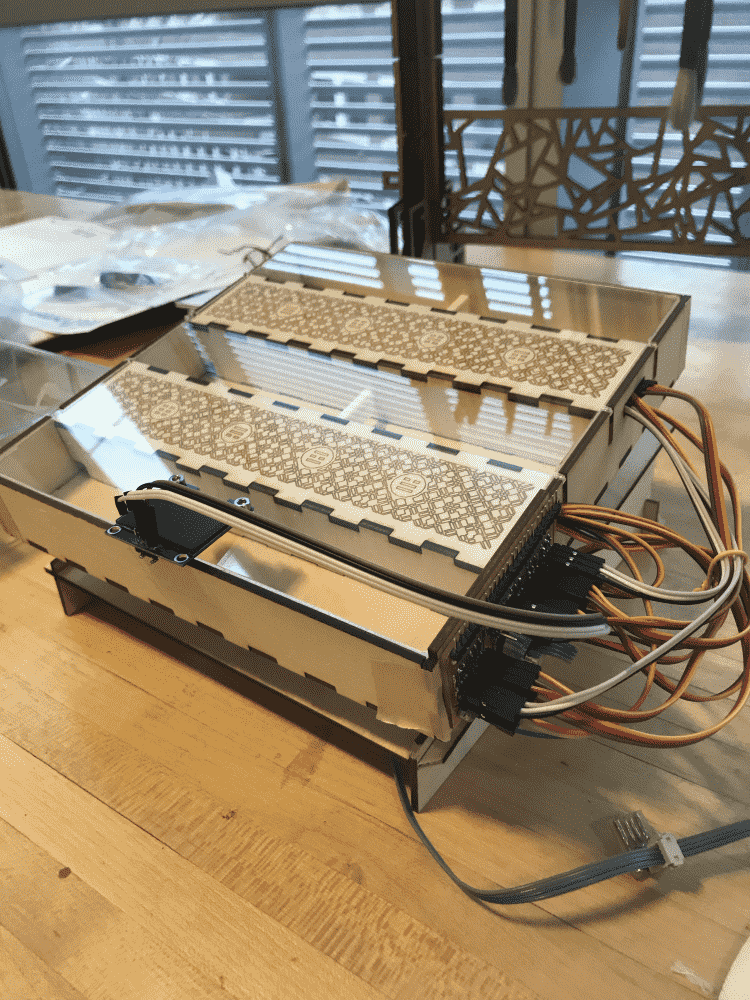The final piece of electronics was the OLED display. Our section seems to be out of OLED's, but being a cunning person I saved one for my final project, but being a forgetful person I left it at home; Being an impatient person I ripped one off of the traces from one of my old PCB's (just kidding, it fell off by itself, it seems that it's a thing with those 2.54mm headers that they always end up falling off and ripping the traces).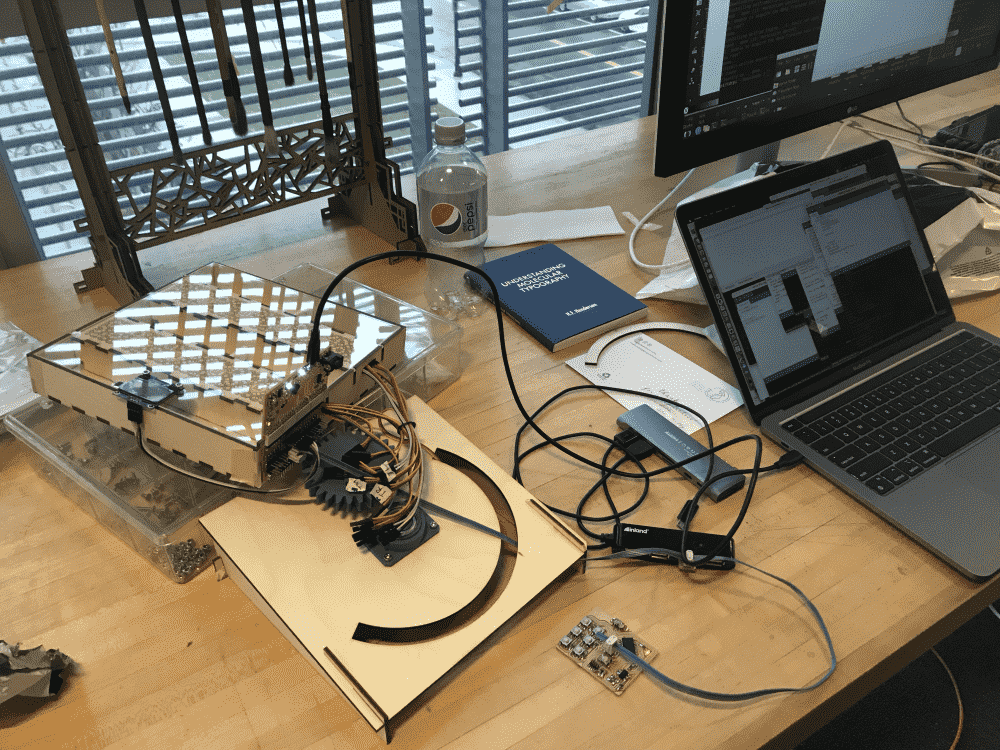The next morning I brought the pristine OLED from home and fastened it onto the designed holes on the acrylic panel. Finally time to start programming the machine!

However, there was one issue. The initial rotation of the servos were unknown, so it was necessary to either come up with zeroes for the servos to move to, or figure out the zeroes the servos were currently at. I ended up taking a combination of the approaches, because angle between adjacent teeth on the pinion of the servo was larger than the the precision I needed, so I had to install the horn at an angle close to zero, and programmatically turn the servo a tiny bit to an exact zero.

It took me more than an hour to zero all 6 servos.

Below you can see a video of my “zeroing” routine, returning all servos to zero. It is quite important, because when the machine is unplugged and plugged to power, all servos tend to jerk or twitch for a split second, and end up at non-zero rotations. I heard it's just a thing with servos.

Also notice that I 3D-printed a casing for the “remote” control, featuring flexture buttons.

Below is a video of me triggering one of the many “self-tests” I designed for the machine, this time for the LED and phototransistors. The LED's are so bright that their flashes are visible from the outside. On my laptop you can see there's an over 900 difference (out of 1023) between light and dark.

Below is a short clip of the machine actually doing divination! For a full run, check out the video here.

I haven't put much work into making some real GUI's on the OLED display (will do soon). Currently it just shows debug messages while the machine is working (like telling you whether it detected a falling ball, or which door it is zeroing, etc.), but in the end, it can draw the hexgram results: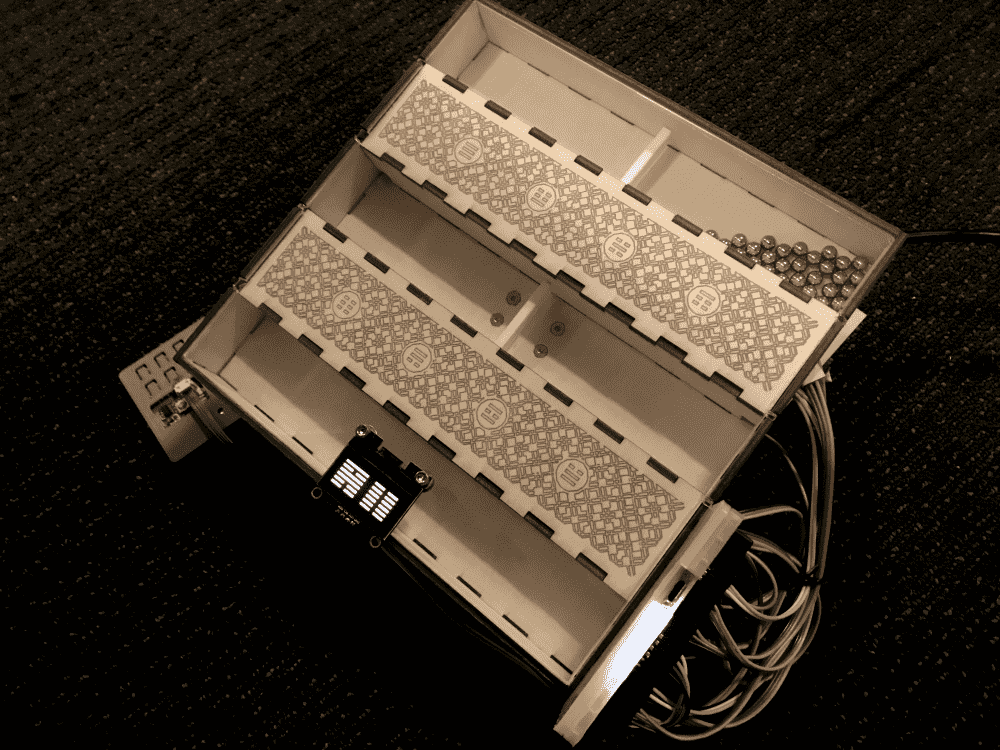## Thoughts

I perhaps spent the most “unproductive” week of my life, working on my final project. Every day for ten days, I come to the lab in the morning to work on the project all day long, sometimes not even returning home at night. Sometimes I entirely forget to eat for a whole day. Yet what have I produced?? A crappy box that wobbles around, that narrowly manages to work for its purpose -- and what's its purpose? Divination. In other words, it's a complete pile of garbage.

If I spent the same amount of time on say, coding or art-making, what complex things I would be able to create!

Thus I often regretted choosing this idea for my final project during these days. It is quite far from the things I usually did and was good at. Perhaps if I just generated some pretty form, with some electronics in it to light or move it in decorative ways, it would probably make a more impressive, and less enervating project.

With this project I had to constantly “fight” the laws of physics. In the end it just looks like some balls falling through some doors (the sensor system is not even noticeable if I'm not there to explain it), but many trials and errors were involved to get these simple mechanisms working.

It seems that I managed to avoid all the things I was good at, and solely used things I had no ideas of before.

But it is perhaps also for this reason, that I felt I learned so much. Before this, I had no idea about what a “bearing” or an “involute gear” or a “countersink hole” is, and my major way of constructing anything is by using cardboard boxes and duct tape. But now I felt quite confident designing and working with those parts, especially since I've myself generated the components from scratch using code. More importantly, I got a taste of the methodologies with which one can turn a vague idea about a machine into concrete steps and tests to take, and components to make. It was like entering a new world.

It would be an exaggeration to say that in this class, I've learned more things than all the things I learned in school added, but it might roughly be on the same order of magnitude. Similarly it would be an exaggeration to say that I've learned more in the final week than the rest of all the weeks added, but it also might be close.

Usually I preferred thinking about questions to asking about them -- but this time there was simply too much I didn't know, that quite a few times I had to ask for help from the TA's like Zach and Alfonso, as well as classmates like Reina -- to them I am very thankful.

Meanwhile, I was also very happy that I tackled many issues on my own. I felt lucky that the project was not a group project, for if this was one, I would surely be working on what I'm most comfortable with -- programming, procedural design, PCB layout etc. and let the engineering kind figure out the physics. As an individual project, I was forced to figure the nasty details of every aspect of the process. Which was great, since I always aspired to know everything.

## Next steps

There are a couple of things I'd like to improve upon if I make another iteration.

Firstly, the largest oversight I had with the current packaging, was that wires are actually objects that physically exist in the real world and one actually has to deal with them. When working too much with the virtual, one starts to confuse wires with the abstract concept of edges on graphs, which is why you see the stream of wires hanging out on the right side of my machine that has no where else to go.

One can argue that they're kinda cool, but I'd also like to try doing it “properly”, perhaps by making the box slightly wider with a skinny chamber on the right dedicated to the PCB and all the wires.

I also realized that the chambers are slightly too small. Initially I calculated to have each of them somewhat large enough to fit all 49 balls -- but when you're trying to roll the balls around, this crowdedness is not helping.

I want to change the material of the box from 1/8" plywood. Plywood is quite easy to work with, but when you make something with it the product just looks kinda cheap and amateurish. Perhaps I should finish the wood. I need to learn how to do that. Or use a different kind of wood. Or perhaps metal or acrylic: electronics and wires don't look that great alongside wood, for some reason.

I also want the machine to look more heavy. Especially for the base, which is currently made out of four thin wood pieces, and starts shifting on the table when the box is rotating too violently. I want something that look more “solid”.

Finally, I want to try using sticks instead of balls, to make the design more “authentic” to the original.

## Conclusion

Despite all the dissatisfactions above, I was satisfied with my contraption. It completes almost all goals I had initially, is a functional divination machine, and all the parts can be generated from a single JavaScript program (which you can find at the beginning of this page, online demo here). So long as one programmer yet lives and reads, the secrets of automated I-Ching divination may never be lost. Perhaps my ancestors will be proud of me (or perhaps mad at me for the sacrilegious interpretation of the procedure).